# JP2010025961A - Image stabilization control apparatus and image capturing apparatus - Google Patents

## Info

Publication number
JP2010025961A
JP2010025961A JP2008183426A JP2008183426A JP2010025961A JP 2010025961 A JP2010025961 A JP 2010025961A JP 2008183426 A JP2008183426 A JP 2008183426A JP 2008183426 A JP2008183426 A JP 2008183426A JP 2010025961 A JP2010025961 A JP 2010025961A
Authority
JP
Japan
Prior art keywords
shake
output
correction
unit
means
Prior art date
Legal status (The legal status is an assumption and is not a legal conclusion. Google has not performed a legal analysis and makes no representation as to the accuracy of the status listed.)
Granted
Application number
JP2008183426A
Other languages
Japanese (ja)
Other versions
JP5274130B2 (en
JP2010025961A5 (en
Inventor
Goro Noto
Junichi Saito
Koichi Washisu

Original Assignee
Canon Inc
キヤノン株式会社
Priority date (The priority date is an assumption and is not a legal conclusion. Google has not performed a legal analysis and makes no representation as to the accuracy of the date listed.)
Filing date
Publication date
Application filed by Canon Inc, キヤノン株式会社 filed Critical Canon Inc
Priority to JP2008183426A priority Critical patent/JP5274130B2/en
Publication of JP2010025961A publication Critical patent/JP2010025961A/en
Publication of JP2010025961A5 publication Critical patent/JP2010025961A5/ja
Application granted granted Critical
Publication of JP5274130B2 publication Critical patent/JP5274130B2/en
Application status is Active legal-status Critical
Anticipated expiration legal-status Critical

• 238000004364 calculation methods Methods 0 abstract 1
• 238000003384 imaging method Methods 0 abstract 1

## Images

•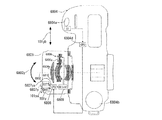•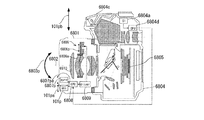•••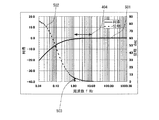•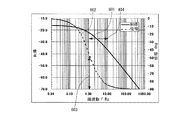•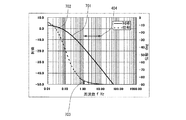••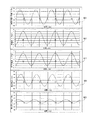••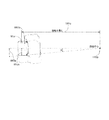••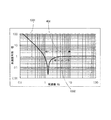•••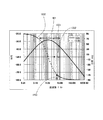•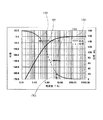•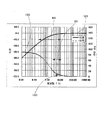•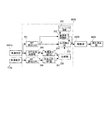•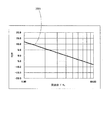•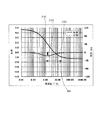••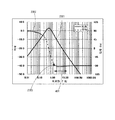•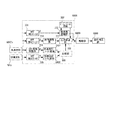•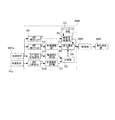•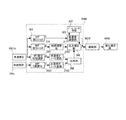•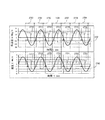•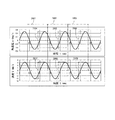•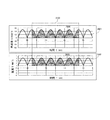•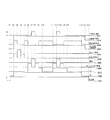•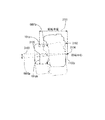•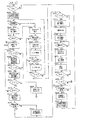•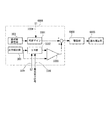•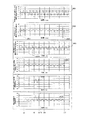•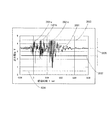•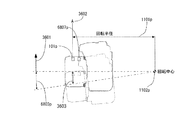•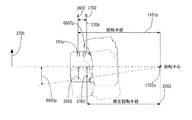•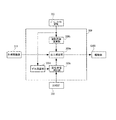•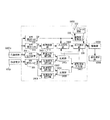•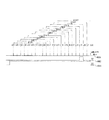••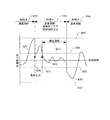•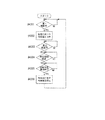••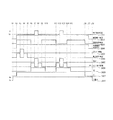•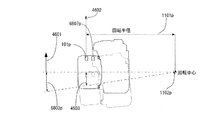•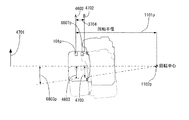•••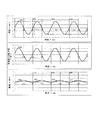•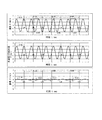•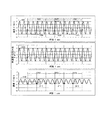•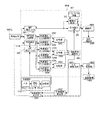•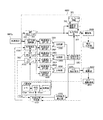•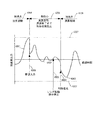••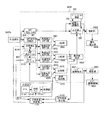•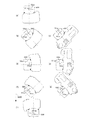•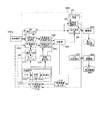••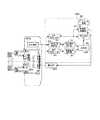•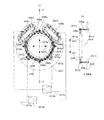••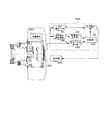•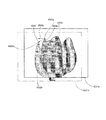•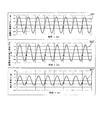•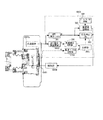•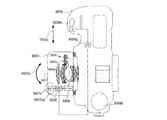•## Classifications

• HELECTRICITY
• H04ELECTRIC COMMUNICATION TECHNIQUE
• H04NPICTORIAL COMMUNICATION, e.g. TELEVISION
• H04N5/00Details of television systems
• H04N5/222Studio circuitry; Studio devices; Studio equipment ; Cameras comprising an electronic image sensor, e.g. digital cameras, video cameras, TV cameras, video cameras, camcorders, webcams, camera modules for embedding in other devices, e.g. mobile phones, computers or vehicles
• H04N5/225Television cameras ; Cameras comprising an electronic image sensor, e.g. digital cameras, video cameras, camcorders, webcams, camera modules specially adapted for being embedded in other devices, e.g. mobile phones, computers or vehicles
• H04N5/232Devices for controlling television cameras, e.g. remote control ; Control of cameras comprising an electronic image sensor
• H04N5/23248Devices for controlling television cameras, e.g. remote control ; Control of cameras comprising an electronic image sensor for stable pick-up of the scene in spite of camera body vibration
• H04N5/23264Vibration or motion blur correction
• H04N5/2328Vibration or motion blur correction performed by mechanical compensation
• H04N5/23287Vibration or motion blur correction performed by mechanical compensation by shifting the lens/sensor position
• GPHYSICS
• G02OPTICS
• G02BOPTICAL ELEMENTS, SYSTEMS, OR APPARATUS
• G02B27/00Other optical systems; Other optical apparatus
• G02B27/64Imaging systems using optical elements for stabilisation of the lateral and angular position of the image
• G02B27/646Imaging systems using optical elements for stabilisation of the lateral and angular position of the image compensating for small deviations, e.g. due to vibration or shake
• GPHYSICS
• G03PHOTOGRAPHY; CINEMATOGRAPHY; ANALOGOUS TECHNIQUES USING WAVES OTHER THAN OPTICAL WAVES; ELECTROGRAPHY; HOLOGRAPHY
• G03BAPPARATUS OR ARRANGEMENTS FOR TAKING PHOTOGRAPHS OR FOR PROJECTING OR VIEWING THEM; APPARATUS OR ARRANGEMENTS EMPLOYING ANALOGOUS TECHNIQUES USING WAVES OTHER THAN OPTICAL WAVES; ACCESSORIES THEREFOR
• G03B5/00Adjustment of optical system relative to image or object surface other than for focusing
• HELECTRICITY
• H04ELECTRIC COMMUNICATION TECHNIQUE
• H04NPICTORIAL COMMUNICATION, e.g. TELEVISION
• H04N5/00Details of television systems
• H04N5/222Studio circuitry; Studio devices; Studio equipment ; Cameras comprising an electronic image sensor, e.g. digital cameras, video cameras, TV cameras, video cameras, camcorders, webcams, camera modules for embedding in other devices, e.g. mobile phones, computers or vehicles
• H04N5/225Television cameras ; Cameras comprising an electronic image sensor, e.g. digital cameras, video cameras, camcorders, webcams, camera modules specially adapted for being embedded in other devices, e.g. mobile phones, computers or vehicles
• H04N5/232Devices for controlling television cameras, e.g. remote control ; Control of cameras comprising an electronic image sensor
• H04N5/23248Devices for controlling television cameras, e.g. remote control ; Control of cameras comprising an electronic image sensor for stable pick-up of the scene in spite of camera body vibration
• HELECTRICITY
• H04ELECTRIC COMMUNICATION TECHNIQUE
• H04NPICTORIAL COMMUNICATION, e.g. TELEVISION
• H04N5/00Details of television systems
• H04N5/222Studio circuitry; Studio devices; Studio equipment ; Cameras comprising an electronic image sensor, e.g. digital cameras, video cameras, TV cameras, video cameras, camcorders, webcams, camera modules for embedding in other devices, e.g. mobile phones, computers or vehicles
• H04N5/225Television cameras ; Cameras comprising an electronic image sensor, e.g. digital cameras, video cameras, camcorders, webcams, camera modules specially adapted for being embedded in other devices, e.g. mobile phones, computers or vehicles
• H04N5/232Devices for controlling television cameras, e.g. remote control ; Control of cameras comprising an electronic image sensor
• H04N5/23248Devices for controlling television cameras, e.g. remote control ; Control of cameras comprising an electronic image sensor for stable pick-up of the scene in spite of camera body vibration
• H04N5/23251Motion detection
• H04N5/23258Motion detection based on additional sensors
• HELECTRICITY
• H04ELECTRIC COMMUNICATION TECHNIQUE
• H04NPICTORIAL COMMUNICATION, e.g. TELEVISION
• H04N5/00Details of television systems
• H04N5/222Studio circuitry; Studio devices; Studio equipment ; Cameras comprising an electronic image sensor, e.g. digital cameras, video cameras, TV cameras, video cameras, camcorders, webcams, camera modules for embedding in other devices, e.g. mobile phones, computers or vehicles
• H04N5/225Television cameras ; Cameras comprising an electronic image sensor, e.g. digital cameras, video cameras, camcorders, webcams, camera modules specially adapted for being embedded in other devices, e.g. mobile phones, computers or vehicles
• H04N5/232Devices for controlling television cameras, e.g. remote control ; Control of cameras comprising an electronic image sensor
• H04N5/23248Devices for controlling television cameras, e.g. remote control ; Control of cameras comprising an electronic image sensor for stable pick-up of the scene in spite of camera body vibration
• H04N5/23264Vibration or motion blur correction
• HELECTRICITY
• H04ELECTRIC COMMUNICATION TECHNIQUE
• H04NPICTORIAL COMMUNICATION, e.g. TELEVISION
• H04N5/00Details of television systems
• H04N5/222Studio circuitry; Studio devices; Studio equipment ; Cameras comprising an electronic image sensor, e.g. digital cameras, video cameras, TV cameras, video cameras, camcorders, webcams, camera modules for embedding in other devices, e.g. mobile phones, computers or vehicles
• H04N5/225Television cameras ; Cameras comprising an electronic image sensor, e.g. digital cameras, video cameras, camcorders, webcams, camera modules specially adapted for being embedded in other devices, e.g. mobile phones, computers or vehicles
• H04N5/232Devices for controlling television cameras, e.g. remote control ; Control of cameras comprising an electronic image sensor
• H04N5/23248Devices for controlling television cameras, e.g. remote control ; Control of cameras comprising an electronic image sensor for stable pick-up of the scene in spite of camera body vibration
• H04N5/23264Vibration or motion blur correction
• H04N5/23267Vibration or motion blur correction performed by a processor, e.g. controlling the readout of an image memory
• GPHYSICS
• G03PHOTOGRAPHY; CINEMATOGRAPHY; ANALOGOUS TECHNIQUES USING WAVES OTHER THAN OPTICAL WAVES; ELECTROGRAPHY; HOLOGRAPHY
• G03BAPPARATUS OR ARRANGEMENTS FOR TAKING PHOTOGRAPHS OR FOR PROJECTING OR VIEWING THEM; APPARATUS OR ARRANGEMENTS EMPLOYING ANALOGOUS TECHNIQUES USING WAVES OTHER THAN OPTICAL WAVES; ACCESSORIES THEREFOR
• G03B2217/00Details of cameras or camera bodies; Accessories therefor
• G03B2217/005Blur detection

## Abstract

<P>PROBLEM TO BE SOLVED: To provide a compact image stabilization control apparatus having a high mobility and configured to correct parallel shake with a high accuracy. <P>SOLUTION: The image stabilization control apparatus includes a shake correction means 6806 configured to correct an image blurring occurring due to shake, a first shake detection means 6807p configured to detect an angular velocity of the shake, a second shake detection means 101p configured to detect the shake by a different method from that of the first shake detection means, a calculation means 308 configured to calculate a correction value from a first signal based on an output from the first shake detection means and a second signal based on an output from the second shake detection means by the time imaging operation of the image capturing apparatus that is an image stabilization object is started, an output correction means 309 configured to correct the output from the first shake detection means by using the correction value, and a shake correction driving means 6809 configured to drive the shake correction means on the basis of the output from the first shake detection means corrected by the output correction means. <P>COPYRIGHT: (C)2010,JPO&INPIT

## Description

The present invention relates to an image stabilization control apparatus and an imaging apparatus that correct image blur due to camera shake or the like (prevent image degradation).

With the current camera, all the important tasks for shooting such as determining exposure and focusing are automated, and it is very unlikely that people who are unskilled in camera operation will fail to shoot. Recently, a camera equipped with an image stabilization control device (consisting of a shake correction unit, a drive unit, a vibration detection unit, etc.) that prevents image shake due to camera shake has been commercialized, causing a photographer to take a mistake. The factor to do is almost gone.

Here, the image stabilization control apparatus for preventing image blur will be briefly described.

The camera shake at the time of shooting is usually a vibration of 1 Hz to 10 Hz as a frequency. Then, in order to be able to take a picture without image blur even when such a camera shake occurs at the shutter release time, the camera shake due to the camera shake is detected, and an image shake correction is performed according to the detected value. The lens (hereinafter, correction lens) must be displaced.

Therefore, in order to take a photograph that does not cause image shake even if the camera shakes, firstly, the camera vibration is accurately detected, and second, the optical axis change due to shake such as camera shake is corrected. It will be necessary. In principle, the above vibration (camera shake) is detected by detecting the acceleration, angular acceleration, angular velocity, angular displacement, etc., and installing a vibration detection unit in the camera that appropriately calculates the output for camera shake correction. Can be done. Then, image blur suppression is performed by driving a shake correction unit (specifically, a correction lens) for displacing the optical axis based on a signal from the vibration detection unit by the drive unit.

Here, a conventional single-lens reflex camera equipped with an image stabilization control device will be described with reference to FIGS. 68 and 69. FIG. 68 is a plan view of a single-lens reflex camera, and FIG. 69 is a side view thereof.

In FIGS. 68 and 69, reference numeral 6804 denotes a camera body, and an interchangeable photographic lens 6801 provided with a shake correction unit described later is attached to the camera body 6804. A shake correction unit, which will be described later, performs image shake correction for camera vertical shake and horizontal shake (hereinafter, angular shake) indicated by arrows 6803p and 6803y with respect to the optical axis 6802.

6804a is a release button, 6804b is a mode dial (including a main switch), 6804c is a retractable strobe, 6804d is a camera CPU, 6805 is an image sensor, and these are provided in the camera body 6804.

Reference numeral 6806 denotes a shake correction unit including a correction lens 6806a, a coil, a permanent magnet, and the like. The drive unit described below energizes the coil, and the correction lens 6806a is moved in the directions of arrows 6806p and 6806y due to the relationship between the coil and the permanent magnet. It can be moved freely. Thereby, image blur correction in the directions of arrows 6803p and 6803y is performed. Reference numerals 6807p and 6807y are angular velocity meters (gyros) which are vibration detection units for detecting shakes around the arrows 6803p and 6803y, respectively, and have detection sensitivity directions in the directions of arrows 6807pa and 6807ya, respectively. The angular velocity output detected by the angular velocity meters 6807p and 6807y is calculated by the lens CPU 6808, and is converted into a drive target value of the shake correction unit 6806, specifically, the correction lens 6806a.

The drive target value is input to a drive unit 6809 in synchronization with a half-press of a release button 6804a provided on the camera body 6804 (switch S1 is turned on: an operation for instructing photometry and focusing for shooting preparation). Is done. Then, the coil included in the shake correction unit 6806 is driven by the drive unit 6809. Thus, as described above, the correction lens 6806a is moved in the directions of arrows 6806p and 6806y that suppress image blur in a plane orthogonal to the optical axis due to the relationship between the coil and the permanent magnet. That is, image blur correction in the directions of arrows 6803p and 6803y is started.

The image stabilization control device includes a shake correction unit 6806, angular velocity meters 6807p and 6807y, a lens CPU 6808, a drive unit 6809, and the like.

In the image stabilization control apparatus described with reference to FIGS. 68 and 69, the angular velocity meters 6807p and 6807y are used for detecting vibrations such as camera shake. The camera body 6804 is not only subjected to angular shakes around the arrows 6803p and 6803y but also translational vibrations (hereinafter referred to as parallel shakes) as indicated by arrows 101pb and 101yb. Will be affected. However, under general imaging conditions, angular shake (rotational shake) around arrows 6803p and 6803y is dominant, and image degradation due to parallel shake indicated by arrows 101pb and 101yb is small. Therefore, only the angular velocity meters 6807p and 6807y have to be provided for vibration detection.

However, in close-up shooting (shooting conditions with a high shooting magnification), image degradation due to parallel shake indicated by arrows 101pb and 101yb cannot be ignored. For example, it is assumed that a subject is photographed as close to 20 cm as in macro photography, and the subject is located at about 1 m. Even in this case, under the condition that the focal length of the imaging optical system is very large (for example, 400 mm), it is necessary to actively detect the parallel shake and drive the shake correction unit 6806.

Patent Document 1 discloses a technique in which an accelerometer for detecting acceleration is provided, parallel shake is detected by the accelerometer, and a shake correction unit is driven together with an output of an angular velocity meter provided separately.

Further, in Patent Document 2, image shake correction is performed while changing the shake correction amount, and motion information of a captured image is captured by an imaging element mounted on the camera during that period. A technique for setting an optimal shake correction amount by evaluating the accuracy of image shake correction based on the output of an image sensor is disclosed.
JP 7-225405 A JP 2002-359769 A

However, in the former prior art, the output of the accelerometer used for detecting parallel shake may change its signal due to environmental changes such as disturbance noise and temperature, and it is difficult to correct parallel shake with high accuracy. In the latter prior art, it takes time to set the optimum shake characteristics, the mobility becomes low, and the mechanism of the shake correction unit becomes large in order to make the shake correction amount variable. There are problems such as.

(Object of invention)
An object of the present invention is to provide an image stabilization control apparatus and an imaging apparatus that are small in size, high in mobility, and capable of performing highly accurate image shake correction of parallel shake.

In order to achieve the above object, the present invention provides a shake correction means for correcting image shake due to shake, a first shake detection means for detecting an angular velocity of shake, and a shake different from the first shake detection means. A second signal based on an output of the second shake detection unit and a first signal based on an output of the first shake detection unit and a second signal before the image capturing operation of the imaging device to be shaken is started. Calculation means for calculating a correction value from the signal, output correction means for correcting the output from the first shake detection means using the correction value, and the first shake detection means corrected by the output correction means. The image stabilization control device includes a shake correction drive unit that drives the shake correction unit based on the output.

In order to achieve the above object, the present invention provides a shake correction means for correcting image shake due to shake, a first shake detection means for detecting angular velocity of shake, and a method different from the first shake detection means. Second shake detection means for detecting shake, calculation means for calculating a correction value from a first signal based on the output of the first shake detection means, and a second signal based on the output of the second shake detection means, Output correction means for correcting the output from the first shake detection means using a correction value, and shake correction for driving the shake correction means based on the output of the first shake detection means corrected by the output correction means A correction means for correcting the correction value based on an output of the driving means, a principal point movement detecting means for detecting a relative change in the principal point position of the imaging optical system of the imaging device to be image-blind; With correction means It is an anti-vibration control unit.

In order to achieve the above object, the present invention is different from the shake correction means for correcting the image shake due to the shake, the first shake detection means for detecting the angular velocity of the shake, and the first shake detection means. Second shake detection means for detecting shake, calculation means for calculating a correction value from a first signal based on the output of the first shake detection means, and a second signal based on the output of the second shake detection means, Output correction means for correcting the output from the first shake detection means using a correction value, and shake correction for driving the shake correction means based on the output of the first shake detection means corrected by the output correction means Drive means and reliability determination means for determining reliability of the correction value, and when the reliability determination means determines that the reliability is low, the output correction means is stored in advance Using the correction value Serial it is an anti-vibration controller for correcting the output of the first vibration detection unit.

In order to achieve the above object, the present invention is an imaging apparatus including the image stabilization control apparatus of the present invention.

According to the present invention, it is possible to provide a vibration-proof control device or an imaging device that is small in size, high in mobility, and capable of performing highly accurate correction of parallel shake.

The best mode for carrying out the present invention is as shown in Examples 1 to 10 below.

FIGS. 1 and 2 are a plan view and a side view showing a single-lens reflex camera equipped with an image stabilization control apparatus according to Embodiment 1 of the present invention. The same parts as those in the conventional examples of FIGS. It is. 68 and 69 is different from the conventional example in FIGS. 68 and 69 as a vibration detecting unit for detecting shake applied to the camera (anti-vibration control device), in addition to the angular velocity meters 6807p and 6807y, an accelerometer (acc) 101p for detecting acceleration. 101y is provided. The acceleration detection axes of the accelerometers 101p and 101y are arrows 101pa and 101ya.

The outputs of the angular velocity meters 6807p and 6807y and the accelerometers 101p and 101y are both input to the lens CPU 6808. Then, the shake correction unit 6806 is driven by the drive unit 6809 according to the relationship between the outputs.

FIG. 3 is a block diagram illustrating the image stabilization control apparatus according to the first embodiment of the present invention. FIG. 3 shows only the configuration of shake (pitch direction: the directions of arrows 6806p and 101pb in FIGS. 1 and 2) generated in the vertical direction of the camera. However, the same configuration is also provided for shake (yaw direction: arrows 6806y and 101yb directions in FIGS. 1 and 2) generated in the horizontal direction of the camera. Since these basically have the same configuration, only the configuration in the pitch direction will be illustrated and described below.

First, correction of angular shake will be described with reference to FIG.

The angular velocity signal from the angular velocity meter 6807p is taken into the lens CPU 6808. Then, the angular velocity signal is input to the HPF integration filter 301, and after the DC component is cut by HPF (high-pass filter or high-pass transmission filter), it is integrated and converted into an angle signal. The HPF process and the integration process are obtained by computing the quantized angular velocity signal in the lens CPU 6808, and can be realized by a known difference equation. Further, it is possible to realize an analog circuit using a capacitor or a resistor before being input to the lens CPU 6808.

Here, the frequency band of camera shake is between 1 Hz and 10 Hz. For this reason, the HPF has a primary HPF characteristic that cuts a frequency component, for example, 0.1 Hz or less, which is sufficiently away from the frequency band of camera shake, for example. This is hereinafter referred to as “0.1 Hz break primary HPF processing”.

FIG. 4 and FIG. 5 are Bode diagrams of HPF characteristics for the purpose of explanation. The horizontal axis represents frequency, the vertical axis represents gain and phase, and a hand shake band is indicated by an arrow 404. The gain indicates the output ratio of the HPF integration filter 301 with respect to the output of the angular velocity meter 6807p in decibels.

Here, in “1 Hz break primary HPF processing” in FIG. 4, the gain 401 has a characteristic in which a frequency of 1 Hz or less is attenuated, but the gain decreases by −6 db even at 1 Hz which is a lower limit frequency of camera shake. The phase 402 also advances 45 degrees at 1 Hz as indicated by the arrow 403. That is, 1 Hz camera shake has low detection accuracy, and sufficient image blur correction cannot be performed.

On the other hand, in the “0.1 Hz break primary HPF processing” shown in FIG. 5, the gain 501 is slightly decreased at 1 Hz, which is the lower limit frequency of camera shake, and the phase 502 is 5 at 1 Hz as indicated by the arrow 503. It's done with progress. Therefore, accurate image blur correction can be performed even with respect to camera shake of 1 Hz. The same applies to integration, and the frequency band of camera shake is between 1 Hz and 10 Hz. Therefore, for example, the integration process has a characteristic of first-order integration of a frequency component of, for example, 0.1 Hz or more that is sufficiently away from the frequency band of camera shake. This is hereinafter referred to as “0.1 Hz break point first-order integration process”.

FIG. 6 and FIG. 7 are Bode diagrams of the integral characteristics to explain the above, where the horizontal axis represents frequency, the vertical axis represents gain and phase, and the hand shake band is indicated by an arrow 404. The gain indicates the output ratio of the HPF integration filter 301 with respect to the output signal of the angular velocity meter 6807p in decibel display.

Here, in the “1 Hz break point first-order integration process” in FIG. 6, the gain 601 has a first-order integration characteristic in which the gain decreases in proportion to the frequency at a frequency of 1 Hz or higher. However, the gain decreases by −6 db even at 1 Hz which is the lower limit frequency of camera shake, and the phase 602 advances 45 degrees at 1 Hz as indicated by the arrow 603. That is, 1 Hz camera shake has low detection accuracy, and sufficient image blur correction cannot be performed. Here, a supplementary explanation that the gain decreases by -6 db even at 1 Hz is given. It is −16 db at a frequency that does not integrate, such as 0.01 Hz and 0.1 Hz. If integration is started from 1 Hz, it should be −16 db even at 1 Hz, but actually it is −22 db at 1 Hz. That is, it means that the gain is further reduced by 6 db at 1 Hz (gain is decreased by −6 db).

On the other hand, in the “0.1 Hz break point first-order integration process” shown in FIG. 7, the gain 701 is slightly decreased at 1 Hz which is the lower limit frequency of camera shake, and the phase 702 is 5 at 1 Hz as indicated by the arrow 703. It's done with progress. Therefore, it is possible to perform shake correction with high accuracy even with respect to 1 Hz camera shake. This will be described using actual camera shakes, camera shakes detected by the angular velocity meter 6807p, and remaining correction waveforms instead of a Bode diagram.

FIG. 8 is a diagram showing a camera shake waveform and its processing result on a time axis. The horizontal axis represents time, the vertical axis represents the actual camera shake angle from the top, the camera shake angular velocity detected by the angular velocity meter 6807p, the camera shake angular velocity after HPF, the integral angle obtained by integrating the angular velocity output after HPF, and the difference between the actual camera shake angle and the integral angle. This is the remaining correction.

A waveform 801 is a shake angle of 1 Hz which is a lower limit frequency of actual camera shake. Then, the angular velocity detected by the angular velocity meter 6807p when the shake is input is indicated by a waveform 802. Here, since the DC bias component and the long-term drift component are superimposed on the output of the actual angular velocity meter 6807p, when the noise component is attenuated by “1 Hz break point primary HPF processing” as described above, the waveform 803 and Become.

The waveform 803 is half the amplitude and 45 degrees ahead of the waveform 802. As described above, this is an influence caused by “1 Hz break point primary HPF processing” of a waveform of 1 Hz which is a camera shake lower limit frequency. When the waveform 803 is subjected to “1 Hz break point first-order integration processing” as described above, a waveform 804 is obtained. Again, for the same reason, the amplitude is halved and the phase is shifted. Compared with the actual deflection angle waveform 801, the amplitude is greatly attenuated and the phase is advanced by 90 degrees. The reason why the phase advances by 90 degrees in this way is that the phase advances by 90 degrees as the sum of the 45 degrees in the primary HPF and 45 degrees in the first order integration.

A waveform 805 is a difference in the integral angle obtained by calculation from the actual deflection angle waveform 801, which corresponds to the remaining correction, but its amplitude is hardly different from the amplitude of the actual deflection angle waveform 801. That is, image shake correction can hardly be performed with respect to this frequency shake.

FIG. 9 shows a case where the same waveform is subjected to “0.1 Hz break point primary HPF” and “0.1 Hz break point first-order integration processing” this time. Also in FIG. 9, the horizontal axis is time, the vertical axis is the actual camera shake angle from the top, the camera shake angular velocity detected by the angular velocity meter 6807p, the camera shake angular velocity after HPF, the integral angle obtained by integrating the angular velocity output after HPF, and the actual camera shake angle. And the remaining correction, which is the difference between the integration angles.
A waveform 901 is a swing angle of 1 Hz which is a lower limit frequency of actual swing. An angular velocity detected by the angular velocity meter 6807p when the vibration is input is indicated by a waveform 902. Here, since the DC bias component and the long-term drift component are superimposed on the output of the actual angular velocity meter 6807p, as described above, when the noise component is attenuated by the “0.1 Hz break point primary HPF processing”, A waveform 903 is obtained.

The waveform 903 has a smaller amplitude change than the waveform 902, and the phase is within 5 degrees. This is because, as described above, the angular velocity signal is processed with “0.1 Hz break point primary HPF” which is a sufficiently low frequency with respect to the waveform of 1 Hz which is the camera shake lower limit frequency. When the waveform 903 is subjected to “0.1 Hz break point first-order integration processing” as described above, a waveform 904 is obtained. Again, for the same reason, the attenuation of the amplitude is small, and the phase is kept at an advance of 10 degrees as compared with the actual deflection angle waveform 901. The reason why the phase advances by 10 degrees in this manner is that the phase advances by 5 degrees in the first-order HPF, and because the phase advances by 5 degrees during integration, the phase advances by 10 degrees as a sum thereof.

A waveform 905 is a difference between integral angles obtained by calculation from the actual deflection angle waveform 901 and corresponds to the remaining correction. The remaining amplitude after correction is attenuated to about ¼ of the amplitude of the actual deflection angle waveform 901. That is, the effect of image blur correction can be obtained by processing the HPF and the integration from a frequency band sufficiently lower than the camera shake lower limit frequency.

Returning to FIG. 3, the output (angle signal θ) of the HPF integration filter 301 is input to the sensitivity adjustment unit 303. The sensitivity adjustment unit 303 amplifies the output of the HPF integration filter 301 based on the zoom and focus information 302 input to the lens CPU 6808 from the focus encoder or zoom encoder, and the imaging magnification obtained from them, and sets the angle shake correction target value. . This is to correct the change in the shake correction sensitivity on the camera image plane with respect to the shake correction stroke of the shake correction unit 6806 due to a change in the optical state such as the focus and zoom of the lens.

The lens CPU 6808 outputs the obtained angular shake correction target value to the drive unit 6809 and drives the shake correction unit 6806 to perform image shake correction.

The above is the schematic configuration of the angular shake correction performed conventionally.

On the other hand, in the first embodiment of the present invention, a shake correction unit 6806 is driven by adding a later-described parallel shake correction target value to the angular shake correction target value.

FIG. 10 is a diagram illustrating the frequency characteristics of the overall shake correction processing, in which the horizontal axis represents frequency, and the vertical axis represents the gain and phase thereof, which shows the ratio of the correction operation of the shake correction unit 6806 to the shake angle in decibel display. In FIGS. 6 and 7, the gain is attenuated in proportion to the frequency and the phase is delayed by 90 degrees in the camera shake frequency band 404 due to the frequency characteristic of the integral value with respect to the camera shake angular velocity. On the other hand, FIG. 10 shows the frequency characteristic of the angle shake correction target value (the shake angle obtained by calculation of the shake detected by the angular velocity meter 6807p) with respect to the shake angle. In the frequency band, the gain is substantially constant and the phase is also substantially zero.

In FIG. 10, the low-frequency region (frequency lower than near 0.1 Hz) is attenuated in the waveform 1001 because the above-mentioned “0.1 Hz break point primary HPF” and “0.1 Hz break point first-order integration processing”. "by.

As described above, since the object of comparison is different from FIGS. 6 and 7 (the angle and the angular velocity in FIGS. 6 and 7 and the angle and the angle in FIG. 10), the integration process in FIG. 10 attenuates 0.1 Hz or less. The waveform is similar to that of HPF. Further, the high frequency region (frequency higher than around 100 Hz) is attenuated in the waveform 1001 due to the mechanical response characteristics of the shake correction unit 6806.

As described above, the camera shake correction band is set by HPF, integration processing, and mechanical response, and image shake correction of the camera shake band indicated by the arrow 404 is performed. As described above, not only when the break point of HPF and integration processing is close to the handshake frequency band, but also when the mechanical response characteristics are not good (for example, a shake correction unit that does not respond at 10 Hz or higher) It cannot be corrected. For this reason, HPF, integral calculation processing, and mechanical response are set with high accuracy in accordance with the camera shake band.

Returning to FIG. 3, the configuration of the parallel shake correction will be described next.
The output of the angular velocity meter 6807p is taken into the lens CPU 6808. Then, the output is input to the HPF integration filter 310, and after the DC component is cut by HPF (high-pass filter or high-pass transmission filter), it is integrated and the angular velocity output ω is converted into the angle output θ. The integration break point in the HPF integration filter 310 is different from that in the HPF integration filter 301, and the reason will be described later.

The output of the HPF integration filter 310 is input to a gain adjustment filter (hereinafter, gain adjustment unit) 311. The role of the gain adjusting unit 311 will also be described later. The output of the gain adjustment unit 311 is corrected by an output correction unit 309 described later to obtain a parallel shake correction target value, which is added to the above-described angular shake correction target value.

Simultaneously with the above processing, the output of the angular velocity meter 6807p is input to the HPF phase adjustment filter (hereinafter, HPF phase adjustment unit) 304, and the DC component superimposed on the output of the angular velocity meter 6807p is cut and the phase of the signal is adjusted. Is done. Details of the HPF breakpoint and phase adjustment at this time will be described later. The output of the HPF phase adjustment unit 304 is extracted only by a frequency component in a predetermined band by an angular velocity meter BPF unit (band pass filter or band transmission filter) 306 which is a band transmission unit.

The output of the accelerometer 101p is input to the HPF integration filter 305, and the DC component superimposed on the accelerometer 101p is cut and first-order integrated to be converted into a velocity V. Details regarding the HPF and the integration break point at this time will be described later. The output of the HPF integration filter 305 is extracted only by a frequency component in a predetermined band by an accelerometer BPF unit (bandpass filter or bandpass filter) 307 which is a bandpass means.

The outputs of the angular velocity meter BPF unit 306 and the accelerometer BPF unit 307 are compared by a comparison unit 308, and a correction value (correction coefficient) for correcting the output of the gain adjustment unit 311 is calculated.

The zoom and focus information 302 is also input to the output correction unit 309. The shooting magnification is calculated from the zoom and focus information 302, and the output of the gain adjustment unit 311 is corrected based on the obtained shooting magnification and the correction value described above. Thus, the parallel shake correction target value is set. The obtained parallel shake correction target value is added to the above-described angular shake correction target value and output to the drive unit 6809. As a result, the shake correction unit 6806 is driven by the drive unit 6809, and both the image shake of the angular shake and the parallel shake are corrected.

The correction value output from the comparison unit 308 will be described first.

FIG. 11 is a diagram showing angular shake 6803p and parallel shake 101pb applied to the camera. The relationship between the parallel shake Y (101pb), the angular shake θ (6803p), and the rotation center O (1102p) at the principal point position of the imaging optical system in the photographic lens 6801 is as follows. It can be expressed by equations (1) and (2).

Y = Lθ ……………… (1)
V = Lω (2)
The rotation radius L (1101p) is a distance from the rotation center 1102p to the accelerometer 101p.

Here, the expression (1) is the radius of rotation L when the output of the accelerometer 101p is second-order integrated to obtain the displacement Y, and the output of the angular velocity meter 6807p is first-order integrated to obtain the angle θ. Equation (2) is the radius of rotation L when the output of the accelerometer 101p is first-order integrated to obtain the velocity V, and the angular velocity ω is obtained from the output of the angular velocity meter 6807p. The radius of rotation L can be obtained by any of these methods (1) and (2).

From the parallel shake Y at the position of the principal point of the image pickup optical system, the shake angle θ of the image pickup optical system, the focal length f of the image pickup optical system, and the shooting magnification β, the shake δ generated on the image pickup surface is obtained by the following equation (3). .

δ = (1 + β) fθ + βY (3)
Here, f and β in the first term on the right side are obtained from the zoom and focus of the image pickup optical system and the imaging magnification β obtained thereby and the focal length information f, and the shake angle θ is obtained from the integration result of the angular velocity meter 6807p. Therefore, according to the information, the angular shake correction can be performed as described with reference to FIG.

Further, the second term on the right side is obtained from the second-order integral value Y of the accelerometer 101p, zoom, focus, and imaging magnification β obtained thereby. As described with reference to FIG. Parallel shake correction can be performed.

However, in the first embodiment, image blur correction is performed on the shake δ rewritten from Equation (3) as in Equation (4) below.

δ = (1 + β) fθ + βLθ (4)
That is, for the parallel shake, the parallel shake displacement Y obtained directly from the accelerometer 101p is not used. Once the rotational radius L obtained by the equation (1) or (2) is obtained, the rotational radius L is corrected by the integration result (θ) of the output of the angular velocity meter 6807p, the zoom, the focus, and the imaging magnification β obtained thereby. Yes.

Here, the accelerometer 101p is disposed at the lens principal point position of the imaging optical system, and the rotation radius L (1101p) is equal to the distance from the rotation center 1102p to the lens principal point position of the imaging optical system. Since the value Y can be obtained by second-order integration of the output of the accelerometer 101p, the reason for performing the parallel shake correction using the equation (4) although the parallel shake correction may be performed using the equation (3) is as follows. Explained.

FIG. 12 is a block diagram showing a signal detection system of the accelerometer 101p. As shown in FIG. 11, the relationship between the parallel shake displacement Y (101pb) of the lens principal point position when the shake angle θ about the rotation center 1102p is input. Is shown.

Now, when the deflection angle θ is input, the accelerometer 101p also detects the variation of the gravity component due to the inclination. In a range where the deflection angle θ is not large, the gravitational acceleration α1 (output of the circuit unit 1201) output by the gravity fluctuation is proportional to the deflection angle θ.

Further, the result of multiplying the deflection angle θ by the rotation radius L (1101p) in FIG. 11 is a parallel deflection displacement Y ′ (output of the circuit unit 1202). The accelerometer 101p outputs the differentiated parallel shake acceleration α2.

Furthermore, noise is also superimposed on the output of the accelerometer 101p. The type of noise includes constant noise regardless of frequency and noise related to frequency. Here, it is assumed that the noise does not depend on the frequency and is proportional to the deflection angle θ. Here, the noise circuit unit 1204 outputs the noise acceleration α3.

The sum of the accelerations α1, α2, and α3 is the output of the accelerometer 101p, which is second-order integrated by the circuit unit 1205 to obtain the parallel shake displacement Y.

If the gravitational acceleration proportional term is G, the radius of rotation is L, the noise proportional term is k, and the angular frequency is ω, the signal detection system of FIG. 12 can be expressed by the following equation (5).

The first term on the right side is a term for acceleration output and gravity acceleration output, and the second term is a noise term.

Here, both the acceleration output and the gravitational acceleration output are related to the phase of the swing angle θ, and the noise is not related to the phase of the swing angle θ, so the right side of Equation (5) is divided into two terms. Although shown, if the phase of each term is ignored for simplification, the following equation (6) is obtained.

That is, the displacement of the parallel shake is dominated by gravitational acceleration and noise on the low frequency side at each frequency where the equation (7) (the frequency at which the result of equation (6) becomes zero) holds. Accurate translational displacement can be measured only on the side.

FIG. 13 shows a Bode diagram of Expression (6) when an amount such as the rotation radius L is applied based on the measurement results so far. In FIG. 13, the horizontal axis represents the frequency, and the vertical axis represents the gain of the parallel shake displacement Y detected by the accelerometer 101p with respect to the input shake angle θ. The scale is “double”, that is, when the scale is 1 time, the input shake angle θ is This shows that the parallel runout Y is accurately calculated.
Here, the reason why the gain 1301 is extremely small at 1.3 Hz is that the parallel shake acceleration detected by the accelerometer 101p is offset by the gravitational acceleration and noise. In the lower frequency band, the accelerometer output becomes larger as the frequency becomes lower, because gravity acceleration and noise become the dominant terms.

On the other hand, as indicated by an arrow 1302, the reliability of the output of the accelerometer 101p is higher on the higher frequency side than this frequency.
Now, the hand shake band is from 1 Hz to 10 Hz as indicated by the arrow 404, and since it is already a band affected by gravitational acceleration and noise, it is impossible to detect parallel shake using the accelerometer 101p.

Therefore, considering that the parallel shake is detected using a reliable band of the accelerometer 101p indicated by the arrow 1302, Expression (4) is used instead of Expression (3). That is, the rotation radius L is obtained by comparing the acceleration output first-order integral value and the angular velocity output, or by comparing the acceleration output second-order integral value and the angular velocity output first-order integral value, and the parallel shake component is obtained from the rotation radius L and the angular velocity output. The calculation formula is adopted.

When the rotation radius L is obtained, the output of the accelerometer 101p and the output of the angular velocity meter 6807p are compared (acceleration output divided by angular velocity output). However, here, the acceleration output and the angular velocity output are extracted and compared only in the band indicated by the arrow 1302 in FIG.

Returning to FIG. 3, both the angular velocity meter BPF unit 306 and the accelerometer BPF unit 307 are the same band transmission filter that extracts only 5 Hz, and the characteristics thereof are shown in FIG. 14. In FIG. 14, the horizontal axis represents the frequency, and the vertical axis represents the gain of the output ratio of the angular velocity meter BPF unit 306 or the accelerometer BPF unit to the output of the HPF phase adjustment unit 304 or the output of the HPF integration filter 305, and is expressed in decibels. Is shown.

Here, this filter characteristic is that a 5 Hz signal is transmitted (the input signal is output as it is because it is 0 decibels), and a 0.5 Hz signal and a 50 Hz signal are attenuated (−20 decibels because they are −20 decibels). ). Of course, a higher-order filter may be used to further specify the frequency to be extracted. For example, using a second-order BPF filter, a signal of 5 Hz is transmitted (the input signal is output as it is because it is 0 decibels), and a signal of 0.5 Hz and a signal of 50 Hz are attenuated (such as gain 1501 in FIG. 15). Since it is −40 decibels, the characteristic may be attenuated to 1/100).

With respect to 5 Hz, the output phase with respect to the input is zero at 5 Hz, and changes greatly before and after that. However, since the purpose is to compare the output results of the angular velocity meter BPF unit 306 and the accelerometer BPF unit 307, there is no problem as long as the change in phase is the same in both the angular velocity BPF unit 306 and the accelerometer BPF unit 307.

Regarding the break points of the HPF phase adjustment unit 304 and the HPF integration filter 305, it is not necessary to align the break points with the HPF integration filter 301 as long as the angular velocity output and the velocity output are compared. Therefore, the HPF break point can be provided on the higher frequency side (for example, 1 Hz) to increase the DC cut capability, and the break point can also be provided on the higher frequency side (for example, 1 Hz) for integration.

In general, HPF and integration require time for stabilization as the break point becomes lower in frequency, but by setting the break point on the high frequency side as described above, the stabilization time can be shortened. However, in order to improve the comparison accuracy, it is preferable that the phase change generated by the HPF phase adjustment unit 304 and the phase change generated by the HPF integration filter 305 be the same.

FIG. 16 is a Bode diagram showing the characteristics of the HPF integration filter 305. By this filter processing, the DC component of the output of the accelerometer 101p (accelerometer output) is cut by the HPF, integrated, and converted into a speed. In FIG. 16, the horizontal axis represents the frequency, and the vertical axis represents the gain of the output ratio of the HPF integration filter 305 with respect to the acceleration output, which is expressed in decibels.

The gain 1601 has a characteristic that a low frequency of 1 Hz or less is attenuated and a high frequency of 1 Hz or more is integrated (a gain decreases in proportion to the frequency). Here, attention is paid only to the frequency of 5 Hz to be extracted by the next accelerometer BPF unit 307. The phase 1602 does not have a sufficient delay of 23 degrees as indicated by an arrow 1603 with respect to −90 degrees (the ideal phase after integration) at 5 Hz. Therefore, in the HPF phase adjustment unit 304, similarly, if there is not enough delay of 23 degrees at 5 Hz, it is advantageous for comparison.

FIG. 17 is a Bode diagram showing the characteristics of the HPF phase adjustment unit 304. With this filter processing, the DC component of the output of the angular velocity meter 6807p (angular velocity meter output) is cut by the HPF. In FIG. 17, the horizontal axis represents the frequency, and the vertical axis represents the gain of the output ratio of the HPF phase adjustment unit 304 with respect to the angular velocity meter output, which is displayed in decibels.

The gain 1701 has a characteristic that a low frequency of 1 Hz or less is attenuated. Here, only the frequency of 5 Hz to be extracted by the next angular velocity meter BPF unit 306 is focused. The phase 1702 advances 23 degrees as indicated by an arrow 1703 at 5 Hz, and is the same as the phase change generated in the HPF integration filter 305. This is because it is a secondary HPF to which HPF is further added for phase adjustment.

It is assumed that phase adjustment is omitted and a first-order HPF is used in the same manner as the HPF of the HPF integration filter 305. Then, as shown in FIG. 18, the 5 Hz phase 1802 advances 11 degrees as indicated by an arrow 1803 at 5 Hz, and the phase shift of the HPF integration filter 305 differs from 23 degrees. Therefore, HPF is further added as phase adjustment, and the phase shift by the HPF integration filter 305 for acceleration output and the phase shift by the HPF phase adjustment unit 304 for angular velocity output are aligned.

As described above, the angular velocity meter 6807p has a narrower frequency range (also referred to as the first frequency range) shown in FIGS. 14 and 15 than the frequency range shown in FIG. 10 (this is also referred to as the second frequency range). The accelerometer 101p is compared. For this reason, it is possible to accurately compare with the angular velocity output in a state where an error such as a gravity component or noise superimposed on the acceleration output is attenuated.

Returning to FIG. 3, the comparison unit 308 compares the output ω of the angular velocity meter BPF unit 306 with the output V of the accelerometer BPF unit 307, and the following equation (8):
L = V / ω ……………… （8）
The rotation radius L is obtained as shown in FIG.

Then, by using the obtained rotation radius L, image blur correction is performed by the calculation formula shown in the above formula (4). For this purpose, the output of the gain adjustment unit 311 is multiplied by the rotation radius L obtained by the comparison unit 308, and the output correction unit 309 sets the parallel shake correction target value.

Here, as can be seen from the equation (4), the HPF integration filter 301 is obtained as shown in FIG. 19 using the rotation radius L, the camera shake angle output (camera angle θ), and the shooting magnification β (obtained from the zoom and focus information 302). It may be good to multiply the output directly by the rotation radius L as a correction value. However, the point that the HPF integration filter 310 and the gain adjustment unit 311 are provided exclusively for parallel shake correction as shown in FIG. 3 will be described below.

First, the role of the gain adjusting unit 311 in FIG. 3 will be described.

Although the turning radius L can be obtained from the equation (8), strictly speaking, the turning radius L differs for each frequency to be extracted. FIG. 20 shows a change in the rotation radius L when the extraction frequency of the angular velocity meter BPF unit 306 and the accelerometer BPF unit 307 in FIG. 3 is changed from 1 Hz to 10 Hz. In FIG. 20, the horizontal axis represents frequency, and the vertical axis represents the ratio of the rotation radius L at each frequency when the rotation radius L is 5 Hz as a reference.

The change 2001 of the rotation radius L decreases in proportion to the frequency. This is because the high-frequency shake is centered at the contact point between the camera and the subject (for example, the face), and the rotation center L becomes the elbow as the frequency decreases. Shows that she is moving away from her waist. Therefore, it is necessary to prepare the rotation radius L for each frequency.

However, a plurality of correction values that can be multiplied by the angular velocity integrated output by the output correction unit 309 cannot be prepared. Therefore, the gain adjustment unit 311 has a characteristic corresponding to the frequency on the angular velocity integral output (shake angle θ) side of the HPF integration filter 310, and an optimal parallel shake correction target value for each frequency even when multiplied by a fixed correction value. Is to be obtained. That is, the change in the rotation radius L multiplied as the correction value is adjusted on the integral output side of the multiplied angular velocity meter 6807p.

FIG. 21 is a Bode diagram of the gain adjustment unit 311, where the horizontal axis represents frequency and the vertical axis represents the decibel display and phase of the output ratio of the gain adjustment unit 311 with respect to the output of the HPF integration filter 310.

Here, the gain 2101 has a characteristic that the output attenuates in proportion to the higher frequency. For example, the result of the output correction unit 309 multiplying the output of the gain adjustment unit 311 by the rotation radius L at the time of 5 Hz extraction as a constant correction value is a different rotation radius as shown in FIG. A result close to the case where L is multiplied can be obtained.

However, when the phase 2102 is viewed, there is a large shift in the camera shake band 404, and for example, the phase is delayed by 18 degrees at 1 Hz. Therefore, the characteristics of the HPF integration filter 310 are different from those of the HPF integration filter 301 in order to cancel out the phase delay.

As described above, the break point of the HPF integration filter 301 is set to 0.1 Hz for both HPF and integration to reduce the phase shift of 1 Hz, which is the camera shake lower limit frequency. On the other hand, the integration break point of the HPF integration filter 310 is set to 0.5 Hz.

FIG. 22 is a Bode diagram of the HPF integration filter 310, where the horizontal axis represents frequency and the vertical axis represents the decibel display and phase of the output ratio of the HPF integration filter 310 with respect to the angular velocity meter output.

Here, the gain 2201 has sufficient integration characteristics (the output is attenuated in proportion to the frequency) in the camera shake band 404. However, as indicated by the arrow 2203, the phase 2202 has a phase delay of 34 degrees at the camera shake lower limit frequency (actually, the phase delay must be 90 degrees, but the phase is delayed by only 56 degrees).

However, in the angular velocity meter signal transmitted through both the HPF integration filter 310 and the gain adjustment unit 311, the phase delay due to gain adjustment cancels out the phase delay shortage of the HPF integration filter 310.

FIG. 23 is a Bode diagram of characteristics transmitted through both the HPF integration filter 310 and the gain adjustment unit 311. The horizontal axis represents frequency, and the vertical axis represents the decibel display and phase of the gain adjustment filter 310 output ratio with respect to the angular velocity meter output. .

Here, the gain 2301 has a sufficient integral characteristic (the output is attenuated in proportion to the frequency) plus a characteristic that corrects the frequency dependence of the rotation radius in the vibration band 404, and the phase 2302 is also shaken as indicated by an arrow 2303. A phase shortage of 16 degrees at the lower limit frequency is sufficient.

As described above, the result of the output correction unit 309 multiplying the output of the gain adjustment unit 311 by the rotation radius L at the time of 5 Hz extraction as a constant correction value is as shown in FIG. A result close to the case where the different rotation radius L is multiplied can be obtained.

As shown in FIG. 3, an angular velocity meter BPF unit 306 and an accelerometer BPF unit 307 are provided. The angular velocity meter 6807p and the accelerometer 101p are compared in a narrower frequency range (first frequency range) shown in FIGS. 14 and 15 than the frequency range (second frequency range) for shake correction shown in FIG. Yes. Therefore, it is possible to accurately compare with the angular velocity output in a state where errors such as gravity components and noise superimposed on the acceleration output are attenuated.

Further, the angular shake correction target value and the parallel shake correction target value are calculated based on the output of the angular velocity meter 6807p. However, as shown in FIG. 3, the angular shake correction target value is calculated using the HPF integration filter 301, and the parallel shake correction target value is calculated using the HPF integration filter 310. That is, different frequency bands are used for angular shake and parallel shake, and the calculation of the parallel shake correction target value uses the gain adjustment unit 311 to set a frequency characteristic different from the calculation of the angular shake correction target value. is doing. Thereby, each of the angular shake and the parallel shake can be corrected with high accuracy.

Note that the method for extracting the angular velocity output and the acceleration output in a narrow frequency range (first frequency range) is not limited to the BPF process described above.

FIG. 24 shows a method in which the spectra of the angular velocity meter 6807p and the accelerometer 101p at a frequency to be compared are obtained by a known Fourier transform method, and the result is compared by the comparison unit 308.

The angular velocity meter Fourier transform computing unit 2401 and the accelerometer Fourier transform computing unit 2402 multiply the angular velocity output and the accelerometer output by the frequency component to be extracted, and obtain the spectrum by integrating the results. The spectrum of the accelerometer 101p is represented by the following equation (9), and the spectrum of the angular velocity meter is represented by the following equation (10) (in consideration of the phase explanation described later, it is not represented here by a complex sine wave).

Here, f is a frequency to be extracted, for example, f = 5 Hz, and n is an integer, for example, n = 1. G (t) and H (t) are outputs at the sampling timing of the velocity and angular velocity, respectively, at the frequency to be extracted. This formula shows the synthesis of the definite integral values of the sine wave and cosine wave for the integer period of the frequency to be extracted.

Using the results of the equations (9) and (10), the velocity and the angular velocity are obtained, and the turning radius L can be obtained by the equation (8).

In FIG. 3, the BPF that transmits only the frequency component to be extracted from the integrated output (speed) of the accelerometer 101p is used to obtain the magnitude of the speed of the frequency component. Similarly, using the BPF that transmits only the frequency component to be extracted from the output of the angular velocity meter 6807p, the magnitude of the velocity of the frequency component is obtained. And the rotation radius L is calculated | required by those comparison.

In FIG. 24, the spectrum of the frequency component desired to be extracted from the integral output (velocity) of the accelerometer 101p is obtained by Fourier transform, and similarly, the spectrum of the frequency component in the output of the angular velocity meter 6807p is obtained by Fourier transform. And the rotation radius L is calculated | required by those comparison.

Here, instead of the HPF phase adjustment unit 304 and the HPF integration filter 305 shown in FIG. 24, HPF integration phase adjustment units 2501 and 2601 and HPF second-order integration filters 2502 and 2602 are provided as shown in FIGS. Provide. Then, the rotation radius L may be obtained from the following equation (11) by integrating the output ω of the angular velocity meter 6807p to obtain an angle θ and the output α of the accelerometer 101p as second-order integration to obtain a displacement Y.

L = Y / θ (11)
By integrating the angular velocity output and the acceleration output by second-order integration, it becomes difficult to be affected by the noise of the high frequency component, so that the rotation radius L can be obtained stably.

Next, how the turning radius L, which is the result of equation (8) or equation (11), is actually determined will be described. Here, a method for obtaining the rotation radius L by comparing the angular velocity ω and the velocity V as in the equation (8) will be described, and the comparison between the angle θ and the displacement Y as in the equation (11) is similarly performed. Therefore, the explanation is omitted.

First, when using BPF, as shown in FIG. 27, the output waveform 2701 of the HPF phase adjustment unit 304 and the output waveform 2702 of the HPF integration filter 305 are sampled at regular intervals, and the results are set as the angular velocity ω1 and the velocity V1, respectively. . In FIG. 27, the horizontal axis time and the vertical axis are the angular velocity after BPF and the velocity obtained by integrating the acceleration, respectively.

The arrows 2703, 2704, 2705, 2706, 2707, 2708, and 2709 in FIG. 27 are sampling periods, respectively. The angular velocity difference ωn between the periods is indicated by arrows 2710 (ω1), 2711 (ω2), 2712 (ω3), 2713 (ω4), 2714 (ω5), 2715 (ω6), 2716 (ω7). Similarly, the speed difference between the periods is Vn, and arrows 2717 (V1), 2718 (V2), 2719 (V3), 2720 (V4), 2721 (V5), 2722 (V6), 2723 (V7) Show.

As the sampling period, half the period of the extraction frequency is set. For example, when the extraction frequency is 5 Hz, the sampling period is 0.1 second. Then, the rotational radius L1 is obtained using the equation (8) from the angular velocity difference ω1 and the velocity difference V1 obtained in the period 2703. Similarly, from the next sample, the rotational radius L2 is obtained from the angular velocity ω2 and the velocity V2 using Equation (8).

In this way, the rotation radius L is obtained in order, and at the same time, the obtained rotation radius L is averaged to obtain a stable rotation radius L. That is, assuming that the number of samplings is n, the average value is obtained as shown in the following equation (12).

Using the rotation radius L obtained in this way, the amount of shake on the image plane is calculated using the above equation (4), and image shake correction is performed. That is, the rotation radius L obtained by Expression (12) is output as a correction value to the output correction unit 309 in FIG.

Using the rotation radius Li (for example, L1) for each period, the image center shake amount at that moment is obtained by the equation (4), and the image center at that moment is not corrected, but the rotation center obtained at each cycle. The reason why the average value of L is obtained and the amount of shake on the image plane is obtained from the result by equation (4) will be described below.

The angular velocity output and the acceleration output originally contain a lot of noise components, and therefore the reliability of the rotation radius L obtained in one cycle is low. Therefore, by averaging the turning radius L, a stable turning radius L can be obtained.

The above is a method for obtaining the rotation radius L based on the result of sampling every period, but is not limited to this method, and there are, for example, a method using the peak of the waveform and a method using the area of the waveform.

FIG. 28 shows a method using the peak of the waveform, where the horizontal axis represents time, and the vertical axis represents the angular velocity after BPF and the velocity obtained by integrating the acceleration.

Arrows 2801, 2802 and 2803 are sampling periods, and the difference ωn between the maximum value and the minimum value between these periods is indicated by arrows 2804 (ω1), 2805 (ω2) and 2806 (ω3). Similarly, the speed difference between the periods is represented by Vn and indicated by arrows 2807 (V1), 2808 (V2), and 2809 (V3).

As the sampling period, one period of the extraction frequency is set. For example, when the extraction frequency is 5 Hz, the sampling frequency is 0.2 seconds. Then, the rotational radius L1 is obtained from the angular velocity difference ω1 and the velocity difference V1 obtained in the period 2801 using Equation (8). Similarly, from the next sample, the rotational radius L2 is obtained from the angular velocity ω2 and the velocity V2 using Equation (8).

In this way, the rotation radius L is obtained in order, and at the same time, the obtained rotation radius L is averaged by equation (12) to obtain a stable rotation radius L.

FIG. 29 shows a method using the area of the waveform, where the horizontal axis represents time, and the vertical axis represents the angular velocity after BPF and the velocity obtained by integrating the acceleration.

In FIG. 29, 2901 is a waveform obtained by converting the angular velocity output (output of the HPF phase adjustment unit 304) into an absolute value, and 2902 is a waveform obtained by converting the velocity output (output of the HPF integration filter 305) into an absolute value. An arrow 2903 is a sampling period, for example, a period from when the main power of the camera is turned on to when shooting is started. Alternatively, it is a period from when the release button 6804a of the camera is pressed halfway to when shooting is started, until the camera is stabilized and until focusing on the subject is completed, or until the subject distance is determined. Then, an area 2904 of the waveform 2901 and an area 2905 of the waveform 2902 within this period indicated by hatching are obtained.

If the area 2904 is Sω and the area 2905 is Sv, they can be expressed by the following equations (13) and (14).

Here, T is the sampling period 2903. For this reason, the rotation radius L is calculated | required by the following formula | equation (15). This means that the average value of the rotation radius L is obtained.

By using the area during the sampling period in this way, a stable turning radius L that is not affected by noise or instantaneous disturbance can be obtained.

Next, a method of obtaining a rotation radius L from the result of obtaining a spectrum by Fourier transform as shown in FIG. 24 instead of BPF will be described.

The velocity spectrum VF and the angular velocity spectrum ωF obtained by integrating the acceleration output using the equations (9) and (10) are obtained, and the rotational radius LF is obtained by the following equation (16).

Here, n in the formulas (9) and (10) is set as, for example, f, and is set for one cycle of the frequency to be extracted. That is, the rotation radius LF is calculated based on the spectra VF and ωF for each of the sampling periods 2801, 2802, and 2803 in FIG. Then, the rotation radii LF obtained in each period are averaged and output as a correction value to the output correction unit 309 in FIG.

As another method, the spectra VF and ωF during the sampling period 2903 shown in FIG. 29 are obtained by equations (9) and (10), and the turning radius LF is obtained by equation (16) based on the results. Good. In this case, the averaging process of the rotation radius LF is not performed, but the period for obtaining the spectra VF and ωF becomes long. As a result, the spectra VF and ωF are averaged, and a stable rotation radius L can be obtained. .

FIG. 30 is a timing chart of the angular shake correction and the parallel shake correction described above, where the horizontal axis is time, each vertical axis is Hi on the axis, and Lo is the axis bottom.

In FIG. 30, 3001 indicates the state of the main switch (main power supply state) of the camera 6804, where Hi is on and Lo is off. Reference numeral 3002 denotes a state of the switch S1 that is turned on when the release button 6804a is half-pressed, where Hi is on (half-pressed) and Lo is off (half-press released). Reference numeral 3003 denotes a state of the switch S2 that is turned on when the release button 6804a is further pressed, and Hi is turned on (fully pressed) and Lo is turned off (half-pressed state).

Reference numeral 3004 denotes each operation of the quick return mirror, the shutter, and the aperture drive, that is, an operation for securing an optimum photographing optical path for accumulating subject information in the image sensor 6805 in FIG. 1, which is activated at Hi and stopped at Lo. . Reference numeral 3005 denotes an exposure operation state in which subject information is accumulated in the image sensor 6805. The operation is performed at Hi and the accumulation is reset at Lo. Actually, subject information other than the exposure operation may be stored in the image sensor 6805 and the image may be displayed on the rear monitor of the camera, but is not included in FIG.

Reference numeral 3006 denotes a focus detection operation state in which an AF sensor (not shown) detects the focus state of the subject light beam that has passed through the imaging optical system of the lens 6801. The focus state detection is Hi, and the non-operation is Lo. Reference numeral 3007 denotes an AF lens driving state in which a part or all of the imaging optical system of the interchangeable lens 6801 is received in response to a signal from an AF sensor (not shown) and the focus state is adjusted. Stopped. Reference numeral 3008 denotes a state of detection operation of the angular velocity meter 6807p and the accelerometer 101p, and Lo is inactive while operating at Hi.

Reference numeral 3009 denotes a state of a turning radius detection operation in which the turning radius L is calculated from the angular velocity output and acceleration output, Hi is a calculation period, and Lo is a calculation stop period. Reference numeral 3010 denotes an angle shake correction operation state in which the angle shake is corrected using the shake correction unit 6806, where Hi is a correction period and Lo is a non-correction period. Reference numeral 3011 denotes the state of the parallel shake correction operation in which the parallel shake is corrected using the shake correction unit 6806, where Hi is the correction period and Lo is the non-correction period.

The operation of each element after the main power supply is turned on at time t1 will be described with reference to the timing chart of FIG.

At time t2, release button 6804a is half-pressed (switch S1 is turned on) (3002). In synchronization therewith, an AF sensor (not shown) starts detecting the focus state (3006). Further, the angular velocity meter 6807p and the accelerometer 101p start to operate (3008). The fact that the release button 6804a is half-pressed by the photographer is that the camera is in a stable state (a state in which the camera is not greatly shaken) aiming at the subject, so that the accelerometer 101p and the angular velocity meter 6807p Stable calculation can be performed. Therefore, calculation of the rotation radius L is started based on the outputs of the accelerometer 101p and the angular velocity meter 6807p (3009). Further, angle shake correction is started (3010).

When the focus state of the image pickup optical system is obtained from the signal of the AF sensor (not shown) at time t3, the focus state is adjusted by extending a part or all of the image pickup optical system (3007). At this time, the calculation for obtaining the rotation radius L is stopped (3009). This is because the drive vibration is applied to the accelerometer 101p while the imaging optical system is driven, and accurate shake detection cannot be performed.

As described with reference to FIG. 3 and the like, only a certain frequency (for example, 5 Hz) is extracted from the output of the accelerometer 101p. For this reason, the drive noise described above is attenuated by the filter and should not cause any problems. However, in reality, the lens drive vibration may be too great, and the output of the accelerometer 101p may be saturated. When the acceleration output is saturated, vibrations (including vibrations) in all frequency bands cannot be detected, and the accelerometer 101p outputs only an error signal. If the rotation radius L is obtained from the error signal, there is a possibility that incorrect shake correction is performed.

Therefore, the calculation of the rotation radius L is stopped while the lens for focus adjustment (focus lens) is being driven. Of course, it is only necessary to use an accelerometer with a wide acceleration detection range (an accelerometer capable of detecting a large acceleration) so that saturation does not occur in lens-driven vibration. However, such an accelerometer with a wide detection range cannot detect a stable turning radius due to low accuracy related to minute acceleration such as camera shake. Therefore, an accelerometer with high hand shake detection accuracy is used even if the acceleration detection range is somewhat sacrificed, and the accelerometer signal at the time of disturbance vibration input is not used for the rotation radius calculation.

At time t4, the lens reaches the target position and stops driving (3007). In synchronism with this, an AF sensor (not shown) detects the focus state again, and confirms whether or not the desired focus state has been reached (3006).

At time t5, since it has been confirmed that the AF has reached the desired focus state, focus state detection ends (3006). If it is found that the desired focus state is not obtained, the lens is driven again and the focus state is readjusted repeatedly. If the desired focus state can be confirmed, the operation for calculating the radius of rotation is resumed (3009). This is because there is no possibility that disturbance vibration due to lens driving is applied to the accelerometer after time t5. When the lens driving is stopped at time t5, the subject distance is obtained from the amount of extension. Then, the imaging magnification is obtained from the relationship with the zoom state and used as the parallel shake correction target value.

At time t6, the switch S2 is turned on by pressing the release button 6804a (3003). In synchronism with this, the aperture in the interchangeable lens 6801, the quick return mirror of the camera 6804, and the shutter are opened until time t9 (3004). The calculation of the rotation radius L is stopped (3009). This is to prevent the accelerometer 101p from becoming saturated and reducing the calculation accuracy of the rotation radius L as described above due to the vibration associated with the aperture stop, the quick return mirror being raised, and the shutter opening operation. is there.

At time t7, exposure is started (3005). In parallel with this, parallel shake correction is started (3011). The rotation radius L when performing the parallel shake correction uses the average value of the period from time t2 to t3 and the average of the average value obtained during the period from t5 to t6.

At time t8, the exposure ends (3005). Also, the correction of the parallel shake ends (3011). In this way, the parallel shake is corrected only for the exposure period. This is because if the correction of the parallel shake is performed in addition to the correction of the angular shake, a large correction stroke of the shake correction unit 6806 is required, and the shake correction unit 6806 becomes large and the interchangeable lens 6801 becomes difficult to handle. . Therefore, the parallel shake correction is performed only for a short time of exposure, and after the exposure is completed, only the angular shake correction is restored.

Between time t8 and t9, shutter closing, aperture opening, and quick return mirror down operation are performed (3004). The rotation radius L is not calculated until time t9 (3009). As described above, as described above, the accelerometer is saturated due to vibrations associated with the aperture opening operation, the quick return mirror down operation, and the shutter closing operation, which reduces the calculation accuracy of the rotation radius L. This is to prevent it.

At time t9, the calculation of the rotation radius L is resumed (3009). At time t10, the release button 6804a is fully released (switch S2 is turned on), and is half-pressed (3003).

At time t11, the switch S2 is turned on again by pressing the release button 6804a (3003). Synchronously with this, the aperture in the interchangeable lens 6801, the quick return mirror up of the camera 6804, and the shutter opening are operated until time t12 (3004). The calculation of the rotation radius L is stopped (3009).

At time t12, exposure is started (3005). In parallel with this, parallel shake correction is started (3011). Note that the rotation radius L when performing parallel shake correction is the average value during the period from time t2 to t3, the average value obtained during the period from time t5 to t6, and the period from time t9 to t11. The average of the average values is used. Thus, the rotation radius L is not reset while the release button 6804a is half-pressed (the switch S1 is turned on), and is accumulated to obtain an average.

At time t13, the exposure ends (3005). Also, the correction of the parallel shake ends (3011). Between time t13 and t14, shutter closing, aperture opening, and quick return mirror down operation are performed (3004). Until the time t14, the calculation of the rotation radius L is stopped (3009).

At time t14, the calculation of the rotation radius L is resumed (3009). At time t15, the release button 6804a is fully released (switch S2 is turned on) and is half pressed (switch S1 is turned on) (3003).

At time t16, half-pressing of the release button 6804a (switch S1 is turned on) is released (3002). In synchronization with this, the calculation of the rotation radius L is stopped (3009). In addition, the value of the radius of rotation L that has been accumulated so far and averaged is reset. That is, when the release button 6804a is pressed halfway again (switch S1 is turned on), there is a possibility that the method of gripping the camera may be different, so whether or not the rotation radius L obtained so far can be used for parallel shake correction as it is. This is because I don't know. When the release button 6804a is pressed halfway again, the rotation radius L is newly obtained.

At time t17 after elapse of a predetermined time (for example, 4 seconds) from time t16, the operations of the angular velocity meter 6807p and the accelerometer 101p are stopped (3008). Also, the angular shake correction is stopped (3010). The reason for not stopping the operation of the angular velocity meter 6807p and the accelerometer 101p and the angle shake correction at time t15 is to deal with the angle shake correction instantaneously when the release button 6804a is half-pressed again (switch S1 is turned on). Because of that.

At time t18, the main power supply of the camera is turned off (3001).

Although not shown in the timing chart, the calculation of the rotation radius L is stopped even when the posture of the camera changes greatly, and it is not added to the average of the rotation radius L so far. When a camera panning operation or other large camera posture change operation occurs, the rotation radius at that time is different from that when the camera is held and the subject is aimed at, so the rotation radius L obtained at that time increases the parallel shake correction accuracy. This is because there is a risk of deterioration.

Therefore, for example, when the output of the angular velocity meter 6807p reaches a predetermined time (for example, 0.5 seconds) or more (for example, 3 deg / s), the rotation radius L obtained so far is reset, and the output of the angular velocity meter 6807p becomes low. At that time, the rotation radius L is newly obtained again. Similarly, when the output of the accelerometer 101p is equal to or greater than a predetermined change (for example, 0.2 G) for a predetermined time (for example, 0.5 seconds), it is determined that the posture of the camera has changed significantly. Then, the rotation radius L obtained so far is reset, and the rotation radius L is newly obtained when the output change of the accelerometer 101p becomes small.

Note that the release button 6804a may be pushed out at time t6 immediately after the detection of the rotation radius L at time t2, and the rotation radius L may not be calculated sufficiently. In such a case, the translational shake is corrected using the rotation radius L given in advance.

As described above, the rotation center of camera shake is different for each frequency, the high frequency is the eyepiece of the camera, the low frequency is near the photographer's waist, and as shown in FIG. The gain adjustment unit 311 having a characteristic is used.

Here, since the center of rotation of shake near 5 Hz is about the vicinity of the eyepiece of the camera, when the radius of rotation L is unknown, as shown in FIG. Is set as the radius of rotation 3101.

Here, the eyepiece of the camera is at the position of the arrow 3102. The intersection point with the optical axis 3103 at that position is the rotation center 3104, and the distance between the rotation center 3104 and the main point position 3105 of the imaging optical system is rotated. A radius 3101 is set. This is because the direction corrected by the shake correction unit is a direction orthogonal to the optical axis 3103, and thus only the parallel shake component in that direction is corrected.

Here, it is assumed that the release button 6804a is fully pressed at time t6 immediately after half-pressing of the release button 6804a at time t2. In this case, the parallel shake is corrected using the initial value, and the calculated turning radius L is used when there is a time from the half-press of the release button 6804a to the push-off of the release button 6804a at time t2.

Further, the calculated turning radius L may vary greatly due to the influence of the gripping state of the camera. For example, when the camera is gripped with very little camera shake, the output of the angular velocity meter is extremely small because there is no camera shake. In such a case, when a DC bias component or the like is superimposed on the speed output obtained by integrating the accelerometer 101p and there is a predetermined output, the turning radius L obtained by Expression (8) becomes a very large value. In such a case, the parallel shake correction is performed using the initial value of the rotation radius without using the calculated rotation radius L. That is, when the angular velocity output is extremely small, or when the obtained rotation radius is greater than or equal to a predetermined value (for example, greater than or equal to the distance from the principal point position of the imaging optical system to the photographer's waist), the initial value is used as the rotation radius L. Perform parallel shake correction.

FIG. 32 is a flowchart for explaining the above configuration, and this flow starts when the main power of the camera is turned on.

In order to explain the main configuration of the present invention in an easy-to-understand manner, various control operations (for example, battery check, photometry, distance measurement, lens driving for AF, strobe charging, and photographing are provided for the camera. Operation, movement, etc.) are omitted. Further, in the following flow, description will be given by taking as an example a case where the angular shake 6803p of the camera is detected by the angular velocity meter 6807p and the parallel shake 101pb is detected by the accelerometer 101p. However, the same flow occurs when the angular shake 6803y of the camera is detected by the angular velocity meter 6807y and the parallel shake 101yb is detected by the accelerometer 101y. Further, this flow is terminated when the main power of the camera is turned off, regardless of which step the process proceeds.

In FIG. 32, in step # 3201, the release button 6804a is half-pressed (switch S1 is turned on), and if half-pressed, the process proceeds to step # 3202, and the angular velocity meter 6807p and the accelerometer 101p are operated to shake. Start detection. At the same time, an AF sensor (not shown) is activated to start detecting the focus state. Here, the above operation is performed after half-pressing operation of the release button 6804a because the photographer holds the camera toward the subject until the half-pressing operation of the release button 6804a is performed. .

In the next step # 3203, it is determined whether or not the angular shake correction target value of the angular velocity meter 93p is almost obtained. If it is obtained, the process proceeds to step # 3204. On the other hand, if not obtained, the process proceeds to step # 3228. This is because some time (for example, 0.5 seconds) is required for the integral output of the angular velocity meter 6807p (the output of the HPF integral filter 301 in FIG. 3) to be stabilized. It is for doing so.

In the next step # 3204, the shake correction unit 6806 is driven to start the correction operation for only the angular shake (here, the parallel shake is not corrected). Then, in the next step # 3205, it is determined whether or not AF is completed. If completed, the process proceeds to step # 3206, and if not completed, the process proceeds to step # 3228.

First, a case where AF has not been completed will be described.

If the AF is not completed, the process proceeds to step # 3228. Here, as described above, a desired frequency component is extracted from the outputs of the angular velocity meter 6807p and the accelerometer 101p and compared to obtain the turning radius. Further, the rotation radius L obtained periodically is accumulated. Then, in the next step # 3229, it is determined whether or not the detection of the focus state is completed. If the detection of the focus state is completed, the process proceeds to step # 3230. Otherwise, the process proceeds to step # 3234. .

If it is determined that the detection of the focus state has not been completed and the process proceeds to step # 3234, it is determined whether or not the release button 6804a is half-pressed (switch S1 is turned off). If it is determined that the half-press of the release button 6804a is released, the process returns to step # 3201, and waits until the release button 6804a is half-pressed. On the other hand, if the half-press of the release button 6804a has not been released, the process returns to step # 3203 to determine again whether or not the angular shake correction is possible.

If it is determined in step # 3203 that the angular shake correction cannot be performed again, the process proceeds to step # 3228, and the focus state detection completion determination is performed again in next step # 3229.

When preparation for angle shake correction is complete, the process proceeds from step # 3203 to step # 3204, where angle shake correction is started and AF completion determination is performed in step # 3205. When the flow flows in this way, since AF is not completed, the process proceeds to step # 3228, and the focus state detection completion determination is performed again in next step # 3229.

If it is determined in step # 3229 that the detection of the focus state is completed, the process proceeds to step # 3230, and the detection of the rotation radius L is stopped. This is because lens driving for focusing is performed in the subsequent steps, so that the driving noise is superimposed on the accelerometer output to prevent inaccurate rotation radius L from being obtained.

In the next step # 3231, lens driving for focusing is performed. Then, in the next step # 3232, it is determined whether or not the lens drive is completed. If completed, the process proceeds to step # 3233, and the lens drive is stopped.

If the lens drive is not completed, the process returns to step # 3228 via step # 3232 → # 3234 → # 3203, or returns to step # 3228 via step # 3232 → # 3234 → # 3203 → # 3404 → # 3205. Return to. Thereafter, the process proceeds to step # 3232 through step # 3228 →→ # 3229 → # 3230 → # 3231, and it is determined again whether the lens driving is completed. That is, as long as the release button 6804a is not half-pressed, the above steps are circulated to wait until the lens driving is completed.

If the half-press of the release button 6804a is not released after the lens drive is stopped in step # 3233, the process returns to step # 3203, and whether or not AF is completed in step # 3205 through step # 3204. Determine. If it is in focus, the process proceeds to step # 3206. If not, the process returns to step # 3228 to resume focus adjustment.

When the process proceeds to step # 3206, the rotation radius L obtained periodically is accumulated as in step # 3228. However, when the flow proceeds to step # 3206 via step # 3228, the rotation radius L has already been detected, and there is no operation at this step.

In the next step # 3207, it is detected whether or not the camera is panned. As a detection method, if the output of the angular velocity meter 6807p is a predetermined period (for example, 0.5 seconds), and the angular velocity is equal to or higher than a predetermined angular velocity (for example, 3 deg / s), the camera is panned (vibrated in a certain direction). judge. Alternatively, if the integral value (output of the HPF integral filter 301) of the angular velocity meter 6807p is a predetermined period (for example, 0.2 seconds) or more than a predetermined angle (for example, 1.5 deg), it is determined that the camera is panned. If panning is detected, the process proceeds to step # 3208. If not, the process proceeds to step # 3211.

When the process proceeds to step # 3208, the detection of the rotation radius L is stopped and the angular shake correction in this direction (the direction of the arrow 6803p) is stopped. This is because the shake is unstable during panning, and the rotation radius L cannot be detected with high accuracy, and the shake angle is large. When the shake correction is performed, the correction lens reaches the mechanical end, which is due to mechanical limitations. This is because the image blur correction accuracy in the direction of the arrow 6803y also decreases.

In the next step # 3209, it is detected again whether panning is in progress. If panning is in progress, the process immediately proceeds to step # 3211. If panning has been completed, the process proceeds to step # 3210. Since the camera is stably held, detection of the rotation radius L is resumed and angular shake correction is also resumed. Then, the process proceeds to step # 3211.

In step # 3211, it is determined whether release of the release button 6804a is half-pressed (switch S1 is off). If half-press is released, the process proceeds to step # 3235, and if not, the process proceeds to step # 3212.

If it is determined that the half-press of the release button 6804a has been released and the process proceeds to step # 3235, the detection of the rotation radius L is stopped, the rotation radius L accumulated so far is reset, and the process proceeds to step # 3225. This is because it is predicted that shooting conditions will be changed, such as moving to shooting of a new subject or ending shooting, by releasing the half-press of the release button 6804a. However, when the half-press operation of the release button 6804a is performed again immediately (for example, the re-operation is performed one second after the half-press release), the rotation radius L may not be reset.

If it progresses to step # 3225, it will wait for predetermined time (for example, 4 second). During this time, the angular shake correction is continued, and the angular velocity meter 6807p and the accelerometer 101p are also operated. In this way, the angle shake correction is continued for a while after the half-press of the release button 6804a is released because it is provided when the release button 6804a is half-pressed again. Then, after a predetermined time has elapsed, the process proceeds to step # 3226, and angular shake correction is stopped. In the next step # 3227, the operations of the angular velocity meter 6807p and the accelerometer 101p are stopped, and the process returns to step # 3201.

If it is determined in step # 3211 that the release button 6804a is continuously pressed halfway, the process proceeds to step # 3212. Here, it is determined whether or not the release button 6804a has been fully pushed (switch S2 is turned on). If no push-off operation has been performed, the process returns to step # 3207 to repeat the same operation. That is, it waits for the start of the photographing operation while performing angular shake correction, detection of the rotation radius L, and detection of panning of the camera. If the release button 6804a has been fully pressed for the shooting operation, the process proceeds to step # 3213.

If the release button 6804a has been fully pressed for the shooting operation, the process proceeds to step # 3213 to stop the rotation detection L from being averaged. This is because there is a vibration generating operation such as a quick return mirror, a diaphragm, and a shutter after this, and this causes a disturbance to the accelerometer 101p, which deteriorates the detection accuracy of the rotation radius L. Here, the average of each period obtained until now (period 2703, 2704, etc. in FIG. 27) is obtained.

In the next step # 3214, it is determined whether or not the obtained rotation radius L is appropriate. If it is valid, the process proceeds to step # 3215, and if not, the process proceeds to step # 3236.

Here, the following three points are used to determine whether or not the radius of rotation detected in step # 3214 is appropriate.
1) The detection period of the rotation radius L is short.
2) The obtained turning radius L is larger than a predetermined value.
3) The state where the angular velocity is below the predetermined value has continued for a long time.

The above 1) is, for example, a period from when it is determined in step # 3201 that the release button 6804a is half-pressed until it is determined in step # 3212 that the release button 6804a is fully pressed. short. Therefore, this is a case where the calculation time of the rotation radius L is insufficient. In such a case, it is determined that the correct turning radius L is not obtained.

The above 2) is a case where the rotation radius L exceeds an upper limit that can be predicted (for example, a distance from the photographing lens principal point position to the waist of the photographer) due to factors such as disturbance. In such a case, it is determined that the correct turning radius L is not obtained.

The above 3) is a case where the angular velocity output (or an angular output obtained by integrating it) is smaller than a predetermined value because the camera is fixed on a tripod or the like and is in a stationary state. In such a case, if the turning radius L is obtained by Equation (8), a very large turning radius L is calculated due to a calculation error, so that it is determined that the correct turning radius L is not obtained.
If it is determined in step # 3214 that the rotation radius is not valid and the process proceeds to step # 3236, when the above state is reached, the rotation radius L is set as the rotation radius L as an initial value (as shown in FIG. 31). Distance from the eye to the principal point position of the imaging optical system).

The initial rotation radius is not limited to the distance from the eyepiece of the camera to the principal point position of the photographing optical system as shown in FIG. 31, and may be zero, for example. In this case, parallel shake correction is not performed.

In the first embodiment, the rotation radius L is obtained prior to shooting, and the output correction unit 309 corrects the angular velocity meter output based on the averaged rotation radius L. However, even in the case of the real-time correction method in which the rotation radius L is calculated and the angular velocity meter output is corrected by the sequential output correction unit 309, if the conditions 1), 2) and 3) are satisfied, the rotation radius L is initially set. A value may be obtained.

Further, in the method for obtaining the rotation radius L in advance and the above-described real-time correction method, in the first embodiment, the lens drive unit provided in the camera, the comparison unit when the drive mechanism such as the diaphragm, the mirror, and the shutter unit is driven. In 308, the rotation radius L is not calculated. However, the calculation of the rotation radius L is continued even when the drive mechanism is driven, and the correction reliability determination unit is the comparison unit when the above 2) is satisfied (the rotation radius L is larger than a predetermined value) or when the drive mechanism is driven. It is determined that the output reliability is low. An initial value may be used as the rotation radius L.

In the next step # 3215, it is determined whether or not the camera is panned as in step # 3207. If panning is in progress, the process proceeds to step # 3217; otherwise, the process proceeds to step # 3216. When it is determined in step # 3215 that panning is in progress, since the angular shake correction is stopped in step # 3208, the angular shake correction is not performed.

If the process proceeds from step # 3215 to step # 3217 as a panning operation, the image sensor 6805 starts an accumulation operation. However, since the shutter is not yet opened, the actual subject luminous flux is not incident on the image sensor 6805. In the next step # 3218, the quick return mirror is raised, the lens diaphragm is driven, and the shutter is opened. As a result, accumulation of the subject luminous flux imaged on the image sensor 6805 is started.

In the next step # 3219, the process waits until a shooting period suitable for exposure. In step # 3220, the parallel shake correction is stopped after the exposure is completed. Since the camera is determined to be in a panning state in step # 3215 and step # 3216 described later is skipped, the parallel shake correction operation stop does not function here (because the parallel shake correction is not originally performed). In the subsequent step # 3221, the shutter is closed, the lens iris is returned to the original position, and the quick return mirror is lowered.

As described above, when it is determined in step # 3215 that the camera is in the panning state, the image is shot without performing the angular shake correction and the parallel shake correction.

On the other hand, if it is determined in step # 3215 that the camera is not panning, the process proceeds to step # 3216, and parallel shake correction is started based on the rotation radius L obtained in step # 3213. In the next step # 3217, the accumulation operation is started by the image sensor 6805, and the same operation as described above is performed until step # 3221.

As described above, when it is determined in step # 3215 that the camera is not panning, the angular shake correction is performed in step # 3204, or the angular shake correction is restarted in step # 3210. Therefore, both the angular shake correction and the parallel shake correction are performed during exposure (during subject light flux accumulation).

In the next step # 3222, information obtained by the image sensor 6805 is displayed on a liquid crystal monitor on the back of the camera and the information is recorded on a recording medium. In the next step # 3223, the process waits until the release button 6804a is released. When the push-off operation is released, the process proceeds to step # 3224. Here, the half-pressed state of the release button 6804a is determined. If the half-press operation is not released, the process returns to step # 3206 and the same operation is repeated. In other words, until the release button 6804a is half-pressed, the rotation radius L is detected and the release button 6804a is waited for the push-off operation.

Step # 3225 and subsequent steps are as described above.

Here, how to start the angular shake correction in step # 3204 and the parallel shake correction in step # 3216 will be described.

FIG. 33 is a block diagram showing in detail until the angle shake correction target value and the parallel shake correction target value are input to the drive unit 6809 that drives the shake compensation unit 6806 to explain the above.

Blocks prior to the sensitivity adjustment unit 303 that outputs the angular shake correction target value and the output correction unit 309 that outputs the parallel shake correction target value are omitted. Note that in practice, all processing is performed, but for the sake of illustration, the sample hold (hereinafter referred to as S / H section) 3302 and the differential 3303 are shown as analog blocks.

The angular shake correction target value output from the sensitivity adjustment unit 303 is input to the variable gain 3301. The variable gain 3301 changes the gain of the angular shake correction target value from zero to 1 over 0.5 seconds, for example, by the input of the ON signal 3304 of the switch S1 by half-pressing the release button 6804a. As a result, when the release button 6804a is pressed halfway, the angular shake correction is performed with accuracy after 0.5 seconds. The reason why the gain is changed little by little is that, when image blur correction is suddenly started by half-pressing the release button 6804a, the viewfinder screen changes greatly depending on the state of camera shake at that time, and the photographer feels uncomfortable. It is. This will be described again later with reference to FIG.

Further, the variable gain 3301 changes the gain of the angular shake correction target value from 1 to 0.5 over 0.5 seconds, for example, after a predetermined time (for example, 4 seconds) from the half-press release of the release button 6804a. This is also to avoid screen fluctuations caused by suddenly stopping image blur correction.

Note that the image blur correction is canceled after a predetermined time from the half-press of the release button 6804a, as described in step # 3225 in FIG. 32, when the release button 6804a is pressed halfway again. It is because it is prepared so that it can do.

The parallel shake correction target value output from the output correction unit 309 is input to the S / H unit 3302 and is also input to the differential unit 3303. In the differential unit 3303, the output from the S / H unit 3302 and the parallel shake correction are performed. Perform differential output of the target value. That is, when the S / H unit 3302 is sampling, since the two signals inputted to the differential 3303 are equal, the output is zero. The S / H unit 3302 also receives an ON signal 3305 of the switch S2 by pressing the release button 6804a, and holds the parallel shake correction target value by the signal input. Therefore, at that time, the output of the S / H unit 3302 is fixed, and the output of the differential 3303 is continuously output from zero in synchronization with the release button 6804a being fully pressed (ON signal 3305 of the switch S2). . This will also be described later using waveforms in FIG.

An exposure completion signal 3306 is also input to the S / H unit 3302, and the S / H unit 3302 resamples the parallel shake correction target value when the exposure completion signal 3306 is input. Therefore, the output of the differential 3303 becomes zero in synchronization with the completion of exposure.

FIG. 34 is a diagram for explaining the above in terms of camera shake waveforms, where the horizontal axis represents elapsed time, and the vertical axis represents the amount of camera shake or its correction amount converted to image plane shake. Further, the timings t2, t6, t8, t9, t16, and t17 on the horizontal axis indicate the same timing as the timing chart shown in FIG.

A waveform 3401 is a shake amount on the image plane caused by the angular shake and the parallel shake, and is shown as a cosine wave for explanation here. A waveform 3402 shows a shake amount component on the image plane generated by the angular shake in the waveform 3401. A waveform 3403 shows a shake amount component on the image plane generated by parallel shake in the waveform 3401.

A waveform 3404 is a converted value on the image plane of the angular shake correction target value output by the variable gain 3301 in FIG. 33, and is a target value for correcting the shake of the waveform 3402. A waveform 3404 uses a predetermined time (for example, 0.5 seconds) after the release button 6804a is half-pressed (time t2) to set the angular shake correction target value to an appropriate gain.

In the waveform 3402, a predetermined shake amount 3307 is generated at time t2. When the image shake correction is started as it is, the shake correction unit 6806 is rapidly driven from the zero position, and the finder screen is greatly shaken. In order to avoid this, as indicated by a waveform 3404, the angular shake correction target value gain is gradually set to an appropriate value at time t2.

When the half-press of the release button 6804a is released at time t16, the angular shake correction target value gain is gradually reduced at time t17 4 seconds later and converged to zero. In the waveform 3402, a predetermined shake amount 3308 is generated at time t17. When the image shake correction is suddenly stopped, the shake correction unit 6806 is driven to the zero position more rapidly than the correction position, and the viewfinder screen is greatly shaken. Therefore, the screen is prevented from changing suddenly by gradually decreasing the gain from time t17.

A waveform 3405 is a converted value on the image plane of the parallel shake correction target value output from the differential unit 3303 in FIG. 33, and is a target value for correcting the shake of the waveform 3403. As described above, since the output of the differential 3303 is continuously output from zero, the waveform 3405 is continuously output from zero from time t6, which is different from the output 3309 of the waveform 3403 at time t6. Yes. This is a measure against the fact that if shake correction is started abruptly at the time t6 as output 3309, the shake correction unit 6806 cannot respond until exposure is started, and sufficient parallel shake correction is not performed.

The parallel shake correction target value becomes zero at the time t8 when the exposure is completed. This is because, as described with reference to FIG. 33, the S / H unit 3302 is returned to the sampling state upon completion of exposure, and the output of the differential unit 3303 becomes zero. For this reason, the shake correction unit 6806 stops correcting the parallel shake. However, since the exposure has already been completed, there is no influence on the image, and the parallel shake correction is suddenly stopped because the mirror is being raised. Will not be visible on the screen.

It is preferable to start and stop image blur correction continuously in order to prevent a sense of incongruity due to screen shake.However, if the screen cannot be confirmed as described above, a sudden parallel shake correction stop causes an early start. To prepare for the operation.

At time t9, the quick return mirror is completely lowered (shutter closing is completed at time t8), but at this point, a predetermined time (for example, 100 ms) has already passed since the parallel shake correction was stopped. Therefore, the screen change due to the parallel shake correction stop is never seen through the viewfinder. A waveform 3406 is a converted value on the image plane of the driving amount of the shake correction unit 6806 and is substantially the same as the combined waveform of the waveform 3404 and the waveform 3405.

Looking at the drive amount of the shake correction unit 6806, the angle shake correction is gradually started from time t2, and the parallel shake correction is started continuously from the time t6 together with the angle shake correction. Then, the parallel shake correction is stopped at time t8, the quick return mirror is lowered at time t9, the half-press of the release button 6804a is released at time t16, and the stop of angular shake correction starts at time t17 four seconds later. .

As described above, both the angular shake correction and the parallel shake correction are controlled so that the correction start is continuously performed, and the shake correction unit 6806 can always perform the image shake correction stably.

As described above, in step # 3213, the detection of the radius of rotation is stopped, and this is followed by a vibration generating operation such as a quick return mirror, a diaphragm, a shutter, etc., which causes disturbance to the accelerometer 101p, This is because the detection accuracy of the rotation radius L deteriorates. This phenomenon will be described with reference to FIG.

FIG. 35 shows the waveform of the accelerometer 101p when the quick return mirror or the shutter is driven. The horizontal axis represents the elapsed time, and the vertical axis represents the output voltage of the accelerometer 101p.

The accelerometer 101p is driven at 5V, and a bias voltage 3505 is superimposed on an output waveform 3501 with respect to a reference voltage 3502. This bias voltage indicates that 1G gravity is applied to the accelerometer.

For the detection of the parallel shake (or the detection of the rotation radius L), 1G gravity is always applied to the accelerometer 101p as shown in FIG. Therefore, the corresponding acceleration output is output as the bias voltage 3505. The acceleration output possible voltage of the accelerometer 101p is between 0.5V and 4.5V, and the output is saturated outside this range.

Now, the output waveform 3501 of the accelerometer 101p includes a section in which the output is saturated like waveforms 3501a, 3501b, and 3501c at the timing of mirror driving and shutter driving. This is because the bias voltage for gravity has already been applied, so that the acceleration detection range is narrowed accordingly, and large vibration acceleration due to the drive of the quick return mirror, shutter, and diaphragm is applied to the accelerometer 101p. .

This problem can be solved by using an accelerometer with a wide detection range. However, an accelerometer with a wide detection range cannot detect high-accuracy parallel shake acceleration because the detection accuracy of minute acceleration is low. Rather than using such a low precision accelerometer, the acceleration radius is not detected when the quick return mirror, shutter drive, aperture, or lens drive is used, and the rotation radius L obtained in other sections is averaged and used However, accurate shake correction is performed. For this reason, acceleration output saturation occurs when the quick return mirror, shutter, aperture, and lens are driven (the acceleration output in that section is not used). Even with an accelerometer with a limited detection range, highly accurate parallel shake detection is possible. An accelerometer is used.

As described with reference to FIG. 11, the rotation radius L is the length from the rotation center 1102p to the accelerometer 101p, and the accelerometer 101p is disposed at the principal point position of the imaging optical system. 1102p is equal to the length of the principal point position of the imaging optical system.

Since the parallel shake occurs due to the positional deviation of the principal point position of the imaging optical system, the principal point positional deviation of the imaging optical system can be obtained by the product of the angular output obtained by integrating the rotation radius L and the angular velocity meter 6807p. A correction target value can be obtained.

By the way, although omitted in the description so far, in practice, the position on the optical axis of the lens changes due to focus adjustment in accordance with the position of the subject, and accordingly, the installation position of the accelerometer 101p and the imaging optical system Deviation occurs in the principal point position. Therefore, in order to accurately obtain the deviation of the principal point position of the imaging optical system, it is necessary to correct the rotation radius L according to the deviation of the principal point position of the imaging optical system and the installation position of the accelerometer 101p.

FIGS. 36 and 37 are explanatory views of the camera shake state described above. FIGS. 36 and 37 are different from each other in the distance between the lens and the subjects 3601 and 3701.

The principal point position A (3602) of the entire imaging optical system when the imaging optical system is adjusted to the in-focus position at the position of the subject 3601 in FIG. 36 is in the same position as the arrangement of the accelerometer 101p in the optical axis direction. Therefore, the rotation radius L (1101p) is obtained based on the output of the accelerometer 101p and the output of the angular velocity meter 6807p, and the lens principal point position is determined by the second term on the right side of the above equation (4) by the rotation radius 1101p and the output of the angular velocity meter 6807p. The parallel shake amount 3603 at is obtained.

In FIG. 37, the subject 3701 is farther from the lens than the subject 3601 in FIG. 36, and the principal point position B (3702) of the entire imaging optical system when the imaging optical system is adjusted to the in-focus position is the arrangement of the accelerometer 101p. Deviation occurs in the optical axis direction (deviation amount 3704). Therefore, even if the rotation radius 1101p is obtained based on the output of the accelerometer 101p and the output of the angular velocity meter 6807p, the parallel shake amount 3703 at the lens principal point position in FIG. 37 cannot be obtained.

Therefore, a corrected rotation radius 3705 is obtained by subtracting the deviation 3704 from the rotation radius 1101p obtained based on the output of the accelerometer 101p and the output of the angular velocity meter 6807p. Then, based on the corrected rotation radius L3705 and the output of the angular velocity meter 6807p, the parallel shake amount 3703 at the lens principal point position B3702 is obtained from the second term on the right side of Expression (4). That is, the rotation radius L is corrected based on the subject distance.

In order to obtain the parallel shake at the principal point position of the imaging lens, it is necessary to place the accelerometer 101p at the principal point position. Therefore, even if the principal point position of the photographic lens changes due to the focus adjustment, the correction process is not necessary if the mounting position of the accelerometer 101p is changed accordingly. For example, it is possible to align the movement of the principal point position and the movement of the accelerometer by attaching the accelerometer 101p on the lens that is fed out for focus adjustment. Will become larger.

However, by performing the correction as described with reference to FIG. 37, it is possible to obtain the parallel shake amount at the changing principal point position while the accelerometer 101p is fixed. This correction can be performed by detecting the position of the lens for focus adjustment with a focus encoder, and obtaining the principal point position of the imaging optical system as a result, and calculating the deviation from the installation position of the accelerometer 101p.

The output correction unit 309 described with reference to FIG. 3 obtains the parallel shake correction target value by multiplying the gain-adjusted angle output by the rotation radius L and the imaging magnification, but also corrects the rotation radius L as described above.

FIG. 38 is a block diagram showing the internal configuration of the output correction unit 309. The shooting magnification calculation 309b calculates the shooting magnification based on the zoom position and focus position of the zoom / focus information 302 from the lens encoder. At the same time, based on the zoom and focus positions, the deviation calculation unit 309d obtains the deviation between the principal point position of the imaging optical system and the accelerometer installation position.

Information on the rotation radius L obtained by the comparison unit 308 is corrected by the rotation radius correction 309c in the output correction unit 309 based on the output of the deviation calculation 309d. Thus, the rotation radius L from the installation position of the accelerometer 101p to the rotation center is corrected to the rotation radius L from the main point position of the imaging optical system to the rotation center at that time.

The corrected rotation radius L and the photographing magnification are multiplied by the output of the gain adjusting unit 311 to obtain a parallel shake correction target value. The correction timing of the rotation radius L is performed in step # 3213 in FIG. In Step # 3213, the length between the installation position of the accelerometer 101p and the principal point position of the imaging optical system under the imaging conditions is subtracted from the average value of the rotation radius L from the installation position of the accelerometer 101p to the center of rotation. A correction rotation radius L from the imaging optical system principal point position to the rotation center is obtained. Then, the reliability is evaluated at step # 3214 and used for parallel shake correction at step # 3216.

The configuration shown in the block diagram of FIG. 38 is not limited to the vicinity of the principal point position of the imaging optical system, and the degree of freedom of the installation position of the accelerometer 101p increases, and the fluctuation of the principal point position of the imaging optical system varies. Even if it happens, it can be easily corrected.

The image stabilization control apparatus according to the first embodiment includes the following components. That is, it has a shake correction unit 6806 (a shake correction unit) that corrects an image shake due to a shake, and angular velocity meters 6807p and 6807y (a first shake detection unit) that detect an angular velocity of the shake. Furthermore, it has accelerometers 101p and 101y (second shake detection means) for detecting shake by a method different from the first shake detection means. Further, the rotation radius L (correction value) is calculated from the first signal based on the output of the first shake detection unit and the second signal based on the output of the second shake detection unit until the imaging operation of the image pickup apparatus targeted for image stabilization starts. ) Is calculated. Further, an output correction unit 309 (output correction unit) that corrects the output of the first shake detection unit using the rotation radius L (correction value) is provided. Further, the image stabilization control apparatus includes a drive unit 6809 (a shake correction drive unit) that drives the shake correction unit based on the output of the first shake detection unit corrected by the output correction unit.

During the photographing operation, the shake correction unit is driven based on the output of the first shake detection unit corrected by the output correction unit (step # 3216 in FIG. 32).

Further, the comparison unit 308 (calculation unit) calculates the rotation radius L (correction value) until the start of the shooting operation, and stops the calculation of the rotation radius L during the shooting operation (step # 3213 in FIG. 32). Then, the rotation radius L during the period until the photographing operation is started is averaged, and the average value is output to the output correction unit 309.

In addition, the comparison unit 308 updates the average value of the rotation radius L calculated every predetermined period until the photographing operation is started, and outputs the corrected average value of the rotation radius L when the photographing operation starts. (Step # 3216 in FIG. 32).
The rotation radius L (correction value) is calculated using the first signal based on the output of the angular velocity meter and the second signal based on the output of the accelerometer, and the rotation of the shake from the vicinity of the principal point position of the imaging optical system. This corresponds to the distance to the center position.

Further, a reliability determination unit (step # 3214 in FIG. 32) for determining the reliability of the correction value is included. The reliability determination unit is provided in the imaging apparatus when the period for obtaining the correction value is shorter than a predetermined value, when the correction value is larger than the predetermined value, when the output of the first shake detection unit is smaller than the predetermined value, or When the drive mechanism is operated, it is determined that the reliability is low.

When it is determined that the reliability is low as described above, the output correction unit corrects the output of the first shake detection unit using the correction value (step # 3236 in FIG. 32) stored in advance. I have to.

Therefore, it is possible to realize a compact and highly maneuverable camera that is easy to handle, or an anti-vibration system that is stable for both video and macro photography (which realizes highly accurate correction of parallel shake).

In the first embodiment, as shown in FIG. 20, the rotation radius L changes depending on the camera shake frequency, and in order to absorb this, the output (integrated angle output) of the angular velocity meter for correcting the parallel shake is used as the gain adjusting unit 311. The gain was adjusted for each frequency.

However, in all cases, the rotation radius L does not depend on the frequency as shown in FIG. For example, when the camera is held in a squatting state and the elbow is fixed (for example, the elbow is pressed against the desk) and the image is taken, the gain of the rotation radius L increases as the frequency is lower as shown in FIG. L is not long). If the gain adjusting unit 311 is used in such a case, the low-frequency parallel shake is excessively corrected, and there is a possibility that the shake increases on the contrary.

Therefore, in the second embodiment of the present invention, a change in the rotation radius L for each frequency is detected, and whether or not the gain adjusting unit 311 can be used is determined accordingly.

FIG. 39 is a block diagram illustrating a configuration of an image stabilization control device included in the single-lens reflex camera according to the second embodiment of the present invention. The appearance of the camera is the same as in FIGS.

The anti-vibration control device according to the second embodiment is different from the anti-vibration control device according to the first embodiment (FIG. 3) in that the angular velocity meter 6807p and the accelerometer 101p Means for determining whether or not the BPF and the rotation radius L for each frequency can be adjusted are added. In addition, an input switching unit that controls execution of adjustment of the rotation radius L for each frequency by determining whether the rotation radius L for each frequency can be adjusted is provided.

39, an angular velocity meter BPF1 unit 3901 and an acceleration BPF1 unit 3902 are filters for extracting an angular velocity output and a velocity output at 5 Hz, like the angular velocity meter BPF unit 306 and the accelerometer BPF unit 307 in FIG. And the comparison part 3905 calculates | requires the rotation radius L in 5 Hz based on those outputs. Further, the angular velocity meter BPF2 unit 3903 and the acceleration BPF2 unit 3904 are filters for extracting angular velocity output and velocity output at 1 Hz. And the comparison part 3906 calculates | requires the rotation radius L in 1 Hz based on those outputs. An adjustment availability determination unit 3907 compares the outputs of the comparison unit 3905 and the comparison unit 3906.

As described in the first embodiment, the high-frequency rotation radius L is generally smaller than the low-frequency rotation radius L. Therefore, when the rotation radius L at 5 Hz, which is the output of the comparison unit 3905, is smaller than the rotation radius L at 1 Hz, which is the output of the comparison unit 3906, the state described in the first embodiment is determined.

Therefore, adjustment availability determination unit 3907 outputs the result to input switching unit 3908. The input switching unit 3908 outputs the output of the gain adjustment unit 311 to the output correction unit 306. Thus, as in the first embodiment, a parallel shake correction target value corresponding to the rotation radius L that changes for each frequency is created.

When the rotation radius L at 1 Hz that is the output of the comparison unit 3906 is the same as the rotation radius L at 5 Hz that is the output of the comparison unit 3905, or the rotation radius L at 5 Hz is large, the state described in the first embodiment is Judged as different states.

The adjustment availability unit 3907 outputs the result to the input switching unit 3908. The input switching unit 3908 outputs the output of the HPF integration filter 301 to the output correction unit 306. That is, a parallel shake correction target value that does not pass through the gain adjustment unit 310 is created.

Here, the reason why the output of the HPF integration filter 310 is not used is that the output of the HPF integration filter 310 is different from the normal HPF integration filter 301 in order to correct the characteristics of the gain adjusting unit 311 as described with reference to FIG. This is because it is a characteristic. Due to such a configuration, an optimal parallel shake correction can be performed according to the photographing situation of the photographer.

In the first embodiment, the parallel shake correction target value is calculated using the average value of the rotation radii L obtained until the start of imaging. However, when the rotation radius L is calculated for a long time, such as when the subject is observed for a long time, the state of camera shake may change between the start of observation and immediately before shooting. For example, the rotation radius L itself changes as the camera is held, or the relationship between the frequency and the rotation radius L differs between when the subject observation starts and immediately before shooting, as described above. is there. Considering such a case, it is preferable to update the average value of the rotation radius L every predetermined period instead of the average value of the rotation radius L obtained immediately before photographing.

In FIG. 27, the output of the speed outputs V2717 to 2723 is compared with each of the angular speed outputs ω2710 to 2716 divided by the period, and the angular velocity output ω and the speed output V are calculated for each section to obtain the turning radius L. It was. In FIG. 28, the output of the peak 2807 to 2809 of the speed output V is compared with each of the peaks 2804 to 2806 of the angular speed output ω which is also divided into periods, and the angular speed output ω and the speed output V are calculated for each section. The turning radius L was obtained. Then, the obtained rotation radii L are averaged at step # 3213 in FIG. 32 and used for parallel shake correction at the time of photographing.

In the second embodiment of the present invention, the averaging calculation is set as a moving average, and the average value is sequentially updated.

FIG. 40 is a conceptual diagram illustrating the above, in which the horizontal axis represents elapsed time and the vertical axis represents each timing.

After the release button 6804a is half-pressed (4001) (switch S1 is turned on), the detection of the rotation radius L starts (4003), and the rotation radius L for each obtained section is indicated by L01 to L22. In the first embodiment, the rotation radius L used for parallel shake correction is obtained on the average of all the rotation radii L01 to L19 until the release button 6804a is fully pressed (4002) (switch S2 is turned on). On the other hand, in the second embodiment, it is updated every predetermined period.

As shown in FIG. 40, when the rotation radius L10 is obtained, an average rotation radius L0110 from the rotation radius L01 to the rotation radius L10 is obtained. Thereafter, each time the turning radius is obtained, a moving average of the turning radii is obtained, and the turning radius for parallel shake correction is updated.

In FIG. 40, since shooting is started when the rotation radius L19 is obtained, parallel shake correction is performed using the moving average value L1019 at that time. That is, the moving average value of the rotation radius L is continuously updated, and parallel shake correction is performed using the latest moving average value at the time of shooting.

Further, as shown in FIG. 39, two types of the rotation radius L1019, that is, the rotation radius L at 5 Hz and the rotation radius L at 1 Hz are obtained, and the rotation radius L for each frequency is adjusted based on the comparison result. It also controls whether or not.

By updating the rotation radius in this way, it is possible to perform highly accurate parallel shake correction using the rotation radius L suitable for the photographing state.

In the method for obtaining the area as described in FIG. 29, the period for obtaining the area is divided, and the rotation radius L obtained in each period is continuously updated with the moving average according to FIG. The parallel shake correction may be performed using the average value.

As described above, when the rotation radius L is obtained by moving average, the averaging period is shorter than that in the first embodiment. For this reason, if the rotation radius L is not obtained during the driving vibration of the camera or lens, it is not possible to cope with shooting immediately thereafter.

Therefore, in the second embodiment, the rotation radius L is calculated when the lens for AF is driven. However, as described in the first embodiment, when the outputs of the accelerometers 101p and 101y are saturated by driving vibration, the correct turning radius L cannot be obtained. Therefore, the sensitivity of the accelerometer 101p is lowered to a level that does not saturate, and the shake acceleration is detected in this state and compared with the angular velocity output. However, when the acceleration sensitivity is lowered, there is a problem that the acceleration detection output is deteriorated. Therefore, the sensitivity of the accelerometer 101p is reduced only at the timing when drive vibration occurs.

In a normal state, the shake acceleration is detected with high accuracy, and the acceleration sensitivity is lowered only for a short period when the drive vibration occurs. Although the shake acceleration accuracy during the generation period of the drive vibration is lowered, the deterioration of the accuracy is alleviated by a moving average with the rotation radius L before and after the drive vibration.

FIG. 41 is a diagram in which a block for controlling the sensitivity of the accelerometer 101p is added to the block diagram of FIG. Here, the accelerometer 101p is divided into a mechanical region 101pc formed of MEMS or the like, a first-stage amplifier 101pd, and a processing circuit 101pe.

The gain of the first stage amplifier 101 pd is variable at the input of the gain change determination unit 4101. The output of the accelerometer 101p is input to the HPF integration filter 305 via the window comparator 4103. The output of the HPF integration filter 305 is input to the accelerometer BPF 1 unit 3902 and the accelerometer BPF 2 unit 3904 via the gain correction unit 4104.

The output of the window comparator 4103 is input to the gain change determination unit 4101. The window comparator 4103 outputs a gain reduction signal when the output of the accelerometer 101p exceeds the first range level, and as a result, the gain change determination unit 4101 lowers the gain of the first-stage amplifier 101pd. Here, the first range is set to about 80% of the saturation level of the accelerometer output.

Further, the window comparator 4103 outputs a gain return signal if the accelerometer output is within the second range level for a predetermined time (for example, 0.1 seconds). As a result, the gain change determination unit 4101 increases the gain of the first stage amplifier 101pd. Revert. The second range is set to 1/3 of the first range. This is because, after the gain change, the gain of the first stage amplifier 101pd becomes 1/3, and in this state, the same level as the first range level is used for the determination.

The rotation radius L calculation incomplete signal from the adjustment availability determination unit 3907 is input to the gain recovery prohibiting unit 4102, and the gain recovery prohibiting unit 4102 outputs a gain recovery prohibiting signal to the gain change determination unit 4101 during that period. Therefore, during the period when the comparison units 3905 and 3906 are calculating the rotation radius L, the first-stage amplifier 101pd is not returned to the original gain. This is to avoid deterioration in accuracy of the rotation radius L caused by changing the gain of the first-stage amplifier 101pd during calculation of the rotation radius L.

The gain correction unit 4104 serves to restore the original gain when the gain of the first-stage amplifier 101pd is small. Here, the gain correction unit 4104 is provided at a stage subsequent to the HPF integration filter 305. High-frequency noise is superimposed on the output of the accelerometer 101p due to the drive vibration, and this causes the output of the accelerometer 101p to be saturated. However, the output of the HPF integration filter 305 is high-frequency for integrating the accelerometer output. The signal is sufficiently attenuated. Therefore, even if the gain of the output after the HPF integration filter 305 is increased, the output is not saturated. That is, by reducing the gain of the first stage amplifier 101pd to avoid saturation of the output of the accelerometer 101p and correcting the gain after integrating the output of the accelerometer 101p, the error of the rotation radius L due to the gain change of the first stage amplifier 101pd is corrected. is doing.

FIG. 42 is a diagram for explaining the above with the output waveform of the accelerometer. The horizontal axis represents elapsed time, and the vertical axis represents the accelerometer output.

A waveform 4201 and a waveform 4203 are outputs (periods 4204 and 4206) of the accelerometer 101p when the first-stage amplifier 101pd is in a normal gain. A waveform 4202 is an output (period 4205) of the accelerometer 101p when the gain of the first-stage amplifier 101pd is lowered to 1/3.

Here, since the output of the waveform 4201 exceeds the determination level 4207, which is the first range, at the time of impact input indicated by the arrow 4209, the window comparator 4103 outputs a gain reduction signal, and the gain of the first-stage amplifier 101pd is obtained. Is reduced to a waveform 4202.

Next, with the arrow 4210, the waveform 4202 falls within the determination level 4208 range which is the second range. Here, as described above, since the gain of the first stage amplifier 101pd is lowered to 1/3, the determination level that is the second range is also set to 1/3 of the determination level that is the first range, and the determination is made at the same level. I am doing so.

In this way, the gain of the first-stage amplifier 101pd is restored to the original after a predetermined period (confirmation period 4211) has elapsed since the waveform 4202 was within the determination level 4208, which is the second range.

However, as described above, the confirmation period 4211 is not only timed for a predetermined period (for example, 0.1 second), but also considers a break of the rotation radius L calculation. That is, when the calculation result of the rotation radius L comes out 0.04 seconds later after a predetermined period (for example, 0.1 seconds), the confirmation period 4211 is 0.14 seconds. The next rotation radius L is calculated after restoring the gain of the first stage amplifier 101pd.

FIG. 43 is a flowchart showing the operation at the time of gain switching of the accelerometer 101p and the first-stage amplifier 101pd. This flow starts simultaneously with the start of the accelerometer and circulates through the flow until the accelerometer stops.

In step # 4301, the window comparator 4103 detects whether or not the accelerometer output is outside the first range 4207. If the accelerometer output is outside the first range 4207, the process proceeds to step # 4302, and if not, the process waits until it is outside the first range 4207 in step # 4301.

In the next step # 4302, the gain change determination unit 4101 sets the gain of the first stage amplifier 101pd to 1/3. The gain correction unit 4104 corrects the gain by multiplying the integrated acceleration output by three times. In the subsequent step # 4303, the window comparator 4103 detects whether or not the accelerometer output has returned to the second range 4208. If the accelerometer output returns within the second range 4208, the process proceeds to step # 4304. If not, the process waits until it returns to the second range 4208 at step # 4303.

In the next step # 4304, the process waits for a predetermined time (for example, 0.1 second), and then proceeds to step # 4305 and waits in step # 4305 until the calculation of the rotation radius L in the current period is completed. In the next step # 4306, the gain change determination unit 4101 restores the gain of the first stage amplifier 101pd. The gain correction unit 4104 returns the integrated acceleration output to 1 time. Thereafter, the process returns to step # 4301.

As described above, when the output of the accelerometer 101p is likely to be saturated, the gain is reduced to prevent the accelerometer 101p from being saturated, and the insufficient gain is corrected by the acceleration output after integration, so that the correction value is not saturated.

FIG. 44 is a flowchart showing the operation of the main part according to the second embodiment of the present invention, and corresponds to FIG. 32 in the first embodiment.

In FIG. 44, one of the differences from the flowchart of FIG. 32 is that, instead of starting the calculation of the rotation radius in steps # 3206 and # 3228, in step # 4401, # 4403, together with the detection of the rotation radius, the detected rotation radius This is the point where the moving average of is calculated.
In addition, the turning radius detection stop in step # 3230 is also omitted. By reducing the gain of the first stage amplifier 101pd of the accelerometer 101p, the accelerometer output is not saturated by the lens driving vibration for focus adjustment, and the rotation radius L can be obtained during this period. Because of that.

45 is a timing chart according to the second embodiment of the present invention, and corresponds to the timing chart of FIG. 30 in the first embodiment. The difference from FIG. 30 is that a rotation radius detection timing 4501 is provided instead of the rotation radius detection timing 3009.

The turning radius detection timing 4501 is an operation for calculating the turning radius from the angular velocity meter output and the accelerometer output, Hi is a calculation period, and Lo is a calculation stop period. The difference between the rotation radius detection timing 4501 and the rotation radius detection timing 3009 is that the rotation radius L is obtained even between the times t3 and t5.

Returning to FIG. 44, further different from the flowchart of FIG. 32 is that step # 4402 is provided in place of the rotation radius detection stop and the rotation radius averaging in step # 3213. The radius is set to be used for parallel shake correction. Since other flows are the same as those in FIG.

As can be seen from the flow of FIG. 44, the detection of the rotation radius L is still stopped when the shutter, quick return mirror, and aperture driving are performed. The rotation radius L may be detected during this period. However, the vibration generated by these driving is extremely large, and in order to avoid saturation of the accelerometer output due to them, it is necessary to considerably reduce the first stage amplifier 101pd of the accelerometer 101p. Instability will occur. For this reason, since the accuracy of the radius of rotation calculated during this period is extremely low, the detection of the radius of rotation L is stopped as described above.

As described with reference to FIG. 38, in the first embodiment, the rotation radius L is corrected by the deviation between the installation position of the accelerometer 101p and the principal point position of the imaging optical system. However, if the accelerometer 101P can be arranged at the same position as the principal point position of the imaging optical system when the imaging magnification is the highest, the influence of parallel shake is reduced when the imaging magnification is lower than that. Accordingly, since the parallel shake can be corrected with a certain degree of accuracy without correcting the rotation radius L, the entire system can be simplified.

In FIG. 46, the accelerometer 101p is provided at the same position as the principal point position A (4602) of the imaging optical system in the state where the photographing lens has the highest photographing magnification (subject closest position 4601). Therefore, when the rotation radius L1101p is obtained from the output of the accelerometer 101p and the output of the angular velocity meter 6807p, it is equal to the rotation radius from the center of rotation to the principal point position of the imaging optical system, and accurate parallel shake correction can be performed. The shake amount 4603 at the principal point position A (4602) of the imaging optical system is equal to the shake amount 4603 at the installation position of the accelerometer 101p.

In FIG. 47, the subject 4701 is away from the imaging optical system. In this case, the principal point position B (4702) of the imaging optical system when the focus adjustment is performed on the subject 4701 deviates from the installation position 4602 of the accelerometer 101p. The shake amount 4703 at the principal point position B (4702) of the imaging optical system is different from the shake amount 4603 at the installation position of the accelerometer 101p. However, since the photographing magnification is low at this time, the influence of image deterioration due to the parallel shake is small, and the parallel shake correction with an acceptable accuracy can be performed even if the rotation radius L is not strictly determined.

What is important is that the accelerometer 101p is arranged at the lens principal point position when the imaging magnification of the imaging optical system is maximized. If this is possible, the calculation can be simplified compared to the first embodiment. become.

Note that the accelerometer 101p is not necessarily arranged at the principal point position of the photographing optical system. Even if the mounting position of the accelerometer 101p and the principal point position of the photographing optical system are deviated, the turning radius correcting means described with reference to FIG. The deviation may be corrected by 309c. That is, instead of simplifying the system by omitting the deviation calculating means 309d in FIG. 38, the correction value of the rotation radius L is prepared by setting the deviation between the imaging optical system principal point position and the accelerometer at the maximum imaging magnification as a fixed value. It is configured to keep.

When the method described with reference to FIG. 38 is employed, the degree of freedom of the accelerometer mounting position becomes extremely high.

As described with reference to FIG. 36 and the like, since the center of rotation exists on the rear side of the camera, the accelerometer is arranged as far as possible from the center of rotation, so that the acceleration caused by the shake increases and the detection accuracy increases. For this reason, the accelerometer is preferably arranged at the most distal end of the photographing lens 6801.

In the second embodiment described above, the angular velocity meter output is corrected based on the latest update value of the correction value of the angular velocity meter output of the output correction unit 309 that is updated before photographing, and the parallel shake is corrected. Therefore, stable and highly accurate translational shake correction is possible.

That is, the comparison units 3905 and 3906 calculate the rotation radius (correction value) for each predetermined period (L01 to L10 in FIG. 40) until the photographing operation is started, and update the average rotation radius. go. Then, the updated radius of rotation at the start of the shooting operation is output to the output correction unit 309 as output correction means (step # 4402 in FIG. 44).

In other words, the comparison units 3905 and 3906 update the average value of the rotation radius calculated every predetermined period until the shooting operation is started, and the updated average value of the rotation radius at the time when the shooting operation starts. Output to the output correction unit to perform parallel shake. The correction value may be obtained by updating the average value calculated from the moving average every predetermined period.

Further, in the second embodiment, the correction value is calculated based on the output of the shift calculation unit 309d (main point movement detection unit) that detects the relative change in the main point position of the imaging optical system of the imaging apparatus and the main point movement detection unit. A rotation radius correction unit 309c (correction value correction means) for correction.

The correction value correction unit corrects the correction value so that the accuracy of image blur correction based on the output of the first shake detection unit corrected by the output correction unit is maximized when the imaging magnification of the imaging optical system is maximum. I am doing so.

Further, the accelerometers 101p and 101y as the second shake detecting means are arranged at the principal point position (B (3702) in FIG. 37) when the imaging magnification of the imaging optical system is maximum.

FIG. 48 is a block diagram illustrating the configuration of the image stabilization control device provided in the single-lens reflex camera according to the third embodiment of the present invention. The appearance of the camera is the same as in FIGS.

The third embodiment of the present invention differs from the first and second embodiments in the following points.
The gain of the first stage amplifier 101pd of the accelerometer 101p is switched by the actuator drive timing signal of the camera, not by the determination of the window comparator 4103 as described in FIG.
As shown in FIG. 3 and the like, instead of optimizing the rotation radius L for each frequency by adjusting the gain of the integrated signal of the angular velocity meter 6807p, the rotation radius L at a plurality of frequencies is obtained and the most suitable. Parallel shake correction is performed using the rotation radius L.

Therefore, the difference between the block diagrams of FIG. 48 and FIG. 41 is as follows.
1) A lens driving instruction unit 4801, a lens driving unit 4802, and a lens driving mechanism 4803 which are not shown in the previous block diagrams are added.
2) An angular velocity meter BPF3 portion 4804, an accelerometer BPF3 portion 4805, a comparison portion 4806, and a turning radius selection portion 4807 are provided.
3) The HPF integration filter 310, the gain adjustment unit 311, and the input switching unit 309 are omitted.

First, switching of the gain of the accelerometer 101p will be described.

In the second embodiment, the gain of the first stage amplifier 101pd is switched based on the output level of the accelerometer itself. On the other hand, in the third embodiment, the gain of the first-stage amplifier 101pd is switched based on the lens drive command signal for focus adjustment.

In FIG. 48, the operation information and the lens driving signal of the release button 6804a are input to the lens CPU 6808. The lens driving instruction unit 4801 drives a lens (focus lens) for focus adjustment based on these signals. That is, the lens drive instruction unit 4801 outputs a lens drive instruction signal to the lens drive unit 4802, and the lens drive unit 4802 drives the lens drive mechanism 4803 based on the signal to cause the lens to perform focus adjustment.

The output signal of the lens drive instruction unit 4801 is also input to the gain change determination unit 4101. Based on this signal input, the gain change determination unit 4101 switches the gain of the first-stage amplifier 101pd. Specifically, when a lens driving instruction is given, the gain of the first-stage amplifier 101pd is lowered during that time. Further, the gain of the gain correction unit 4104 is increased while the gain is being decreased. This prevents the overall gain from changing.

FIG. 49 is a diagram for explaining the above with the waveform of the accelerometer 101p. The horizontal axis represents elapsed time, and the vertical axis represents accelerometer output.

Waveforms 4201 and 4203 are outputs of the accelerometer 101p when the first stage amplifier 101pd is at a normal gain (periods 4204 and 4206), and a waveform 4202 is an output of the accelerometer 101p when the gain of the first stage amplifier is lowered to 1/3 (period 4205). . Here, a waveform 4201 is obtained as a waveform 4202 by lowering the gain of the first-stage amplifier 101pd at the lens drive instruction start timing (arrow 4901) from the lens drive instruction unit 4801.

Next, since the generation of driving vibration due to lens driving has already ended at the lens driving instruction stop timing (arrow 4902), the gain of the first-stage amplifier 101pd may be restored at this point. However, after that, the gain is returned to the original value after waiting until the turning radius L is calculated (arrow 4903). That is, the gain return prohibiting unit 4102 works as in FIG.

The gain recovery prohibiting unit 4102 prohibits the gain change determining unit 4101 from restoring the gain until no rotational radius averaging signal is input from the rotational radius averaging unit 5401 described later. The next rotation radius L is calculated after restoring the gain of the first stage amplifier 101pd.

If the lens driving timing is used in this way, it is necessary to lengthen the period during which the gain is reduced in anticipation of a difference between the driving instruction timing and the vibration generation timing due to actual driving. In other words, it is necessary to reduce the gain at the driving instruction timing at the previous time with a margin more than actual lens driving. However, a reliable timing can be obtained when the gain is restored.

In the second embodiment, the gain is restored when the output detected by the accelerometer 101p decreases for a predetermined period. This is because it is considered not to restore the gain when the impact disappears for a moment while the impact continues. In the third embodiment in which the drive instruction timing is picked up, since the drive end timing is inputted, there is no large vibration thereafter. Therefore, it is not necessary to observe the output of the accelerometer for a predetermined period as in the second embodiment and return the gain from the result.

Next, a description will be given of performing parallel shake correction using a rotation radius L that is most suitable than the rotation radius L at a plurality of frequencies.

As shown in FIG. 48, three sets of BPF are used when the rotation radius L is obtained. Angular velocity meter BPF 1 unit 3901 and accelerometer BPF 1 unit 3902 extract signals at 2 Hz of an angular velocity signal (output of HPF phase adjustment unit 304) and an accelerometer signal (output of gain correction unit 4104), respectively. Then, by comparing these signals with the comparison unit 3905, the rotation radius L is obtained as described in the first embodiment.

Similarly, the angular velocity meter BPF 2 unit 3903 and the accelerometer BPF 2 unit 3904 extract signals at 5 Hz of the angular velocity signal (output of the HPF phase adjustment unit 304) and the accelerometer signal (output of the gain correction unit 4104), respectively. Then, by comparing these signals with the ratio comparison unit 3906, the rotation radius L is obtained as described in the first embodiment.

The angular velocity meter BPF 3 unit 4804 and the accelerometer BPF 3 unit 4805 extract signals at 8 Hz of the angular velocity signal (output of the HPF phase adjustment unit 304) and the accelerometer signal (output of the gain correction unit 4104), respectively. Then, by comparing these signals with the comparison unit 4806, the rotation radius L is obtained as described in the first embodiment.

The rotation radius selection unit 4807 selects an optimum rotation radius from the rotation radii L obtained by the comparison units 3905, 3906, and 4806, and outputs the selected rotation radius to the output correction unit 309. Therefore, parallel shake correction is performed at the rotation radius L at the frequency selected by the output of the rotation radius selection unit 4807 in each extraction frequency (any one of 2 Hz, 5 Hz, and 8 Hz).

Since the gain adjustment unit 311 is not used as shown in FIG. 3, the HPF integration filter 310 for correcting the phase shift of the gain adjustment unit 311 is not necessary, and the angular shake correction target value is output by the output of the HPF integration filter 301. And a parallel shake correction target value is obtained.

Here, a method for obtaining the optimum turning radius by the turning radius selection unit 4807 will be described.

A waveform 5001 in FIG. 50 is an output of the accelerometer BPF1 unit 3902, and a waveform 5008 is a signal waveform obtained by multiplying the output of the angular velocity meter BPF1 unit 3901 by the rotation radius L obtained by the comparison unit 3905. That is, the waveform 5008 is the speed obtained from the angular velocity meter 6807p, and is in the same dimension as the waveform 5001. Here, since the rotation radius L is obtained in the same manner as the method described with reference to FIG. 28, the waveform 5008 is an inverse calculation value, and the waveform 5001 has the same amplitude. However, the waveform 5001 and the waveform 5008 may be out of phase.

When the center of rotation is fixed at one place, the waveform 5001 and the waveform 5008 coincide. However, when there are a plurality of rotation centers and the accelerometer 101p detects a combined shake from these rotation centers, the phase with the angular velocity signal changes depending on the magnitude of the shake at each rotation center. .

Accordingly, parallel shake correction is performed using the rotation radius L at a frequency at which the waveforms of the outputs of the accelerometer BPF1 unit 3902 and the output of the angular velocity meter BPF1 unit 3901 are aligned with each other and the phases of the outputs are aligned. No. has the highest parallel shake correction accuracy.

In order to set the degree of coincidence between the two waveforms, the rotation radius selection unit 4807 obtains a waveform 5009 that is the difference between the waveforms 5001 and 5008. Then, the waveform 5001 and the waveform 5009 are sampled at regular intervals, and the results are compared. Arrows 5002, 5003, and 5004 are sampling periods, and the maximum amplitude (difference between the maximum value and the minimum value) of the waveforms 5001 and 5009 between these periods is indicated by arrows 5005, 5006, 5007, and 5010, 5011, and 5012.

The sampling frequency is set as the sampling cycle. For example, when the extraction frequency is 2 Hz, the sampling frequency is 0.5 seconds. The maximum amplitudes of the waveforms 5001 and 5009 for each period thus obtained are averaged. The reason for averaging is to avoid a decrease in determination accuracy due to a sudden change in the maximum amplitude.

In synchronization with the start of detection of the rotation radius L, the rotation radius selection unit 4807 starts to obtain the maximum amplitudes of the waveforms 5001 and 5009 and averages each of them immediately before photographing. Further, by determining the ratio of the average values, a coincidence degree determination value for determining how much the waveforms 5001 and 5008 match is calculated. The smaller the coincidence determination value is, the closer the waveform 5001 and the waveform 5008 are.

Note that the average of the maximum amplitudes of the waveforms 5001 and 5009 is not the average from the detection of the radius of rotation to the start of imaging, but may be a method of updating the moving average for each predetermined period as in the method described in the second embodiment. . In that case, a matching score is obtained using the updated value immediately before shooting.

51 is a signal waveform obtained by multiplying the output of the accelerometer BPF2 unit 3903 and the waveform 5108 by the output of the angular velocity meter BPF2 unit 3904 by the rotation radius L obtained by the comparison unit 3906.

Further, in order to determine the degree of coincidence between the two waveforms, the turning radius selection unit 4807 obtains a waveform 5109 that is the difference between the waveforms 5101 and 5108. The waveforms 5101 and 5109 are sampled at regular intervals, and the results are compared. Arrows 5102, 5103, and 5104 are sampling periods, and the maximum amplitude (difference between the maximum value and the minimum value) of the waveforms 5101 and 5109 between these periods is indicated by arrows 5105, 5106, 5107, and 5110, 5111, 5112.

As the sampling period, by matching with the period in FIG. 50, the matching degree determination value obtained in FIG. 50 and the conditions are aligned.

The maximum amplitudes of the waveforms 5101 and 5109 for each period obtained in this way are averaged.

In synchronization with the start of detection of the rotation radius L, the rotation radius selection unit 4807 starts to obtain the maximum amplitudes of the waveforms 5101 and 5109 and averages each of them immediately before imaging. Further, by calculating the ratio of the average values, the degree of coincidence determination value for determining how much the waveforms 5101 and 5108 match is calculated.

A waveform 5201 in FIG. 52 is an output of the accelerometer BPF3 unit 4804, and a waveform 5208 is a signal waveform obtained by multiplying the output of the angular velocity meter BPF3 unit 4805 by the rotation radius L obtained by the comparison unit 4806.

Further, in order to determine the degree of coincidence between the two waveforms, the turning radius selection unit 4807 obtains the waveform 5209 by obtaining the difference between the waveforms 5201 and 5208. Then, the waveforms 5201 and 5209 are sampled at regular intervals, and the results are compared. Arrows 5202, 5203, and 5204 are sampling periods, and the maximum amplitude (difference between the maximum value and the minimum value) of the waveforms 5201 and 5209 between these periods is indicated by arrows 5205, 5206, 5207, and 5210, 5211, and 5212.

As the sampling period, by matching with the period in FIG. 50, the matching degree determination value obtained in FIG. 50 and the conditions are aligned.

The maximum amplitudes of the waveforms 5201 and 5209 for each period thus obtained are averaged.

In synchronization with the start of detection of the rotation radius L, the rotation radius selection unit 4807 starts to obtain the maximum amplitude of the waveforms 5201 and 5209 and averages each of the waveforms immediately before imaging. Further, by calculating the ratio of the average values, the degree of coincidence determination value for determining how much the waveforms 5201 and 5208 match is calculated. In this way, the turning radius selection unit 4807 obtains the coincidence determination value for each of 2 Hz, 5 Hz, and 8 Hz, and calculates the turning radius L at the frequency at which the smallest value (the waveform of each other is the most coincident) is calculated. The data is output to the output correction unit 309.

The output correction unit 309 obtains the parallel shake correction target value by multiplying the output (shake angle) of the HPF integration filter 301 of the angular velocity meter 6807p by the imaging magnification obtained from the input rotation radius L, zoom, and focus information 302. The drive unit 6809 corrects the angular shake by driving the shake correction unit 6806 based on the angular shake correction target value from the sensitivity adjustment unit 303 while the release button 6804a is half-pressed. After the release button 6804a is fully pressed (exposure period), the shake correction unit 6806 is driven based on the angular shake correction target value and the parallel shake correction target value from the output correction unit 309.

As described above, in the third embodiment, the rotational radius L having the highest parallel shake correction effect can be obtained, so that the parallel shake correction with high accuracy can be performed.

In FIG. 48, the embodiment in which a plurality of BPFs are prepared has been described. However, as shown in FIG. 26, the rotation radius L of each frequency is obtained by using Fourier transform, and the rotation of the appropriate frequency is performed. The radius L may be selected. As a selection method in this case, as described with reference to FIGS. 50 to 52, the coincidence degree determination value may be obtained by creating a difference between the speed and the angular speed × the rotation radius L. Or you may select the rotation radius L of the frequency with the smallest deviation | shift of the velocity phase for every frequency obtained by Fourier transformation, and (angular velocity x rotation radius phase).

FIG. 53 is a block diagram for explaining the above. The angular velocity meter BPF1 unit 3901, the accelerometer BPF1 unit 3902, the angular velocity meter BPF2 unit 3903, the accelerometer BPF2 unit 3904, the angular velocity meter BPF3 unit 4804, and the accelerometer BPF3 unit 4805 shown in FIG. 48 are changed. That is, the angular velocity meter Fourier transform 1 unit 5301, the accelerometer Fourier transform 1 unit 5302, the angular velocity meter Fourier transform 2 unit 5303, the accelerometer Fourier transform 2 unit 5303, the angular velocity meter Fourier transform 3 unit 5304, and the accelerometer Fourier transform 3 unit 5306 has been edited.

The angular velocity and velocity spectrum of each frequency by each Fourier transform means are obtained by the above formulas (9) and (10). The turning radius selection unit 4807 obtains the velocity phase and the angular velocity phase at each frequency by the following equations (17) and (18).

In an ideal case where the center of rotation is one place, the phase of the velocity obtained by the equation (17) and the phase of the angular velocity obtained by the equation (18) should match. However, as described above, when there are multiple rotation centers and the accelerometer detects a combined shake from these rotation centers, the phase with the angular velocity signal changes depending on the magnitude of the shake at each rotation center. To do.

Therefore, the turning radius selection unit 4807 obtains the difference between the equations (17) and (18) for obtaining the phase of the speed and acceleration using the equations (17) and (18) for each frequency, and the difference becomes the smallest. The rotation radius L of the frequency is output to the output correction unit 309.

The output correction unit 309 obtains the parallel shake correction target value by multiplying the output (shake angle) of the HPF integration filter 301 of the angular velocity meter 6807p by the imaging magnification obtained from the input rotation radius L, zoom, and focus information 302. The drive unit 6809 corrects the angular shake by driving the shake correction unit 6806 based on the angular shake correction target value from the sensitivity adjustment unit 303 while the release button 6804a is half-pressed. After the release button 6804a is fully pressed (exposure period), the shake correction unit 6806 is driven based on the angular shake correction target value and the parallel shake correction target value from the output correction unit 309.

As described above, in the third embodiment, the rotational radius L having the highest parallel shake correction effect can be obtained, so that the parallel shake correction with high accuracy can be performed.

FIG. 54 is a block diagram illustrating a configuration of an image stabilization control device included in a single-lens reflex camera according to Embodiment 4 of the present invention. The fourth embodiment is different from the third embodiment in the following points.

The fourth embodiment is different from the third embodiment in the following points.
The gain of the first stage amplifier 101pd of the accelerometer 101p is lowered by the determination of the window comparator 4103 as described with reference to FIG. 41, but the restoration is switched by the actuator drive timing signal of the camera.
The rotation radius L is an average value of the rotation radii L at a plurality of frequencies.

Therefore, the difference between the block diagrams of FIGS. 54 and 48 is as follows.
1) A window comparator 4103 is provided.
2) A rotation radius averaging unit 5401 is provided instead of the rotation radius selection unit 4807.

First, switching of the gain of the accelerometer 101p will be described.

In the third embodiment, the gain of the first stage amplifier 101pd is lowered and restored based on the lens drive command signal for focus adjustment. However, in the fourth embodiment, as in the second embodiment, the gain of the first-stage amplifier 101pd is lowered based on the output magnitude of the acceleration 101p, and the lens driving for focus adjustment is completed as in the third embodiment. The gain is restored based on the command signal.

FIG. 55 is a diagram for explaining the above with the waveform of the accelerometer 101p. The horizontal axis represents elapsed time, and the vertical axis represents acceleration output.

Waveforms 4201 and 4203 are the output of the accelerometer 101p when the first stage amplifier is at normal gain (periods 4204 and 4206), and the waveform 4202 is the output of the accelerometer 101p when the gain of the first stage amplifier is reduced to 1/3 (period 4205). is there.

Here, since the output of the waveform 4201 exceeds the determination level 4207, which is the first range, at the point of impact input indicated by the arrow 4209, the window comparator 4103 outputs a gain reduction signal to increase the gain of the first-stage amplifier 101pd. The waveform is lowered to a waveform 4202.

Next, since the generation of driving vibration due to lens driving has already ended when the lens driving instruction stop timing is stopped (arrow 4902), the gain of the first-stage amplifier 101pd may be restored at this point. However, after that, the gain is returned to the original value after waiting until the turning radius L is calculated (arrow 4903). That is, the gain return prohibiting unit 4102 works as in FIG.

The gain return prohibiting unit 4102 prohibits the gain change determining unit 4101 from restoring the gain until no rotating radius averaging signal is input from the rotating radius averaging unit 5401 described later. The next rotation radius is calculated after the gain of the first-stage amplifier 101pd is restored. When the gain is reduced in this way, the gain of the first stage amplifier 101pd can be reduced only when necessary by using the output of the accelerometer.

In addition, when returning the gain to the original value, it is not necessary to observe whether or not the gain can be restored by observing the waveform for a predetermined period after the acceleration output becomes small as in the second embodiment. Use the right timing.

In the second embodiment, the gain is restored when the output detected by the accelerometer 101p decreases for a predetermined period. This is because it is considered not to restore the gain when the impact disappears for a moment while the impact continues. In the fourth embodiment in which the drive instruction timing is picked up, since the drive end timing is inputted, there is no possibility of large vibration thereafter.

Next, a description will be given of obtaining an average value of the rotation radii L at a plurality of frequencies and performing parallel shake correction.

As in FIG. 48, three sets of BPFs are used when the rotation radius L is obtained. The rotation radius averaging unit 5401 calculates an average value of the rotation radii L obtained by the comparison units 3905, 3906, and 4806. Therefore, parallel shake correction is performed with the rotation radius L averaged by the rotation radius averaging unit 5401 at each extraction frequency (2 Hz, 5 Hz, and 8 Hz).

The rotation radius averaging unit 5401 averages the rotation radius L obtained by the comparison units 3905, 3906, and 4806 for each of the sampling periods 5002, 5003, and 5004 shown in FIGS. And at the time of imaging | photography, parallel shake correction is performed using the value which further averaged the rotation radius L calculated | required in each of these sampling periods.

It should be noted that averaging for parallel shake correction is not an average from the detection of the radius of rotation to the start of imaging, but may be a method of updating the moving average for each predetermined period as in the method described in the second embodiment. In that case, a matching score is obtained using the updated value immediately before shooting.

The output correcting unit 309 multiplies the output of the HPF integral filter 301 (shake angle) of the angular velocity meter 6807p by the rotation radius L inputted from the turning radius averaging unit 5401 and the shooting magnification obtained from the zoom and focus information 302, and the parallel shake correction target. Find the value. The drive unit 6809 corrects the angular shake by driving the shake correction unit 6806 based on the angular shake correction target value from the sensitivity adjustment unit 303 while the release button 6804a is half-pressed. After the release button 6804a is fully pressed (exposure period), the shake correction unit 6806 is driven based on the angular shake correction target value and the parallel shake correction target value from the output correction unit 309.

As described above, in the fourth embodiment, since the rotation radius L can be obtained from the average value of the rotation radii L obtained for each frequency, stable parallel shake correction can be performed.

In FIG. 54, an example in which a plurality of BPFs are prepared has been described. However, as shown in FIG. 53, the rotation radius L of each frequency is obtained by using Fourier transform, and the rotation radius L is obtained by the average. Also good.

As described above, since the rotation radius L is obtained from the average value of the rotation radii L obtained for each frequency, stable parallel shake correction can be performed.

FIG. 56 is a block diagram illustrating a configuration of an image stabilization control device included in a single-lens reflex camera according to Embodiment 5 of the present invention.

The fifth embodiment is different from the fourth embodiment in the following points.
The rotation radius L is not the average value of the rotation radii L at a plurality of frequencies, but the rotation radius L at the frequency with the highest shake speed.

Therefore, the differences between the block diagrams of FIGS. 56 and 54 are as follows.
1) Maximum amplitude detectors 5601, 5602, and 5603 are provided.
2) A frequency selection unit 5604 is provided instead of the rotation radius averaging unit 5401.

As in FIG. 48, three sets of BPFs are used when the rotation radius L is obtained. Output signals from the maximum amplitude detectors 5601, 5602, and 5603 are input to the frequency selector 5604. Then, the frequency with the largest amplitude of the shake speed is selected, and the rotation radius L (one of the signals from the comparison units 3905, 3906, and 4806) at that frequency is input to the output correction unit 309.

Here, the output signals of the maximum amplitude detectors 5601, 5602, and 5603 will be described.

The maximum amplitude detectors 5602 and 5603 obtain the maximum value and the minimum value of the velocity within the period for each of the sampling periods 5102, 5103, 5104 and 5202, 5203, 5204 in FIGS. 51 and 52, respectively. Then, the maximum amplitudes 5105, 5106, 5107 and 5205, 5206, 5207 are obtained from the difference.

Similarly, the maximum amplitude detection unit 5601 obtains the maximum value and the minimum value of the velocity in each sampling period 5002, 5003, 5104 in FIG. 50, and obtains the maximum amplitude 5005, 5006, 5007 from the difference. Then, at the time of shooting, a value obtained by further averaging the maximum amplitude obtained in each sampling period is obtained, and the signal is output to the frequency selection unit 5604.

The maximum amplitude signal may be a method of updating the moving average for each predetermined period as in the method described in the second embodiment, instead of the average from the detection of the radius of rotation to the start of imaging.

The frequency selection unit 5604 selects a frequency having the largest shake speed among the maximum amplitudes for each frequency obtained immediately before photographing, and outputs the output of the comparison unit at that frequency (any one of 3905, 3906, 4806). The data is output to the output correction unit 309.

Note that the comparison units 3905, 3906, and 4806 also obtain the rotation radius L for each sampling period as described above, and obtain an averaged value of the rotation radii L obtained in each sampling period during imaging. Note that the signal of the rotation radius L is not an average from the detection of the rotation radius to the start of photographing, but may be a method of updating the moving average for each predetermined period as in the method described in the second embodiment.

As described above, the detection of the rotation radius L is continued for each frequency until immediately before shooting, and the frequency selection unit 5604 selects the rotation radius L of the frequency with the highest shake speed during shooting.

The output correction unit 309 multiplies the output (shake angle) of the HPF integration filter 301 of the angular velocity meter 6807p by the imaging magnification obtained from the rotation radius L, zoom, and focus information 302 input from the frequency selection unit 5604, and a parallel shake correction target value. Ask for. The drive unit 6809 corrects the angular shake by driving the shake correction unit 6806 based on the angular shake correction target value from the sensitivity adjustment unit 303 while the release button 6804a is half-pressed. After the release button 6804a is fully pressed (exposure period), the shake correction unit 6806 is driven based on the angular shake correction target value and the parallel shake correction target value from the output correction unit 309.

As described above, in the fifth embodiment, the rotational radius L at the frequency with the highest shake speed can be obtained. Therefore, even when a large shake is generated at a specific frequency, the parallel shake correction accuracy does not deteriorate. Of course, FIG. 56 describes the embodiment in which a plurality of BPFs are prepared. However, as shown in FIG. 53, the rotation radius L of each frequency is obtained by using Fourier transform, and the rotation radius L is obtained by the average. Also good.

Further, the maximum amplitude of the velocity output obtained by integrating the accelerometer 101p is used for selecting the frequency. However, the deflection displacement obtained by integrating the accelerometer 101p in the second order, the output of the angular velocity meter 6807p, and the angle output obtained by integrating the angular velocity meter 6807p. May be used.

FIG. 57 is a block diagram showing a configuration of an image stabilization control device provided in a single-lens reflex camera according to Embodiment 6 of the present invention.

The sixth embodiment is different from the fifth embodiment in the following points.
The frequency that uses the rotation radius L is selected according to the posture of the camera, not the rotation radius L at the frequency with the largest shake speed as the rotation radius L.

Therefore, the difference between the block diagrams of FIG. 57 and FIG. 56 is as follows. That is, the maximum amplitude detection units 5601, 5602, and 5603 are eliminated, and the posture detection unit 5701 is provided.

An output signal of the accelerometer 101p is input to the posture detection unit 5701. Although not shown in the figure, signals from the accelerometer 101y that detects the direction orthogonal to the accelerometer 101p and the accelerometer 101z that detects the direction orthogonal to the accelerometers 101p and 101y are also input to the attitude detection unit 5701. . The posture detection unit 5701 detects the posture of the camera from the relationship between the signals of these three accelerometers 101p, 101y, and 101z.

58A to 58H illustrate the posture of the camera to be detected and the detection direction of the accelerometer in each posture.

58A to 58H, the arrow 101pa is the acceleration detection direction of the accelerometer 101p, the arrow 101ya is the acceleration detection direction of the accelerometer 101y, and the arrow 101za is the acceleration detection direction of the accelerometer 101z. An arrow 5809 is the direction of gravity. In addition, the posture 5801 is upward facing the camera, the posture 5802 is obliquely above the camera horizontal position 45 degrees, the posture 5803 is obliquely above the camera vertical position 45 degrees, the posture 5804 is horizontal in the camera horizontal position, and the posture 5805 is horizontal in the camera vertical position. In addition, the posture 5806 is 45 degrees below the camera horizontal position, the posture 5807 is 45 degrees below the camera vertical position, and the posture 5808 is downward.

In addition, it turns out that the rotation radius L changes with the attitude | positions which a camera holds. For example, in the posture 5804, the higher the frequency, the smaller the radius of rotation L. In the postures 5801 and 5808, low-frequency parallel shake becomes dominant.

When the camera is held vertically as in the posture 5805, a large parallel shake occurs at a high frequency in the direction of the arrow 101ya. Therefore, in the posture 5804, it is preferable to perform parallel shake correction with an average of the rotation radii L obtained from the outputs of the accelerometer and the angular velocity meter extracted at frequencies of 2 Hz, 5 Hz, and 8 Hz.

In the postures 5801 and 5808, it is preferable to perform parallel shake correction with the rotation radius L obtained from the output of the accelerometer and the angular velocity meter extracted at a frequency of 2 Hz. Similarly, in the posture 5805, it is preferable to perform parallel shake correction with the rotation radius L obtained from the output of the accelerometer and the angular velocity meter extracted at a frequency of 8 Hz.

The frequency selection unit 5604 obtains the average of the comparison units 3905, 3906, and 4806 according to the signal of the posture detection unit 5701, or selects the signal of the comparison unit 3905 and selects the signal of the comparison unit 4806, and the rotation radius thereof L is output to the output correction unit 309. The output correction unit 309 multiplies the output (shake angle) of the HPF integration filter 301 of the angular velocity meter 6807p by the imaging magnification obtained from the rotation radius L, zoom, and focus information 302 input from the frequency selection unit 5604, and a parallel shake correction target value. Ask for.

The drive unit 6809 corrects the angular shake by driving the shake correction unit 6806 based on the angular shake correction target value from the sensitivity adjustment unit 303 while the release button 6804a is half-pressed. After the release button 6804a is fully pressed (exposure period), the shake correction unit 6806 is driven based on the angular shake correction target value and the parallel shake correction target value from the output correction unit 309.

As described above, in the sixth embodiment, since the rotation radius L at the frequency dominant to shake can be obtained according to the shooting posture, stable parallel shake correction can be performed prior to shooting.

FIG. 59 is a block diagram illustrating a configuration of an image stabilization control device included in the single-lens reflex camera according to the seventh embodiment of the present invention.

The seventh embodiment is different from the sixth embodiment in the following points. That is, instead of using a plurality of BPFs, a single BPF is used, and the extraction frequency of the BPF is made variable according to the posture of the camera.

Therefore, the difference between the block diagrams of FIGS. 59 and 57 is as follows.

1) The angular velocity meter BPF 1 portion 3901, the accelerometer BPF 1 portion 3902, the angular velocity meter BPF 2 portion 3903, the accelerometer BPF 2 portion 3904, the angular velocity meter BPF 3 portion 4804, the accelerometer BPF 3 portion 4805, and the frequency selection portion 5604 are eliminated. The outputs of the angular velocity meter 6807p and the accelerometer 101p (the output of the HPF phase adjustment unit 304 and the output of the gain correction unit 4104) are input to the angular velocity meter variable BPF unit 5901 and the accelerometer variable BPF unit 5902.

2) Further, the output of the attitude detection unit 5701 is input to the angular velocity meter variable BPF unit 5901 and the accelerometer variable BPF unit 5902.

3) The turning radius calculation in-progress signal of the comparison unit 3905 is input to the gain recovery prohibiting unit 4102. The posture detection unit 5701 sets the BPF extraction frequency of the angular velocity meter variable BPF unit 5901 and the accelerometer variable BPF unit 5902 to 5 Hz when the posture of the camera is the posture 5804.

The posture detection unit 5701 sets the BPF extraction frequency of the angular velocity meter variable BPF unit 5901 and the accelerometer variable BPF unit 5902 to 8 Hz when the posture of the camera is the posture 5805. The posture detection unit 5701 sets the BPF extraction frequency of the angular velocity meter variable BPF unit 5901 and the accelerometer variable BPF unit 5902 to 2 Hz when the camera posture is the postures 5801 and 5808.

By measuring the posture in advance as described above, the frequency for extracting the rotation radius L can be determined, so that it is not necessary to prepare a plurality of BPFs and the calculation load is small. Therefore, a parallel shake correction system suitable for consumer products can be obtained.

The comparison unit 3905 obtains the rotation radius L based on the outputs of the angular velocity meter variable BPF unit 5901 and the accelerometer variable BPF unit 5902 and outputs the rotation radius L to the output correction unit 309. The output correction unit 309 multiplies the output (shake angle) of the HPF integration filter 301 of the angular velocity meter 6807p by the rotation radius L, the zoom, and the shooting magnification obtained from the focus information 302 input from the comparison unit 3905, to obtain the parallel shake correction target value. Ask.

The drive unit 6809 corrects the angular shake by driving the shake correction unit 6806 based on the angular shake correction target value from the sensitivity adjustment unit 303 while the release button 6804a is half-pressed. After the release button 6804a is fully pressed (exposure period), the shake correction unit 6806 is driven based on the angular shake correction target value and the parallel shake correction target value from the output correction unit 309.

As described above, in the seventh embodiment, since the BPF that extracts the most dominant frequency is set according to the shooting posture, it is not necessary to prepare a plurality of BPFs, and the calculation load is small. Therefore, a parallel shake correction system suitable for consumer products can be obtained.

FIG. 60 is a block diagram illustrating a configuration of an image stabilization control apparatus for a single-lens reflex camera according to Embodiment 8 of the present invention.

The eighth embodiment differs from the seventh embodiment in the following points. That is, the extraction frequency of a single BPF is variable, but the extraction frequency is configured to change with time. That is, the break point of the BPF is switched every predetermined period (for example, a period twice the period 2801 shown in FIG. 28). Specifically, 1 second elapses at the break point 2 Hz, and the rotation radius L of the period is obtained from the maximum amplitude of the velocity signal and the angular velocity signal therebetween. Then, 0.4 second is passed at the break point 5 Hz, the rotation radius L of the period is obtained from the maximum amplitude of the speed signal and the angular velocity signal, and 0.25 second is passed at the break point 8 Hz, and the speed signal during And the rotation radius L of the period is obtained from the maximum amplitude of the angular velocity signal.

Therefore, the difference between the block diagrams of FIGS. 60 and 58 is as follows. That is, a BPF control unit 6001 is provided instead of the posture detection unit 5701.

The output signal of the BPF control unit 6001 is input to the angular velocity meter variable BPF unit 5901 and the accelerometer variable BPF unit 5902. The BPF control unit 6001 circulates the BPF break points of the angular velocity meter variable BPF unit 5901 and the accelerometer variable BPF unit 5902 at 2 Hz, 5 Hz, and 8 Hz from the start of detection of the turning radius (time t2 in FIG. 30).

Here, the order of circulation is such that the numerical values are arranged in ascending or descending order, such as 2 Hz → 5 Hz → 8 Hz → 5 Hz → 2 Hz → 5 Hz, so that the change of the numerical value does not suddenly (for example, 8 Hz → 2 Hz). This is because it takes time for the BPF to stabilize after changing the break point when the numerical value changes suddenly.

The comparison unit 3905 obtains the rotation radii L at the BPF break points of the angular velocity meter variable BPF unit 5901 and the accelerometer variable BPF unit 5902, averages them at the time of photographing, and outputs them to the output correction unit 309. Alternatively, the moving average of the rotation radius L (for example, the average of three rotation radii L) is updated for each break point, and the latest updated rotation radius L is output to the output correction unit 309 at the time of shooting.

The output correction unit 309 obtains the parallel shake correction target value by multiplying the output (shake angle) of the HPF integration filter 301 of the angular velocity meter 6807p by the imaging radius obtained from the rotation radius L, zoom, and focus information 302 input from the comparison unit 3905. . The drive unit 6809 corrects the angular shake by driving the shake correction unit 6806 based on the angular shake correction target value from the sensitivity adjustment unit 303 while the release button 6804a is half-pressed. After the release button 6804a is fully pressed (exposure period), the shake correction unit 6806 is driven based on the angular shake correction target value and the parallel shake correction target value from the output correction unit 309.

As described above, in the eighth embodiment, since the BPF extraction frequency is variable in time series, it is not necessary to prepare a plurality of BPFs, and the calculation load is small. Therefore, a parallel shake correction system suitable for consumer products can be obtained.

In the first to eighth embodiments described above, an example in which the image stabilization control device is applied to a single-lens reflex camera with interchangeable lenses has been shown. It can also be applied to camera systems attached to portable devices.

In the first to eighth embodiments of the present invention, the acceleration sensors 101p and 101y are used to detect parallel shake. In the ninth embodiment of the present invention, a method for detecting parallel shake using a sensor other than an accelerometer will be described.

FIG. 61 is a configuration diagram illustrating a camera and an image stabilization control device included in the camera according to Embodiment 9 of the present invention. The camera shown here is a digital compact camera, but may be a single-lens reflex camera.

A feature of the ninth embodiment of the present invention is that a shake correction unit 6806 is used to detect parallel shake near the principal point of the imaging optical system.

As described in Japanese Patent No. 3192462, a technique for detecting acceleration using a shake correction unit can be performed by observing the amount of current flowing in a drive coil provided in the shake correction unit. The driven part of the shake correction unit is composed of a correction lens, its holding frame, and a drive coil (or drive magnet), and has a mass sufficiently larger than the acceleration detection mass of the accelerometer. Since the acceleration detection can be performed with higher accuracy as the acceleration detection mass is larger, highly accurate acceleration detection can be performed using the shake correction unit.

However, the driven part of the shake correction part is generally slidably supported with respect to the fixed part, and the sliding friction may reduce the acceleration detection accuracy. However, as described with reference to FIGS. 12 and 13 and the like, when the rotation radius L is obtained in comparison with the angular velocity signal, the comparison is performed by extracting only a specific frequency component. Can be relaxed.

The sliding friction can be avoided by configuring a shake correction unit as shown in FIG. 62A and 62B are views showing a plane and a cross section of a shake correction unit 6201 (same as 6806 in FIG. 61) elastically supported by a wire. In FIG. 62, the correction lens 6202 is held by a holding frame 6203. In addition, the holding frame 6203 is provided with a holding substrate 6209 for connecting the driving coils 6207a and 6207b and the wirings of the respective driving coils to wires to be described later. Wires 6205 a, 6205 b, 6205 c, and 6205 d are provided between the ground plane substrate 6204 that is a fixed portion and the holding substrate 6209.

The wires 6205a to 6205d are soldered to the ground plane substrate 6204 and the holding substrate 6209 with solder portions 6211a, 6211b, 6211c, 6211d, 6212a, 6212b, 6212c, and 6212d. The solder parts 6211b, 6211d, 6212, and 6212d are not shown.

Note that when the wires 6205a to 6205d are soldered to the ground plane substrate 6204 and the holding substrate 6209, the correction lens 6202 and the ground plane substrate 6204 are fixed with a dedicated tool and the interval and inclination of both are regulated. Thereby, the mounting accuracy of the correction lens 6202 to the ground plane substrate 6204 is increased.

Power is supplied to the drive coils 6207a and 6207b from the ground plane substrate 6204 through the wires 6205a to 6205d and the patterns 6206a, 6206b, 6206c, and 6206d on the holding substrate 6209. Permanent magnets 6208a and 6208b indicated by broken lines are provided on the ground plane substrate 6204 facing the drive coils 6207a and 6207b. Therefore, when a current is applied to the drive coils 6207a and 6207b, the correction lens 6202 is driven in the directions of the arrows 6213p and 6213y while bending the wires 6205a to 6205d due to the current balance of the drive coils 6207a and 6207b.

Magnetic, optical, or eddy current type position detection sensors 6215a and 6215b are attached to the ground plane substrate 6204 and detect the distance between the targets 6214a and 6214b attached to the holding substrate 6209. Outputs of the position detection sensors 6215a and 6215b are appropriately amplified by the differential amplifiers 6216a and 6216b, respectively, and current flows through the drive coils 6207a and 6207b based on the signals.

Here, when the outputs of the position detection sensors 6215a and 6215b are negatively fed back to the drive coils 6207a and 6207b, a known position feedback is obtained, and the correction lens 6202 is electrically fixed when the outputs of the position detection sensors 6215a and 6215b are zero. .

The bias voltages and gains of the position detection sensors 6215a and 6215b are adjusted so that the outputs of the position detection sensors 6215a and 6215b become zero in a state where the correction lens 6202 is coincident with the optical axis of another imaging optical system (not shown). . Then, the correction lens 6202 is stably held at that position.

In such a state, when the shake correction target values 6217a and 6217b for angular shake and parallel shake are input, the correction lens 6202 is driven with high accuracy according to the target value.

Even when there is no input of a shake correction target value, acceleration of gravity or parallel shake is applied to the driven part of the shake correction unit 6201, so that the correction lens 6202 is against the elastic force of the wires 6205a to 6205d. The position changes. This change is detected by the position detection sensors 6215a and 6215b, and a current that cancels the change in position is supplied to the drive coils 6207a and 6207b, so that the correction lens 6202 is always maintained at the initial position. Therefore, by observing the current flowing through the drive coils 6207a and 6207b, it is possible to detect the input gravity and parallel shake acceleration. Using this acceleration detection output, as described above in relation to the angular velocity meter, the rotation radius L is obtained and parallel shake correction is performed.

Thus, in the case of the support method using a wire, since there is no sliding friction, an accurate acceleration detection can be performed.

When the shake correction unit performs shake correction such as angular shake, acceleration detection accuracy is greatly deteriorated due to a change in the current. Therefore, in the ninth embodiment, the shake correction unit 6201 is electrically fed back to and held at the initial position (position where the photographing optical axis and the correction lens optical axis coincide) until immediately before photographing. Image blur correction is performed by driving the correction lens based on the angular shake correction target value and the parallel shake correction target value only during exposure. That is, the shake correction unit 6201 is used as an accelerometer before exposure and is used as a shake correction unit during exposure. For this reason, optical angle shake correction as described in the previous embodiments cannot be performed in shooting preparation (such as half-pressing the release button).

However, a digital camera can use an electronic image stabilization function that reduces the shake between frames by changing the cut-out position of each shooting frame output by the image sensor in accordance with the shake.

The electronic image stabilization function may control the cutout position according to a change in the motion vector between frames output by the image sensor, or may control the cutout position according to the output of the angular velocity meters 6807p and 6807y. good. In other words, electronic image stabilization is performed before shooting, and during shooting, the correction lens is driven to perform optical image stabilization.

FIG. 63 is a flowchart for explaining the above operation, and steps having the same functions as those in the flow of FIG. This flow starts when the main power of the camera is turned on. In addition, the shake correction unit is electrically held at the initial position in synchronization with the main power on.

In step # 6301, the acceleration applied to the shake correction unit 6201 is detected by detecting the current flowing through the drive coil of the shake correction unit 6201. Here, since the correction lens of the shake correction unit 6201 is positioned substantially at the principal point position of the entire imaging optical system, the acceleration detected here is the parallel shake acceleration of the imaging optical system principal point position. Activating the angular velocity meter and driving the AF sensor are the same as step # 3202 in FIG.

In Step # 6302, electronic shake prevention for angular shake is started. By changing the cutout position of the image signal of the image sensor output in time series based on the output of the angular velocity meter (angle shake correction target value which is the sensitivity adjustment unit 303 output), the composition shift due to camera shake between images Is output to a rear LCD monitor (not shown). Of course, as described above, a motion vector between image signals output in time series without using an angular velocity meter signal may be obtained, and the cutout position of the image signal may be changed according to the value.

In step # 6303, since the panning is detected in step # 3207, the electronic image stabilization is stopped. The rotation radius detection stop is the same as step # 3208 in FIG. In step # 6304, since the panning is completed in step # 3209, the electronic image stabilization is resumed. The rotation radius detection / redetection is the same as step # 3210 in FIG.

In step # 6305, electronic image stabilization is stopped, and optical image stabilization is started by driving the shake correction unit 6201 based on the parallel shake and angular shake correction target values. In step # 6306, since image sensor accumulation is completed (exposure is completed) in step # 3219, optical image stabilization is stopped, electronic image stabilization is started, and angular shake is corrected. Although the optical image stabilization is finished, the shake correction unit returns to the initial position and is electrically held, and the acceleration detection is continued. The initial position holding of the shake correction unit is released when the main power is turned off.

In step # 6307, since a predetermined time has elapsed since the release button was pressed halfway, electronic image stabilization is stopped and angular shake correction is stopped. Then, in the next step # 6308, acceleration detection using the shake correction unit is stopped. Although the principal point position of the photographic lens and the position of the shake correction unit change depending on the zoom or focus position, the correction is performed as described with reference to FIG.

Thus, the shake correction unit is used as an accelerometer. Specifically, the second shake detection means is a means for detecting shake from the current generated in the drive coil of the shake correction means due to shake when the shake correction drive means is not operating. The shake correction unit is used as the second shake detection unit until the photographing operation is started. Therefore, a dedicated accelerometer is not required, and the camera can be reduced in size and weight. It should be noted that during the photographing operation, the image correction is performed by driving the image correction drive unit.

Further, by using the shake correction unit 6201 as described with reference to FIG. 62, highly accurate parallel shake detection can be performed. Furthermore, when the shake correction unit is used as an accelerometer, the electronic shake was corrected, and the angle shake correction and the acceleration detection could be performed in parallel.

Further, the lens CPU 6808 causes the drive unit, which is a shake correction drive unit, to perform image shake correction by image cut-out control from the image pickup unit (image pickup element) until a shooting operation is started. During the photographing operation, the shake correction driving means is driven to drive the shake correction means to perform image shake correction.

FIG. 64 is a configuration diagram illustrating a camera and an image stabilization control device included in the camera according to Embodiment 10 of the present invention. The camera shown here is a digital compact camera, but may be a single-lens reflex camera. Also in the tenth embodiment, a method for detecting a parallel shake using a sensor other than an accelerometer will be described.

A feature of the tenth embodiment is that a parallel shake near the principal point of the imaging optical system is detected by using an image output of an imaging unit (hereinafter referred to as an imaging element 6401).

A method for detecting camera shake and compositional deviation by comparing each image output by the image sensor 6401 with time is widely known and used as an electronic image stabilization or image composition technique. However, the electronic image stabilization technique and the image composition technique cannot be applied to still image use unless the image sensor output can be read nondestructively. However, as described above, in the case of the method of obtaining the rotational radius L in advance and correcting the angular velocity output using the rotational radius L as a correction value, the output of the image sensor can also be used. Specifically, in the case of a method in which a shake radius is detected by image output and the rotation radius L is obtained by comparison with an angle based on the angular velocity output, a still image is captured using a shooting preparation state (moving image state) before shooting a still image. It is possible to correct the angular velocity output during image shooting. As described above, since the angular velocity output can be corrected prior to the still image shooting, the image output that can only be used for moving images can be used for still images.

FIG. 65 is a diagram for explaining the state of a motion vector. An image 6501b in which the image of a flower after a predetermined time has elapsed (for example, after 1/30 second) is indicated by a thin line with respect to an image 6501a obtained by photographing a flower at a certain time. These two images differ in composition due to angular shake and parallel shake.

A high-contrast region such as a contour portion 6503a in the flower 6502a that is the main subject of the image 6501a is determined as a feature point. A feature point 6503b corresponding to the feature point 6503a is obtained from the position information of the feature point 6503a and the contour image information of the flower 6502b in 6501b. When the motion vector 6504 connecting the two feature points is decomposed in the horizontal direction and the vertical direction, arrows 6504y and 6504p are obtained. When the motion vectors obtained by component decomposition in these two directions are connected for each image, the shake displacement on the image can be obtained.

Take the vertical motion vector 6504p as an example. A waveform 6602 which accumulates motion vectors between images every time and is input to the motion vector BPF unit 6403 shown in FIG. 64 is as shown in FIG. The motion vector BPF unit 6403 transmits only the first frequency band component (for example, 2 Hz) in the waveform 6602. For this reason, noise superimposed on the motion vector and low-frequency shift caused by the movement of the subject can be removed, and only the shake component can be accurately detected. The angular velocity output is converted into an angle by the HPF integral delay adjustment means 6402 and the motion vector and the dimension are made uniform (displacement displacement detected from the output of the imaging means and shake angle detected by the angular velocity meter: delay adjustment will be described later). To do). Thereafter, the angular velocity meter BPF 306 transmits only the first frequency band component in the angle waveform. As a result, a low-frequency drift component or the like superimposed on the angle waveform is removed.

Take the vertical motion vector 6504p as an example. A waveform 6602 which accumulates motion vectors between images every time and is input to the motion vector BPF unit 6403 shown in FIG. 64 is as shown in FIG. Here, in FIG. 66, a case where a shake on a sine wave is added, the horizontal axis represents time, and the vertical axis represents the locus of the image shift X on the image obtained by accumulating the vertical motion vectors between the images. Become. The waveform 6602 is out of phase with the image shift waveform 6601 due to actual shake. This phase shift is caused by a delay due to the image reading time of the image sensor 6401.

Therefore, by passing the output of the angular velocity meter 6807p through the HPF integral delay adjustment unit 6402, the angular velocity is integrated into an angle, and a delay equivalent to the delay of the image sensor 6401 is added. Therefore, the output waveform 6603 of the angular velocity meter BPF unit 306 is in phase with the waveform 6602.

Thus, even if the output signal of the image sensor 6401 is delayed, the angular velocities can be compared by delaying the angular velocity signal because the rotation radius L is necessary only at the time of photographing and it is not necessary to calculate in real time.

The extraction frequency of the motion vector BPF unit 6403 and the angular velocity BPF unit 306 is set to 2 Hz. This is because the digital compact camera has less high-frequency vibration.

The comparison unit 3905 obtains the rotation radius L by comparing the waveform 6602 and the waveform 6603 (X / θ), and outputs the rotation radius L to the output correction unit 309. The output of the angular velocity meter 6807p is converted into a shake angle by the HPF integration filter 301, and then the output correction unit 309 multiplies the rotation radius L to become a shake correction target value.

Here, the sensitivity adjustment unit 309 and the zoom focus information 302 shown in FIG. 61 are abolished in FIG. This is because the image shift detected by the image sensor 6401 already includes the sensitivity due to the zoom focus state, the influence of the shooting magnification, and the image shift due to angular shake. Therefore, when the rotation radius L is obtained from the output of the image sensor 6401, the rotation radius L has already been corrected for image sensitivity due to sensitivity, imaging magnification, and angular shake.

When the rotation radius L is obtained based on the image captured by the image sensor 6401 in this way, it is not necessary to correct the sensitivity and the shooting magnification.

Further, it is not necessary to separately correct the angular shake and the parallel shake, and the parallel shake can be corrected regardless of the principal point position of the photographing lens.

In the tenth embodiment, as in the ninth embodiment, the electronic image stabilization is performed until the photographing, and the shake correction unit is not driven. This is to increase the accuracy of motion vector detection between images output from the image sensor 6401 by fixing the shake correction unit.

In the state where the shake correction unit is driven and the angle shake correction is performed, when the motion vector of the image sensor is obtained, the motion vector between the images output by the image sensor becomes an image shift due to the parallel shake component. . In this case, as shown in FIG. 67, the angle shake correction target value may be obtained using the sensitivity adjustment unit, zoom, and focus information, and the shake correction may be performed at the time of shooting by adding the parallel shake correction target value thereto. Even in this case, it is not necessary to correct the principal point position of the photographing lens or the photographing magnification when obtaining the parallel shake correction target value.

In the tenth embodiment, the second shake detecting means is a means for detecting a shake displacement based on a time-series motion vector between two images from the image pickup means (the image pickup device 6401 in FIG. 64).

Further, the lens CPU 6808 causes the drive unit 6809 (blur correction driving unit) to perform image blur correction by image cut-out control from the image sensor 6401 (FIG. 64) which is an imaging unit until the photographing operation is started. During the photographing operation, the shake correction driving means is driven by the shake correction unit 6201 (shake correction means) so as to perform image shake correction.

As described above, since the image sensor 6401 can be used as an accelerometer, a dedicated accelerometer is not required, and the camera can be reduced in size and weight. Further, by using the shake correction unit 6201, highly accurate parallel shake detection can be performed. Furthermore, when the image sensor 6401 is used as an accelerometer, the electronic image stabilization can be performed to advance the angular shake correction and the acceleration detection in parallel.

According to each of the above embodiments, the output of the angular velocity detection means is corrected by the output of the acceleration detection means in a specific band, or the output of the angular velocity detection means is detected by the motion detection signal of the imaging means in the specific band. Correction is made. Accordingly, it is possible to perform image stabilization with high accuracy of parallel vibration, that is, small size, high mobility, and stable even for macro photography.

In the first to tenth embodiments described above, the description of the parallel shake countermeasure has been continued by taking the image stabilization control device provided in the digital single-lens reflex camera or the digital compact camera as an example. However, the anti-vibration control device of the present invention can be combined into a small and high-performance system, so that the present invention is not limited to this. For still image shooting of digital video cameras, still image shooting of surveillance cameras, Web cameras, mobile phones, etc. Can also be deployed.

It is a top view which shows the single-lens reflex camera which concerns on Example 1 of this invention. It is a side view which shows the single-lens reflex camera which concerns on Example 1 of this invention. It is a block diagram which shows the image stabilization control apparatus with which the single-lens reflex camera which concerns on Example 1 of this invention is equipped. It is a figure which shows the frequency characteristic of the image stabilization control apparatus which concerns on Example 1 of this invention. It is a figure which shows the frequency characteristic of the image stabilization control apparatus which concerns on Example 1 of this invention. It is a figure which shows the frequency characteristic of the image stabilization control apparatus which concerns on Example 1 of this invention. It is a figure which shows the frequency characteristic of the image stabilization control apparatus which concerns on Example 1 of this invention. It is a figure for demonstrating the waveform of the image stabilization control apparatus which concerns on Example 1 of this invention. It is a figure for demonstrating the waveform of the image stabilization control apparatus which concerns on Example 1 of this invention. It is a figure which shows the frequency characteristic of the image stabilization control apparatus which concerns on Example 1 of this invention. It is a figure for demonstrating the shake state of the camera which concerns on Example 1 of this invention. It is a block diagram which shows the accelerometer which concerns on Example 1 of this invention. It is a figure which shows the frequency characteristic of the accelerometer which concerns on Example 1 of this invention. It is a figure which shows the frequency characteristic of the image stabilization control apparatus which concerns on Example 1 of this invention. It is a figure which shows the frequency characteristic of the image stabilization control apparatus which concerns on Example 1 of this invention. It is a figure which shows the frequency characteristic of the image stabilization control apparatus which concerns on Example 1 of this invention. It is a figure which shows the frequency characteristic of the image stabilization control apparatus which concerns on Example 1 of this invention. It is a figure which shows the frequency characteristic of the image stabilization control apparatus which concerns on Example 1 of this invention. It is a block diagram which shows the other structural example of the image stabilization control apparatus which concerns on Example 1 of this invention. It is a figure which shows the frequency characteristic of the rotation radius in the image stabilization control apparatus which concerns on Example 1 of this invention. It is a figure which shows the frequency characteristic of the image stabilization control apparatus which concerns on Example 1 of this invention. It is a figure which shows the frequency characteristic of the image stabilization control apparatus which concerns on Example 1 of this invention. It is a figure which shows the frequency characteristic of the image stabilization control apparatus which concerns on Example 1 of this invention. It is a block diagram which shows another structural example of the image stabilization control apparatus which concerns on Example 1 of this invention. It is a lock figure which shows another structural example of the anti-vibration control apparatus which concerns on Example 1 of this invention. It is a block diagram which shows another example of a structure of the image stabilization control apparatus which concerns on Example 1 of this invention. It is a figure for demonstrating the waveform of the image stabilization control apparatus which concerns on Example 1 of this invention. It is a figure for demonstrating the waveform of the image stabilization control apparatus which concerns on Example 1 of this invention. It is a figure for demonstrating the waveform of the image stabilization control apparatus which concerns on Example 1 of this invention. It is a timing chart of the image stabilization control apparatus which concerns on Example 1 of this invention. It is a figure for demonstrating the shake state of the camera which concerns on Example 1 of this invention. It is a flowchart which shows operation | movement of the principal part of the image stabilization control apparatus which concerns on Example 1 of this invention. It is a block diagram which shows the signal processing system which drives the shake correction part which concerns on Example 1 of this invention. It is a wave form diagram for demonstrating the drive of the shake correction part which concerns on Example 1 of this invention. It is a figure for demonstrating the output waveform of the accelerometer which concerns on Example 1 of this invention. It is a figure for demonstrating the shake state of the camera which concerns on Example 1 of this invention. It is a figure for demonstrating the shake state of the camera which concerns on Example 1 of this invention. It is a block diagram which shows the internal structure of the output correction | amendment part which concerns on Example 1 of this invention. It is a block diagram which shows the structure of the image stabilization control apparatus which concerns on Example 2 of this invention. It is a timing chart for demonstrating the moving average of the rotation radius in the image stabilization control apparatus which concerns on Example 2 of this invention. It is a block diagram which shows the other structural example of the anti-vibration control apparatus which concerns on Example 2 of this invention. It is a figure for demonstrating the acceleration output waveform which concerns on Example 2 of this invention. It is a flowchart which shows the accelerometer control which concerns on Example 2 of this invention. It is a flowchart which shows operation | movement of the image stabilization control apparatus which concerns on Example 2 of this invention. It is a timing chart of the image stabilization control apparatus which concerns on Example 2 of this invention. It is a figure for demonstrating the shake of the camera which concerns on Example 2 of this invention. It is a figure for demonstrating the shake of the camera which concerns on Example 2 of this invention. It is a block diagram which shows the structure of the image stabilization control apparatus which concerns on Example 3 of this invention. It is a figure for demonstrating the acceleration output waveform which concerns on Example 3 of this invention. It is a figure for demonstrating the waveform regarding the shake of the image stabilization control apparatus which concerns on Example 3 of this invention. It is a figure for demonstrating the waveform regarding the shake of the image stabilization control apparatus which concerns on Example 3 of this invention. It is a figure for demonstrating the waveform regarding the shake of the image stabilization control apparatus which concerns on Example 3 of this invention. It is a block diagram which shows the other structural example of the image stabilization control apparatus which concerns on Example 3 of this invention. It is a block diagram which shows the structure of the image stabilization control apparatus which concerns on Example 4 of this invention. It is a figure for demonstrating the output waveform of the accelerometer which concerns on Example 4 of this invention. It is a block diagram which shows the structure of the image stabilization control apparatus which concerns on Example 5 of this invention. It is a block diagram which shows the structure of the image stabilization control apparatus which concerns on Example 6 of this invention. It is a figure for demonstrating the attitude | position of the camera which concerns on Example 6 of this invention. It is a block diagram which shows the structure of the image stabilization control apparatus which concerns on Example 7 of this invention. It is a block diagram which shows the structure of the image stabilization control apparatus which concerns on Example 8 of this invention. It is a block diagram which shows the camera and vibration proof control apparatus which concern on Example 9 of this invention. It is a figure for demonstrating the shake correction part which concerns on Example 9 of this invention. It is a flowchart which shows operation | movement of the image stabilization control apparatus which concerns on Example 9 of this invention. It is a block diagram which shows the camera and vibration-proof control apparatus which concern on Example 10 of this invention. It is a figure for demonstrating the motion vector which concerns on Example 10 of this invention. It is a figure for demonstrating the waveform regarding the shake of the vibration proof control apparatus which concerns on Example 10 of this invention. It is a block diagram which shows the other example of the camera which concerns on Example 10 of this invention, and an image stabilization control apparatus. It is a top view which shows the single-lens reflex camera provided with the conventional anti-vibration system. It is a side view which shows the single-lens reflex camera provided with the conventional anti-vibration system.

Explanation of symbols

101p accelerometer (second shake detection means)
101y accelerometer (second shake detection means)
301 HPF integration filter 310 HPF integration filter 304 HPF phase adjustment unit 305 HPF integration filter 306 Angular velocity meter BPF means (band transmission means)
307 Accelerometer BPF means (band transmission means)
308 Comparison unit (calculation means)
309 Output correction unit (output correction means)
311 gain adjustment unit 2401 angular velocity meter Fourier transform unit 2402 acceleration Fourier transform unit 2501 HPF integral phase adjustment unit 2502 HPF second order integration unit 2601 HPF integration phase adjustment unit 2602 HPF second order integration unit 3901 angular velocity meter BPF1 unit 3902 accelerometer BPF1 unit 3903 angular velocity meter BPF 2 unit 3904 Accelerometer BPF 2 unit 3905 Comparison unit 3906 Comparison unit 3907 Adjustment enable / disable determination unit 3908 Input switching unit 4101 Gain change determination unit 4102 Gain return prohibition unit 4801 Lens drive instruction unit 4802 Lens drive unit 4803 Lens drive mechanism 4804 Angular velocity meter BPF 3 unit 4805 accelerometer BPF 3 part 5301 angular velocity meter Fourier transform 1 part 5302 accelerometer Fourier transform 1 part 5303 angular velocity meter Fourier transform 2 part 5304 accelerometer Fourier Conversion 2 parts 5305 gyro Fourier transform 3 5306 accelerometer Fourier transform part 5401 rotational radius averaging unit 6401 imaging element (imaging means)
5601 Maximum amplitude detection unit 5602 Maximum amplitude detection unit 5603 Maximum amplitude detection unit 5701 Posture detection unit 5901 Angular velocity meter BPF unit 5902 Angular velocity meter BPF unit 6001 BPF control unit 6401 Imaging element (imaging means)
6806 Shake correction unit (shake correction means)
6807p Angular velocity meter (first shake detection means)
6807y Angular velocity meter (first shake detection means)
6809 Drive unit (shake correction drive unit)

## Claims (25)

1. Shake correction means for correcting image shake due to shake,
First shake detection means for detecting angular velocity of shake;
Second shake detection means for detecting shake in a different manner from the first shake detection means;
Calculation for calculating a correction value from the first signal based on the output of the first shake detection means and the second signal based on the output of the second shake detection means before the photographing operation of the image pickup apparatus targeted for image stabilization is started. Means,
Output correction means for correcting the output from the first shake detection means using the correction value;
An image stabilization control apparatus, comprising: a shake correction drive unit that drives the shake correction unit based on the output of the first shake detection unit corrected by the output correction unit.
2.   2. The image stabilization control apparatus according to claim 1, wherein the calculation unit calculates the correction value until the start of the shooting operation, and stops the calculation of the correction value during the shooting operation.
3.   3. The image stabilization control according to claim 1, wherein the shake correction unit is driven based on an output of the first shake detection unit corrected by the output correction unit during the photographing operation. apparatus.
4.   4. The prevention according to claim 1, wherein the arithmetic means averages correction values during a period until the photographing operation is started, and outputs the average value to the output correction means. Vibration control device.
5.   The calculation means updates an average value of the correction values calculated every predetermined period until the photographing operation is started, and outputs the updated average value of the correction values at the time when the photographing operation starts. 4. The anti-vibration control device according to claim 1, wherein the anti-vibration control device outputs to the correction means.
6.   6. The image stabilization control apparatus according to claim 5, wherein the calculation unit calculates and updates an average value of the correction values by a moving average.
7. Shake correction means for correcting image shake due to shake,
First shake detection means for detecting angular velocity of shake;
Second shake detection means for detecting shake in a different manner from the first shake detection means;
A computing means for computing a correction value from a first signal based on the output of the first shake detecting means and a second signal based on the output of the second shake detecting means;
Output correction means for correcting the output from the first shake detection means using the correction value;
A shake correction driving means for driving the shake correction means based on the output of the first shake detection means corrected by the output correction means;
A principal point movement detection means for detecting a relative change in the principal point position of the imaging optical system of the image pickup apparatus targeted for image stabilization;
An image stabilization control apparatus comprising correction value correction means for correcting the correction value based on an output of the principal point movement detection means.
8.   The correction value correction unit is configured to maximize the accuracy of the image shake correction based on the output of the first shake detection unit corrected by the output correction unit when the imaging magnification of the imaging optical system is maximum. 8. The image stabilization control device according to claim 7, wherein the correction value is corrected.
9.   The image stabilization control apparatus according to claim 7 or 8, wherein the second shake detection unit is arranged at a principal point position when the imaging magnification of the imaging optical system is maximum.
10. The first shake detection means is an angular velocity meter;
The second shake detecting means is an accelerometer;
The correction value is calculated using the first signal based on the output of the angular velocity meter and the second signal based on the output of the accelerometer, and the rotation center position of shake from the vicinity of the principal point position of the imaging optical system The image stabilization control device according to claim 1, wherein the image stabilization control device corresponds to a distance up to.
11. The first shake detection means is an angular velocity meter;
The second shake detection means is means for detecting shake from a current generated in a drive coil of the shake correction means due to shake when the shake correction drive means is not in operation.
7. The image stabilization control apparatus according to claim 1, wherein the shake correction unit is used as the second shake detection unit until the photographing operation is started.
12.   12. The image stabilization control apparatus according to claim 11, wherein during the photographing operation, the image blur correction is performed by causing the shake correction driving unit to drive the shake correction unit.
13. The first shake detection means is an angular velocity meter;
The said 2nd shake detection means is a means to detect the displacement of shake based on the motion vector between two time-sequential images from an imaging means, The one of Claim 1 thru | or 6 characterized by the above-mentioned. Anti-vibration control device.
14.   14. The image stabilization control device according to claim 13, wherein during the photographing operation, the image blur correction unit performs the image blur correction by driving the shake correction unit.
15.   The image stabilization control apparatus according to claim 1, further comprising a reliability determination unit that determines the reliability of the correction value.
16.   16. The image stabilization control apparatus according to claim 15, wherein the reliability determination unit determines that the reliability is low when a period for calculating the correction value is shorter than a predetermined period.
17.   16. The image stabilization control apparatus according to claim 15, wherein the reliability determination unit determines that the reliability is low when the correction value is greater than a predetermined value.
18.   16. The image stabilization control apparatus according to claim 15, wherein the reliability determination unit determines that the reliability is low when the output of the first shake detection unit is smaller than a predetermined value.
19.   The image stabilization control apparatus according to claim 15, wherein the reliability determination unit determines that the reliability is low when a drive mechanism provided in the imaging apparatus is operated.
20.   20. The output correction unit corrects the output of the first shake detection unit using a correction value stored in advance when it is determined that the reliability is low. An anti-vibration control device according to claim 1.
21. Shake correction means for correcting image shake due to shake,
First shake detection means for detecting angular velocity of shake;
Second shake detection means for detecting shake in a different manner from the first shake detection means;
A computing means for computing a correction value from a first signal based on the output of the first shake detecting means and a second signal based on the output of the second shake detecting means;
Output correction means for correcting the output from the first shake detection means using the correction value;
A shake correction driving means for driving the shake correction means based on the output of the first shake detection means corrected by the output correction means;
Reliability determination means for determining the reliability of the correction value;
When the reliability determination unit determines that the reliability is low, the output correction unit corrects the output of the first shake detection unit using a correction value stored in advance. Vibration control device.
22.   The image stabilization control apparatus according to claim 21, wherein the reliability determination unit determines that the reliability is low when a drive mechanism provided in an image pickup apparatus to be image stabilization is operated.
23.   The image stabilization control apparatus according to claim 21, wherein the reliability determination unit determines that the reliability is low when the correction value is greater than a predetermined value.
24.   The image stabilization control apparatus according to claim 21, wherein the reliability determination unit determines that the reliability is low when an output of the first shake detection unit is smaller than a predetermined value.
25.   An image pickup apparatus comprising the image stabilization control device according to any one of claims 1 to 24.
JP2008183426A 2008-07-15 2008-07-15 Image blur correction apparatus, optical apparatus, image pickup apparatus, and image blur correction apparatus control method Active JP5274130B2 (en)

## Priority Applications (1)

Application Number Priority Date Filing Date Title
JP2008183426A JP5274130B2 (en) 2008-07-15 2008-07-15 Image blur correction apparatus, optical apparatus, image pickup apparatus, and image blur correction apparatus control method

## Applications Claiming Priority (12)

Application Number Priority Date Filing Date Title
JP2008183426A JP5274130B2 (en) 2008-07-15 2008-07-15 Image blur correction apparatus, optical apparatus, image pickup apparatus, and image blur correction apparatus control method
CN200980127984.7A CN102099737B (en) 2008-07-15 2009-07-10 Image stabilization control apparatus and imaging apparatus
KR1020117003008A KR101269457B1 (en) 2008-07-15 2009-07-10 Image stabilization control apparatus and imaging apparatus
EP12182779.4A EP2544047B1 (en) 2008-07-15 2009-07-10 Image stabilization control apparatus and imaging apparatus
US12/992,021 US8792011B2 (en) 2008-07-15 2009-07-10 Image stabilization control apparatus and imaging apparatus
EP09797985A EP2304496B1 (en) 2008-07-15 2009-07-10 Image stabilization control apparatus and imaging apparatus
PCT/JP2009/062935 WO2010008061A1 (en) 2008-07-15 2009-07-10 Image stabilization control apparatus and imaging apparatus
CN201410003624.9A CN103763468B (en) 2008-07-15 2009-07-10 Image stabilization control apparatus and picture pick-up device
ES09797985T ES2396417T3 (en) 2008-07-15 2009-07-10 Apparatus for controlling image stabilization and imaging apparatus
US13/940,314 US8957974B2 (en) 2008-07-15 2013-07-12 Image stabilization control apparatus and imaging apparatus
US14/196,185 US9007468B2 (en) 2008-07-15 2014-03-04 Image stabilization control apparatus and imaging apparatus with correction value based on outputs of first and second vibration detection units
US14/641,531 US9294677B2 (en) 2008-07-15 2015-03-09 Image stabilization control apparatus and imaging apparatus with correction value based on outputs of first and second vibration detection units

## Publications (3)

Publication Number Publication Date
JP2010025961A true JP2010025961A (en) 2010-02-04
JP2010025961A5 JP2010025961A5 (en) 2011-08-18
JP5274130B2 JP5274130B2 (en) 2013-08-28

# Family

## Family Applications (1)

Application Number Title Priority Date Filing Date
JP2008183426A Active JP5274130B2 (en) 2008-07-15 2008-07-15 Image blur correction apparatus, optical apparatus, image pickup apparatus, and image blur correction apparatus control method

## Country Status (7)

US (4) US8792011B2 (en)
EP (2) EP2304496B1 (en)
JP (1) JP5274130B2 (en)
KR (1) KR101269457B1 (en)
CN (2) CN103763468B (en)
ES (1) ES2396417T3 (en)
WO (1) WO2010008061A1 (en)

## Cited By (8)

* Cited by examiner, † Cited by third party
Publication number Priority date Publication date Assignee Title
JP2012163824A (en) * 2011-02-08 2012-08-30 Nikon Corp Shake correction apparatus and optical device
JP2012173302A (en) * 2011-02-17 2012-09-10 Nikon Corp Shake correcting device and optical apparatus
WO2012141193A1 (en) 2011-04-12 2012-10-18 富士フイルム株式会社 Imaging device
WO2014020924A1 (en) * 2012-07-31 2014-02-06 オリンパス株式会社 Shake amount detection device, imaging device and shake amount detection method
JP2014085451A (en) * 2012-10-22 2014-05-12 Canon Inc Imaging device and control method thereof
JP2016061912A (en) * 2014-09-17 2016-04-25 オリンパス株式会社 Imaging device and image tremor correction method
US9413962B2 (en) 2013-09-26 2016-08-09 Canon Kabushiki Kaisha Image capture apparatus and control method therefor
US9568742B2 (en) 2011-12-22 2017-02-14 Canon Kabushiki Kaisha Image stabilization apparatus, control method thereof, optical apparatus and image capturing apparatus

## Families Citing this family (33)

* Cited by examiner, † Cited by third party
Publication number Priority date Publication date Assignee Title
JP5274130B2 (en) * 2008-07-15 2013-08-28 キヤノン株式会社 Image blur correction apparatus, optical apparatus, image pickup apparatus, and image blur correction apparatus control method
GB201001887D0 (en) * 2010-02-05 2010-03-24 Cambridge Mechatronics Ltd Optical image stabalisation
JP2011186636A (en) * 2010-03-05 2011-09-22 Sony Corp Information processing device and method, and program
JP5181001B2 (en) * 2010-08-09 2013-04-10 キヤノン株式会社 Image blur correction apparatus, control method therefor, and imaging apparatus and optical apparatus equipped with the image blur correction apparatus
WO2012077314A1 (en) * 2010-12-06 2012-06-14 パナソニック株式会社 Inertial force sensor
EP2533518B1 (en) 2011-06-10 2014-09-10 Canon Kabushiki Kaisha Shake compensation apparatus, shake compensation control method, and image capturing apparatus and control method thereof
CN104011866B (en) 2011-07-27 2017-06-09 伊利诺伊大学评议会 For the nanopore sensor that biomolecule is characterized
WO2013033954A1 (en) 2011-09-09 2013-03-14 深圳市大疆创新科技有限公司 Gyroscopic dynamic auto-balancing ball head
JP5939780B2 (en) * 2011-12-08 2016-06-22 キヤノン株式会社 Electronics
JP5913960B2 (en) * 2011-12-21 2016-05-11 キヤノン株式会社 Anti-vibration control device, control method therefor, and imaging device
JP5956749B2 (en) * 2011-12-21 2016-07-27 キヤノン株式会社 Anti-vibration control device, control method therefor, and imaging device
CN103209297B (en) * 2012-01-13 2016-12-28 佳能株式会社 Picture pick-up device and method, lens apparatus and method thereof and camera system
US9077902B2 (en) * 2012-02-27 2015-07-07 Panasonic Intellectual Property Management Co., Ltd. Image capturing apparatus having camera shake correction function
US9105550B2 (en) * 2013-01-11 2015-08-11 Digimarc Corporation Next generation imaging methods and systems
JP6128389B2 (en) * 2013-01-24 2017-05-17 パナソニックＩｐマネジメント株式会社 Imaging device
JP6091255B2 (en) * 2013-02-28 2017-03-08 オリンパス株式会社 Blur amount detection device and imaging device
JP6017381B2 (en) * 2013-07-22 2016-11-02 オリンパス株式会社 Image blur correction apparatus and imaging apparatus
US8903568B1 (en) 2013-07-31 2014-12-02 SZ DJI Technology Co., Ltd Remote control method and terminal
JP6173134B2 (en) * 2013-09-02 2017-08-02 キヤノン株式会社 Image blur correction apparatus and control method thereof
WO2015051501A1 (en) 2013-10-08 2015-04-16 SZ DJI Technology Co., Ltd Apparatus and methods for stabilization and vibration reduction
US20150103190A1 (en) * 2013-10-16 2015-04-16 DigitalOptics Corporation Europe Limited Image acquisition method and apparatus with mems optical image stabilization (ois)
JP6454869B2 (en) * 2014-03-24 2019-01-23 パナソニックＩｐマネジメント株式会社 Imaging device
CN104219448B (en) * 2014-08-28 2015-12-02 小米科技有限责任公司 Image pickup method and device
US10037596B2 (en) * 2014-11-11 2018-07-31 Raymond Miller Karam In-vehicle optical image stabilization (OIS)
KR20160062379A (en) * 2014-11-25 2016-06-02 삼성전기주식회사 Filter preprocessing circuit and optical image stabilization module
JP6381433B2 (en) * 2014-12-15 2018-08-29 オリンパス株式会社 Camera system and blur correction method thereof
JP5914716B1 (en) * 2015-02-19 2016-05-11 オリンパス株式会社 Imaging device
TWI569081B (en) * 2015-03-13 2017-02-01 台灣東電化股份有限公司 Image capture system
JP6492949B2 (en) * 2015-05-14 2019-04-03 セイコーエプソン株式会社 Circuit device, physical quantity detection device, electronic device, and moving object
JP6590541B2 (en) * 2015-06-11 2019-10-16 オリンパス株式会社 Blur correction device
WO2018182870A1 (en) * 2017-03-27 2018-10-04 Hrl Laboratories, Llc Adaptive downhole inertial measurement unit calibration method and apparatus for autonomous wellbore drilling
US9952445B2 (en) 2015-10-22 2018-04-24 Stmicroelectronics, Inc. Optical image stabilization synchronization of gyroscope and actuator drive circuit
US10473949B2 (en) * 2017-01-30 2019-11-12 Semiconductor Components Industries, Llc Systems and methods for an optical image stabilizer system

## Citations (2)

* Cited by examiner, † Cited by third party
Publication number Priority date Publication date Assignee Title
JPH07225405A (en) * 1993-12-14 1995-08-22 Nikon Corp Image blurring correcting camera
JP2007171786A (en) * 2005-12-26 2007-07-05 Canon Inc Vibration-proof control device and imaging apparatus

## Family Cites Families (40)

* Cited by examiner, † Cited by third party
Publication number Priority date Publication date Assignee Title
JPH0348229A (en) * 1989-04-03 1991-03-01 Asahi Optical Co Ltd Image blurring correcting device and angular acceleration sensor for camera
JP2803072B2 (en) * 1990-10-18 1998-09-24 富士写真フイルム株式会社 Optical image stabilizer
US5701522A (en) * 1991-03-06 1997-12-23 Nikon Corporation Camera shake amplitude detecting device
JP3192462B2 (en) 1992-01-31 2001-07-30 キヤノン株式会社 Stabilization function with a camera
US5831671A (en) * 1992-09-10 1998-11-03 Canon Kabushiki Kaisha Image blur prevention apparatus utilizing a stepping motor
US6009279A (en) * 1993-12-03 1999-12-28 Nikon Corporation Photographing apparatus having anti-vibration function
JPH07168236A (en) * 1993-12-14 1995-07-04 Ricoh Co Ltd Camera-shake correcting device and method thereof
JPH07191355A (en) * 1993-12-27 1995-07-28 Canon Inc Controller for preventing image shaking
US6091448A (en) 1993-12-27 2000-07-18 Canon Kabushiki Kaisha Apparatus for preventing an image blur prevention device from changing states during an image recording operation
JP3559586B2 (en) 1994-04-28 2004-09-02 キヤノン株式会社 Optical device
US5805212A (en) * 1994-11-17 1998-09-08 Canon Kabushiki Kaisha Vibration correcting apparatus controlling the gain of the control circuit
US5794078A (en) * 1995-09-11 1998-08-11 Nikon Corporation Image movement correction of camera
FI114422B (en) * 1997-09-04 2004-10-15 Nokia Corp Source speech activity detection
JPH1195275A (en) * 1997-09-25 1999-04-09 Nikon Corp Shake detecting device and shake correcting camera
JP3466895B2 (en) * 1997-12-12 2003-11-17 キヤノン株式会社 Shake correction apparatus, an imaging apparatus, an imaging system, a camera unit and a lens unit
US6154611A (en) 1998-05-18 2000-11-28 Canon Kabushiki Kaisha Image-shake compensation apparatus
DE29814034U1 (en) 1998-08-05 1998-10-22 Hackenbroch Jun Josef Girth for fixing a saddle on a horse riding
JP4665304B2 (en) * 2000-11-17 2011-04-06 株式会社ニコン Vibration reduction camera
JP2002359769A (en) 2001-05-31 2002-12-13 Canon Inc Device and method for correcting camera-shake
JP2003131281A (en) 2001-10-24 2003-05-08 Canon Inc Device for correcting image blurring
CN1658932A (en) 2002-06-11 2005-08-24 世嘉股份有限公司 Game device
JP4086605B2 (en) 2002-09-24 2008-05-14 キヤノン株式会社 Imaging device
JP4389123B2 (en) * 2003-03-24 2009-12-24 フジノン株式会社 Anti-vibration lens device
JP2005003719A (en) * 2003-06-09 2005-01-06 Olympus Corp Photographing device
JP2005077886A (en) 2003-09-02 2005-03-24 Canon Inc Photographing equipment
JP4717382B2 (en) * 2004-06-15 2011-07-06 キヤノン株式会社 Optical equipment
JP4634752B2 (en) * 2004-07-09 2011-02-23 Ｈｏｙａ株式会社 Camera with image blur correction function
JP2006091279A (en) * 2004-09-22 2006-04-06 Canon Inc Optical instrument
JP3952205B2 (en) * 2004-11-19 2007-08-01 株式会社タムロン Actuator and lens unit and camera provided with the same
JP4667052B2 (en) * 2005-01-27 2011-04-06 キヤノン株式会社 Imaging device, camera body and interchangeable lens thereof
JP2006285040A (en) * 2005-04-01 2006-10-19 Fujinon Corp Image blurring correcting apparatus
JP4717651B2 (en) * 2006-02-07 2011-07-06 キヤノン株式会社 Image shake correction apparatus and imaging apparatus
TWI440962B (en) * 2006-07-13 2014-06-11 Pentax Ricoh Imaging Co Ltd Anti-shake apparatus
JP4994756B2 (en) * 2006-09-14 2012-08-08 キヤノン株式会社 Anti-vibration control device, optical apparatus including the same, imaging device, and control method of anti-vibration control device
JP4789789B2 (en) * 2006-12-12 2011-10-12 キヤノン株式会社 Imaging device
KR100819301B1 (en) * 2006-12-20 2008-04-03 삼성전자주식회사 Method and apparatus for optical image stabilizer on mobile camera module
JP5439733B2 (en) * 2008-03-31 2014-03-12 リコーイメージング株式会社 Imaging device
JP5094606B2 (en) * 2008-07-15 2012-12-12 キヤノン株式会社 Image shake correction apparatus, optical apparatus including the same, image pickup apparatus, and image shake correction apparatus control method
JP5031690B2 (en) * 2008-07-15 2012-09-19 キヤノン株式会社 Anti-vibration control device, imaging device, and control method for image stabilization control device
JP5274130B2 (en) 2008-07-15 2013-08-28 キヤノン株式会社 Image blur correction apparatus, optical apparatus, image pickup apparatus, and image blur correction apparatus control method

## Patent Citations (2)

* Cited by examiner, † Cited by third party
Publication number Priority date Publication date Assignee Title
JPH07225405A (en) * 1993-12-14 1995-08-22 Nikon Corp Image blurring correcting camera
JP2007171786A (en) * 2005-12-26 2007-07-05 Canon Inc Vibration-proof control device and imaging apparatus

## Cited By (11)

* Cited by examiner, † Cited by third party
Publication number Priority date Publication date Assignee Title
JP2012163824A (en) * 2011-02-08 2012-08-30 Nikon Corp Shake correction apparatus and optical device
JP2012173302A (en) * 2011-02-17 2012-09-10 Nikon Corp Shake correcting device and optical apparatus
WO2012141193A1 (en) 2011-04-12 2012-10-18 富士フイルム株式会社 Imaging device
JP5519077B2 (en) * 2011-04-12 2014-06-11 富士フイルム株式会社 Imaging device
US9568742B2 (en) 2011-12-22 2017-02-14 Canon Kabushiki Kaisha Image stabilization apparatus, control method thereof, optical apparatus and image capturing apparatus
WO2014020924A1 (en) * 2012-07-31 2014-02-06 オリンパス株式会社 Shake amount detection device, imaging device and shake amount detection method
US9131156B2 (en) 2012-07-31 2015-09-08 Olympus Corporation Amount-of-shake sensing apparatus, imaging apparatus, and method for detecting the amount of shake
JP2014029420A (en) * 2012-07-31 2014-02-13 Olympus Corp Shake amount detection device, imaging apparatus, and shake amount detection method
JP2014085451A (en) * 2012-10-22 2014-05-12 Canon Inc Imaging device and control method thereof
US9413962B2 (en) 2013-09-26 2016-08-09 Canon Kabushiki Kaisha Image capture apparatus and control method therefor
JP2016061912A (en) * 2014-09-17 2016-04-25 オリンパス株式会社 Imaging device and image tremor correction method

## Also Published As

Publication number Publication date
KR20110039552A (en) 2011-04-19
EP2544047A2 (en) 2013-01-09
CN103763468A (en) 2014-04-30
US9294677B2 (en) 2016-03-22
US20140176739A1 (en) 2014-06-26
US20150189185A1 (en) 2015-07-02
CN102099737B (en) 2014-01-29
KR101269457B1 (en) 2013-05-31
US8792011B2 (en) 2014-07-29
JP5274130B2 (en) 2013-08-28
WO2010008061A1 (en) 2010-01-21
ES2396417T3 (en) 2013-02-21
US9007468B2 (en) 2015-04-14
EP2304496A1 (en) 2011-04-06
US20130300881A1 (en) 2013-11-14
US8957974B2 (en) 2015-02-17
EP2544047B1 (en) 2016-09-14
CN103763468B (en) 2017-03-01
CN102099737A (en) 2011-06-15
EP2304496A4 (en) 2011-07-20
EP2544047A3 (en) 2013-04-10
US20110063458A1 (en) 2011-03-17
EP2304496B1 (en) 2012-10-24

## Similar Documents

Publication Publication Date Title
JP4607048B2 (en) Imaging device using optical motion sensor as gyroscope
KR100819301B1 (en) Method and apparatus for optical image stabilizer on mobile camera module
JP4717382B2 (en) Optical equipment
EP1507408B1 (en) Shooting lens having vibration reducing function and camera system for same
JP4522207B2 (en) Camera system, camera body and interchangeable lens
JP2887061B2 (en) Apparatus for image blur prevention
KR101523694B1 (en) Photographic apparatus
US7430367B2 (en) Image pickup apparatus, camera main body thereof and interchangeable lens
CN101551578B (en) Photographic apparatus
EP1802105A1 (en) Image stabilizing apparatus and image pickup apparatus
CN102099737B (en) Image stabilization control apparatus and imaging apparatus
CN101207718B (en) Camera capable of displaying moving image and control method of the same
KR101527938B1 (en) Photographic apparatus
KR101523695B1 (en) Photographic apparatus
US20100074605A1 (en) Image stabilizing apparatus and optical apparatus
US5978600A (en) Motion compensation device to compensate for motion of an optical system without using motion sensors
JPH06222414A (en) Vibrationproofing system
CN101551573B (en) Photographic apparatus
US20060132612A1 (en) Motion picture taking apparatus and method
JP2012088465A (en) Vibration-proof controller, imaging apparatus, and vibration-proof control method
US8305455B2 (en) Image stabilization control apparatus and imaging apparatus
US8259183B2 (en) Shooting lens having vibration reducing function and camera system for same
JP3940807B2 (en) Locking device for shake correction optical system
EP1933189A1 (en) Image pickup apparatus including image shake correction
US7689107B2 (en) Anti-shake apparatus

## Legal Events

Date Code Title Description
RD01 Notification of change of attorney

Free format text: JAPANESE INTERMEDIATE CODE: A7421

Effective date: 20100520

RD01 Notification of change of attorney

Free format text: JAPANESE INTERMEDIATE CODE: A7421

Effective date: 20100630

A621 Written request for application examination

Free format text: JAPANESE INTERMEDIATE CODE: A621

Effective date: 20110628

A521 Written amendment

Free format text: JAPANESE INTERMEDIATE CODE: A523

Effective date: 20110628

A131 Notification of reasons for refusal

Free format text: JAPANESE INTERMEDIATE CODE: A131

Effective date: 20121211

A521 Written amendment

Free format text: JAPANESE INTERMEDIATE CODE: A523

Effective date: 20130208

TRDD Decision of grant or rejection written
A01 Written decision to grant a patent or to grant a registration (utility model)

Free format text: JAPANESE INTERMEDIATE CODE: A01

Effective date: 20130416

A61 First payment of annual fees (during grant procedure)

Free format text: JAPANESE INTERMEDIATE CODE: A61

Effective date: 20130514